# R S Aggarwal Solutions for Class 10 Maths Chapter 5 Arithmetic Progression

R S Aggarwal Solutions for class 10 Chapter 5 Arithmetic Progression are available here. Chapter 5 of class 10 gives the students an overview of the different problems related to the topic. All questions are prepared by subject experts at BYJU’S based on CBSE Syllabus. Students of class 10 are often advised to solve all R S Aggarwal Solutions for Maths to sharpen their skills. Students can avail the R S Aggarwal Solutions and download the pfd for free to practice offline.

## Download PDF of R S Aggarwal Solutions for Class 10 Chapter 5 Arithmetic Progression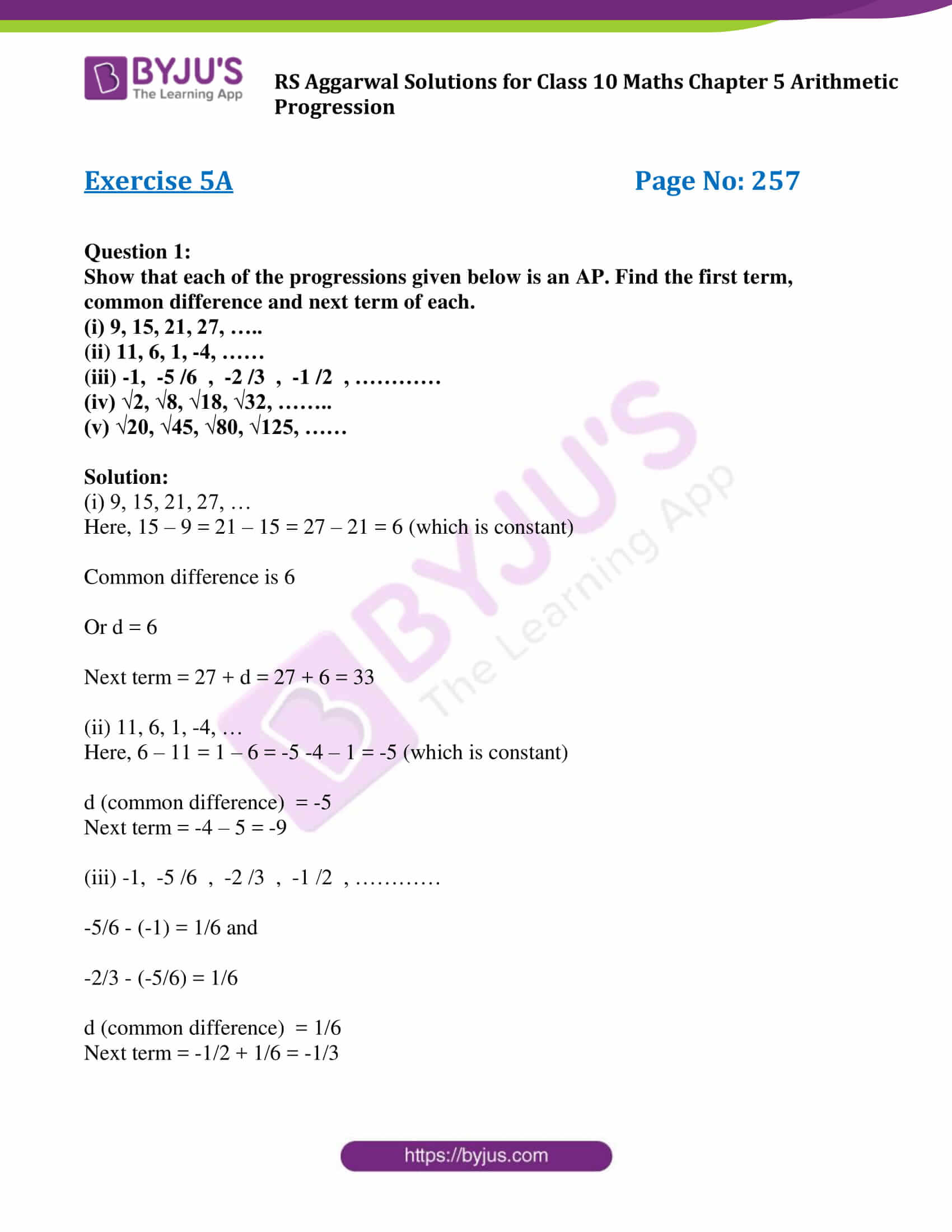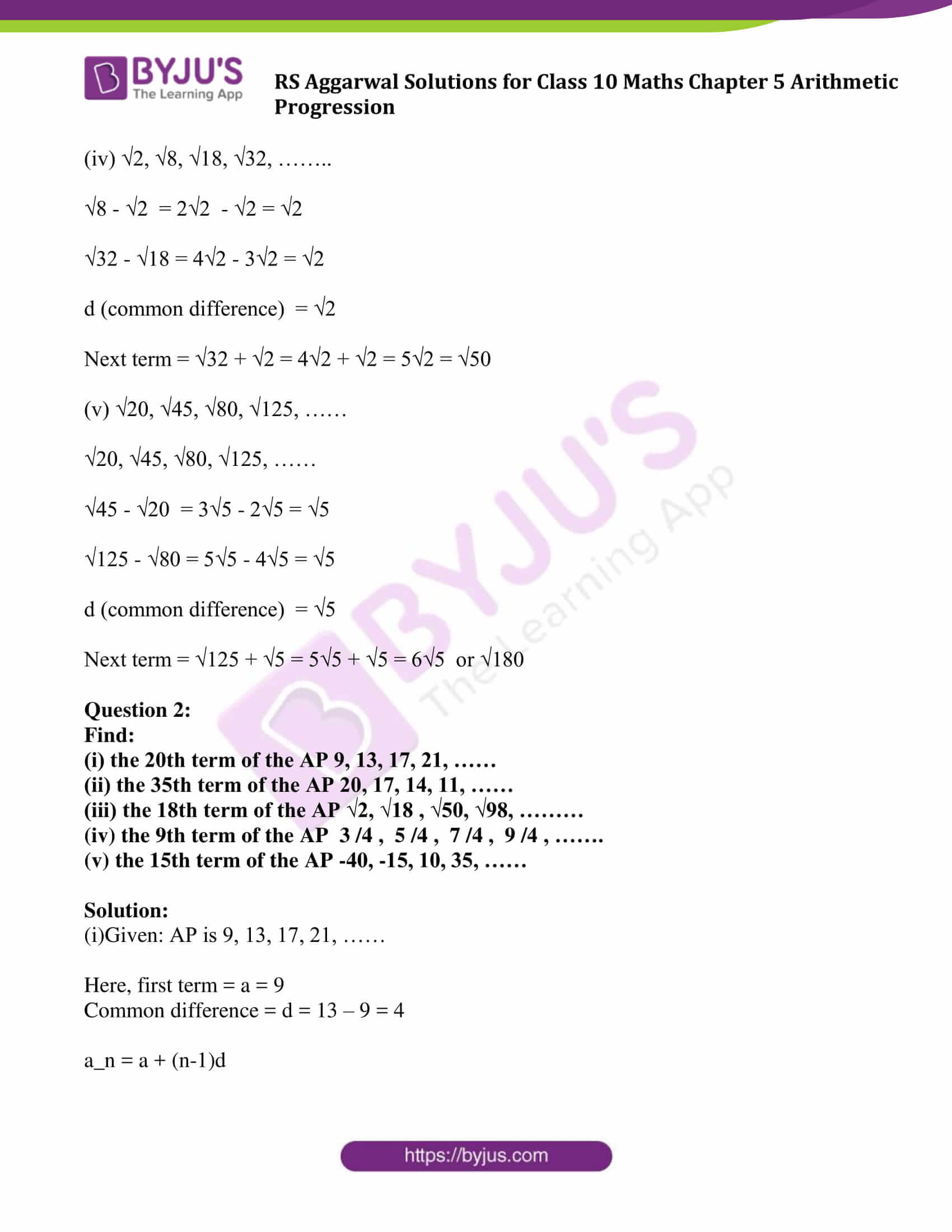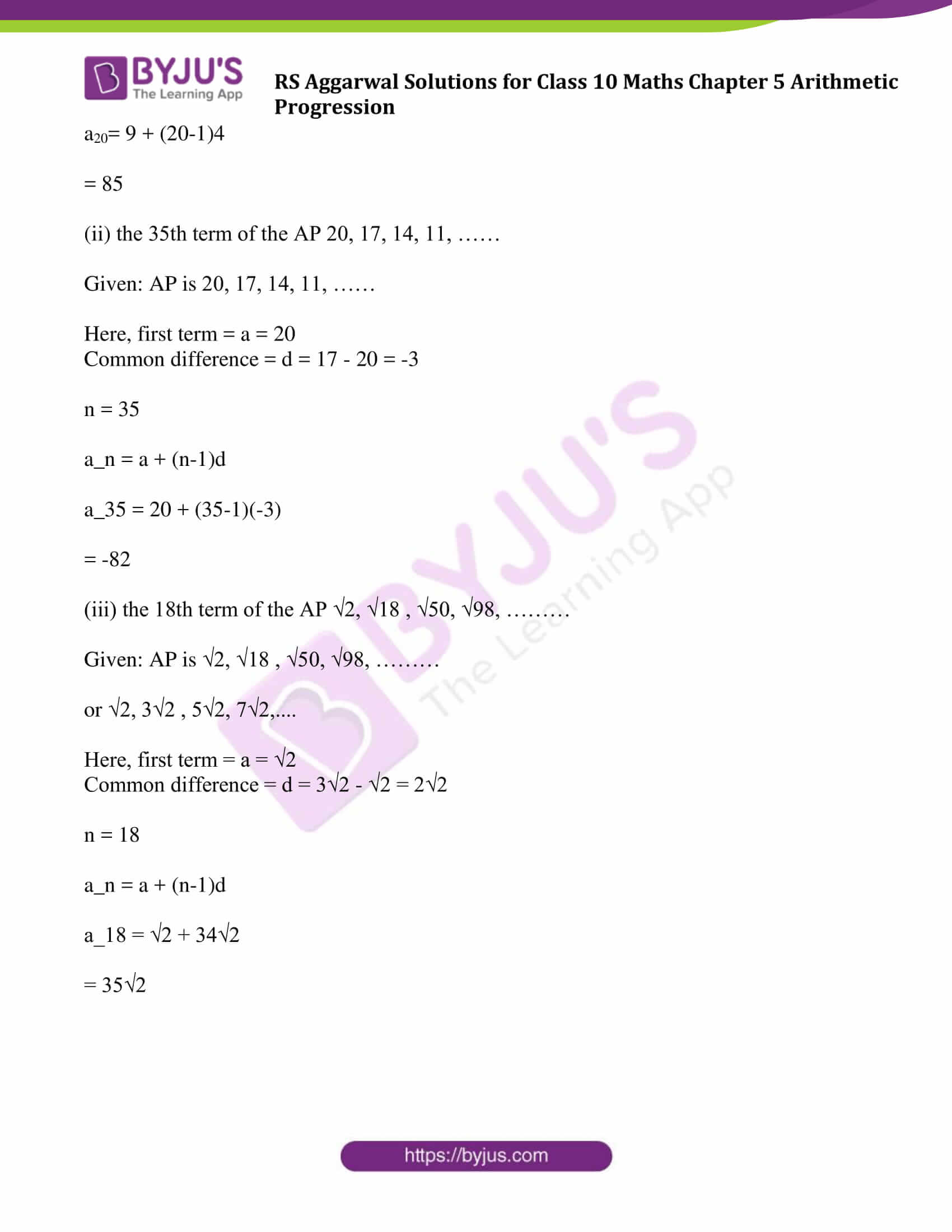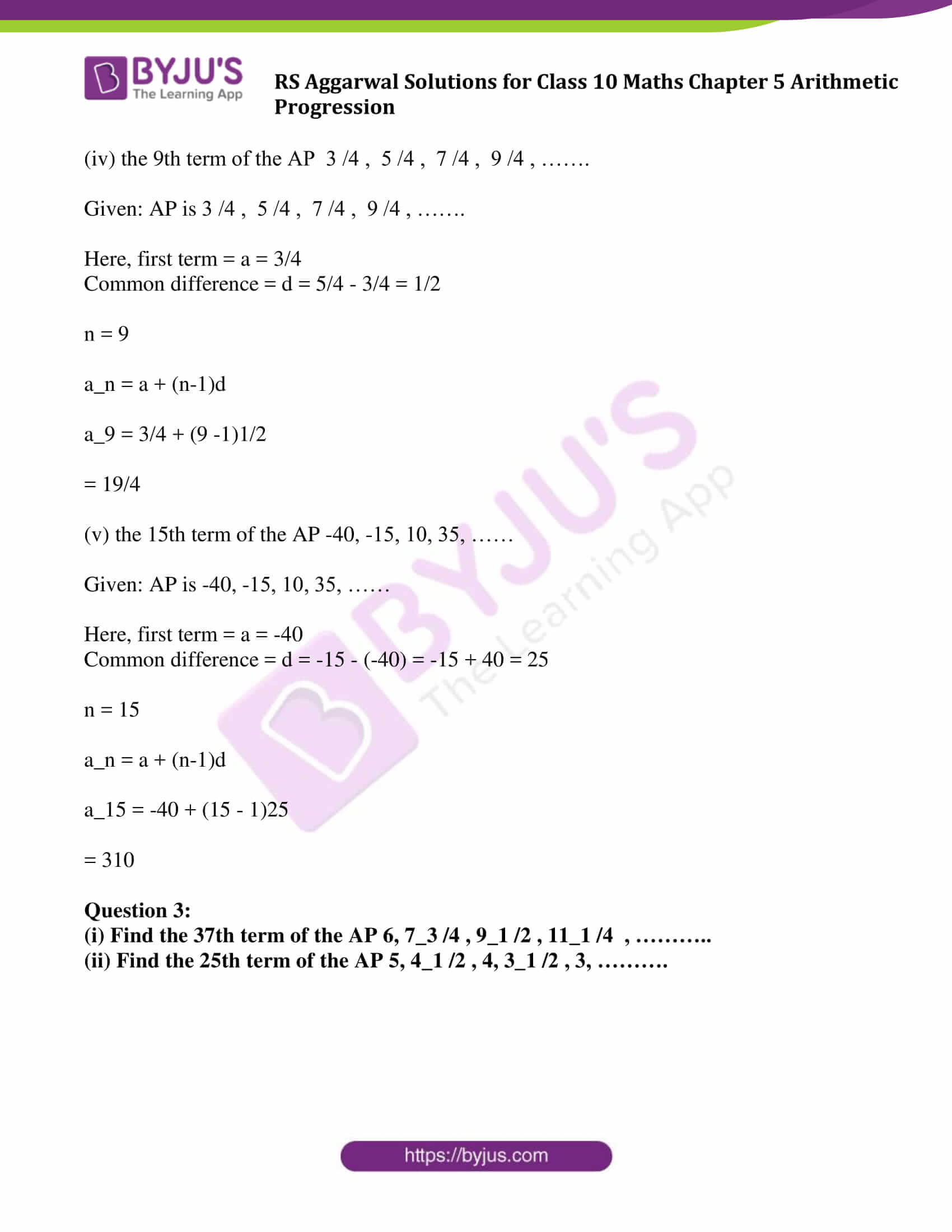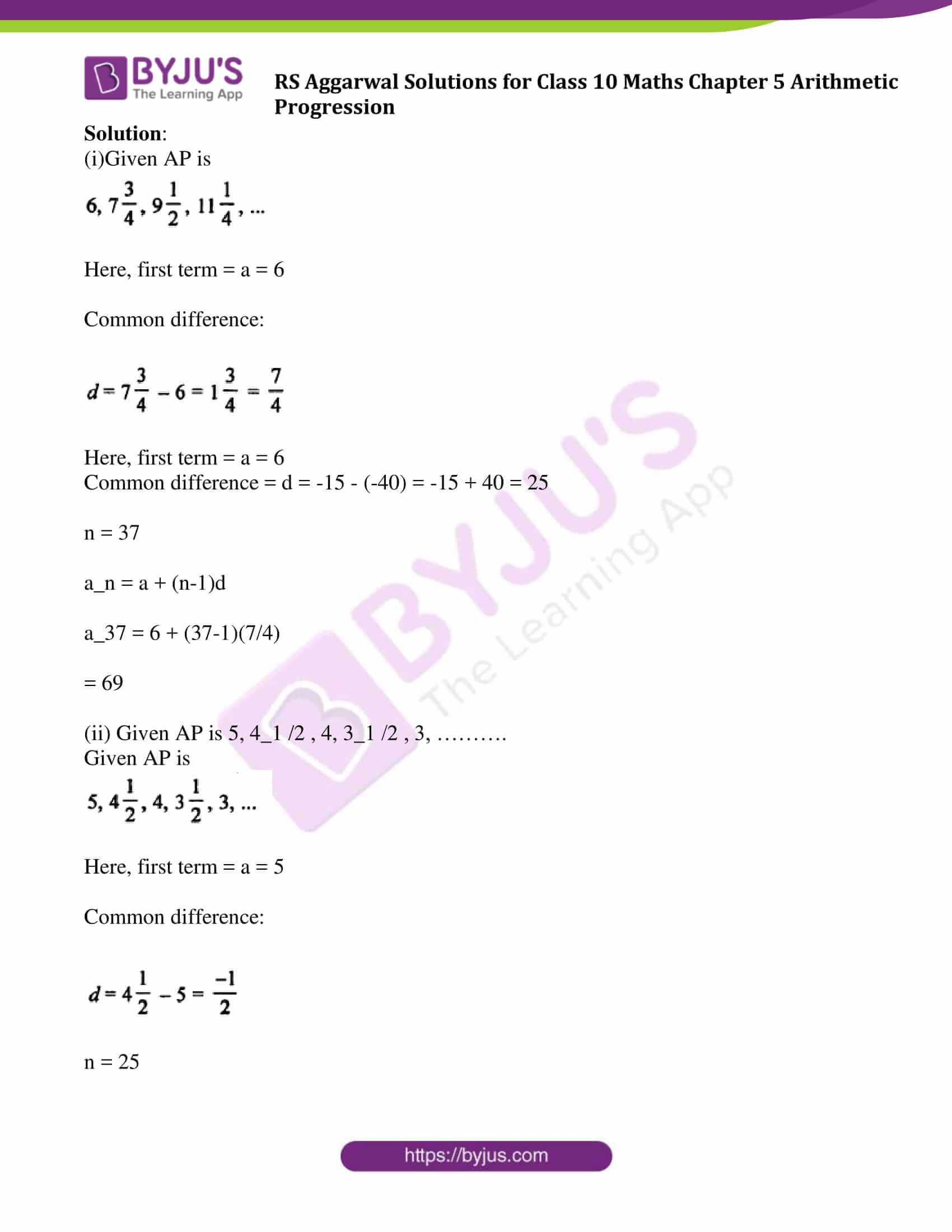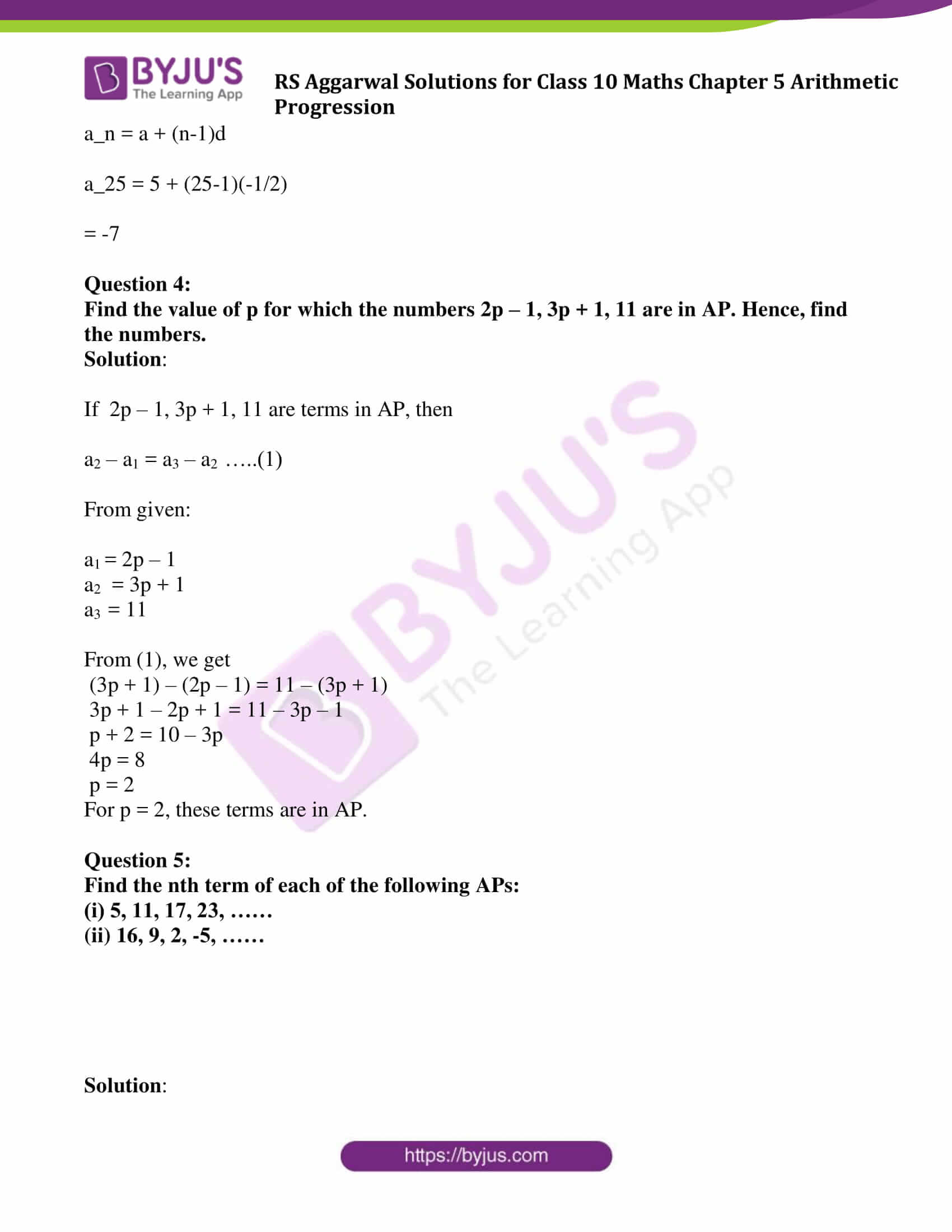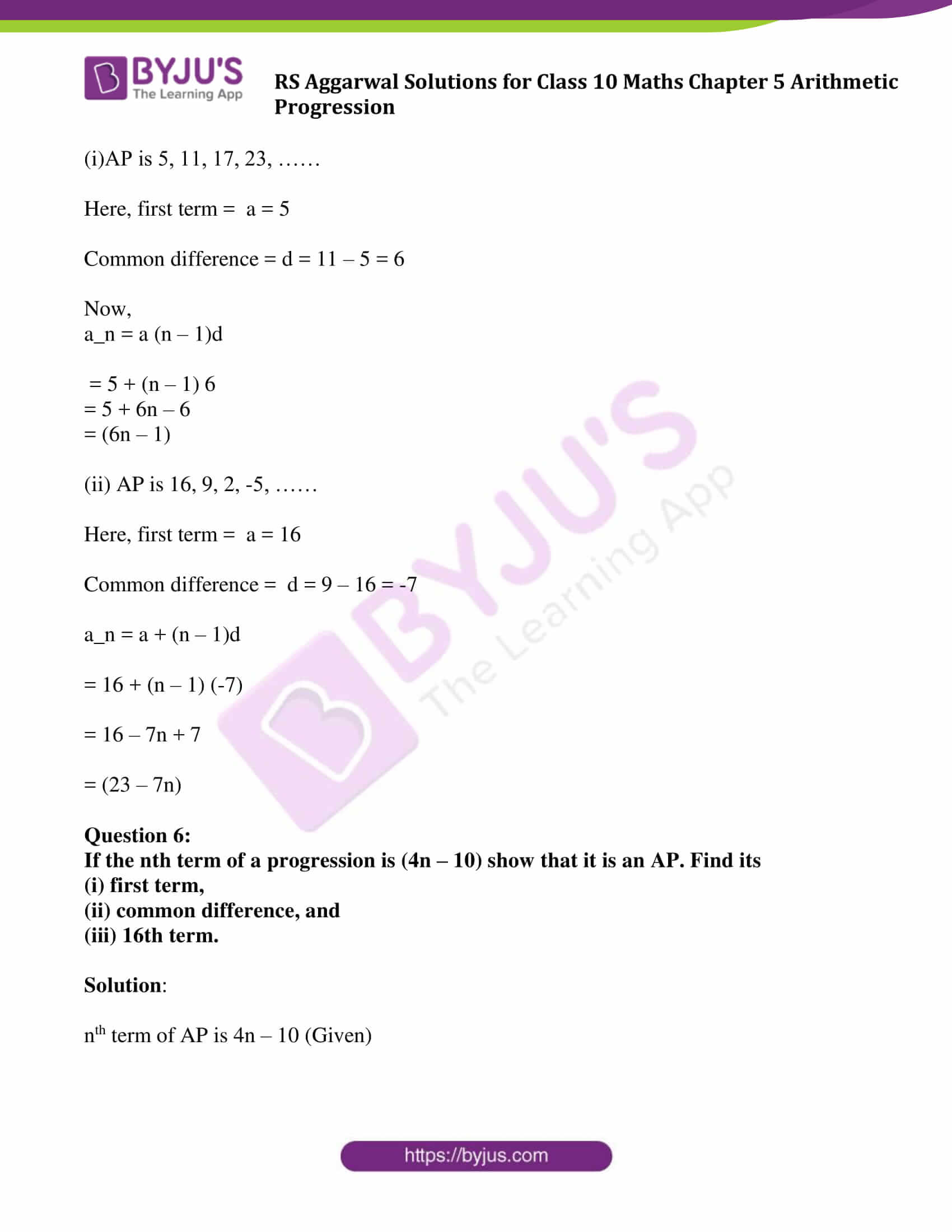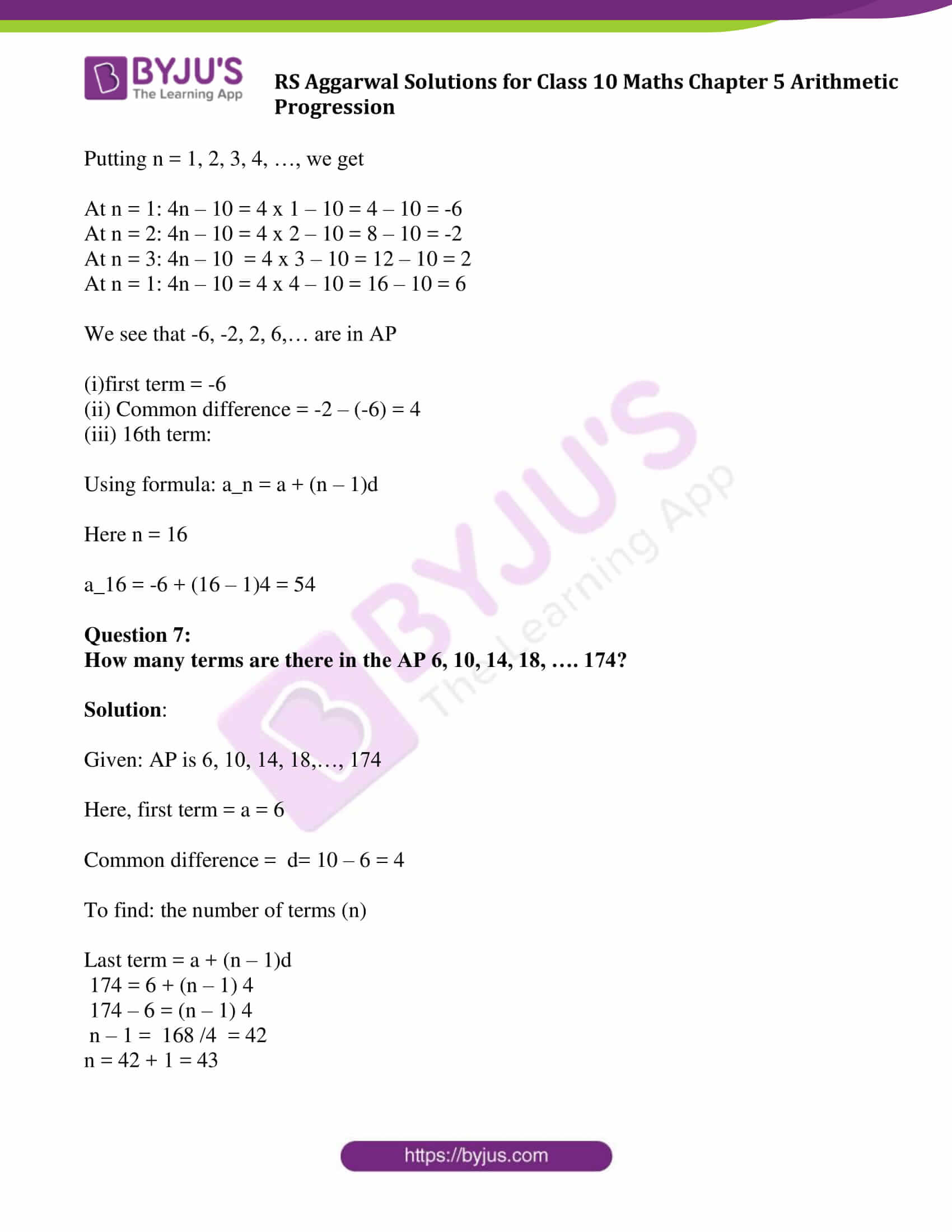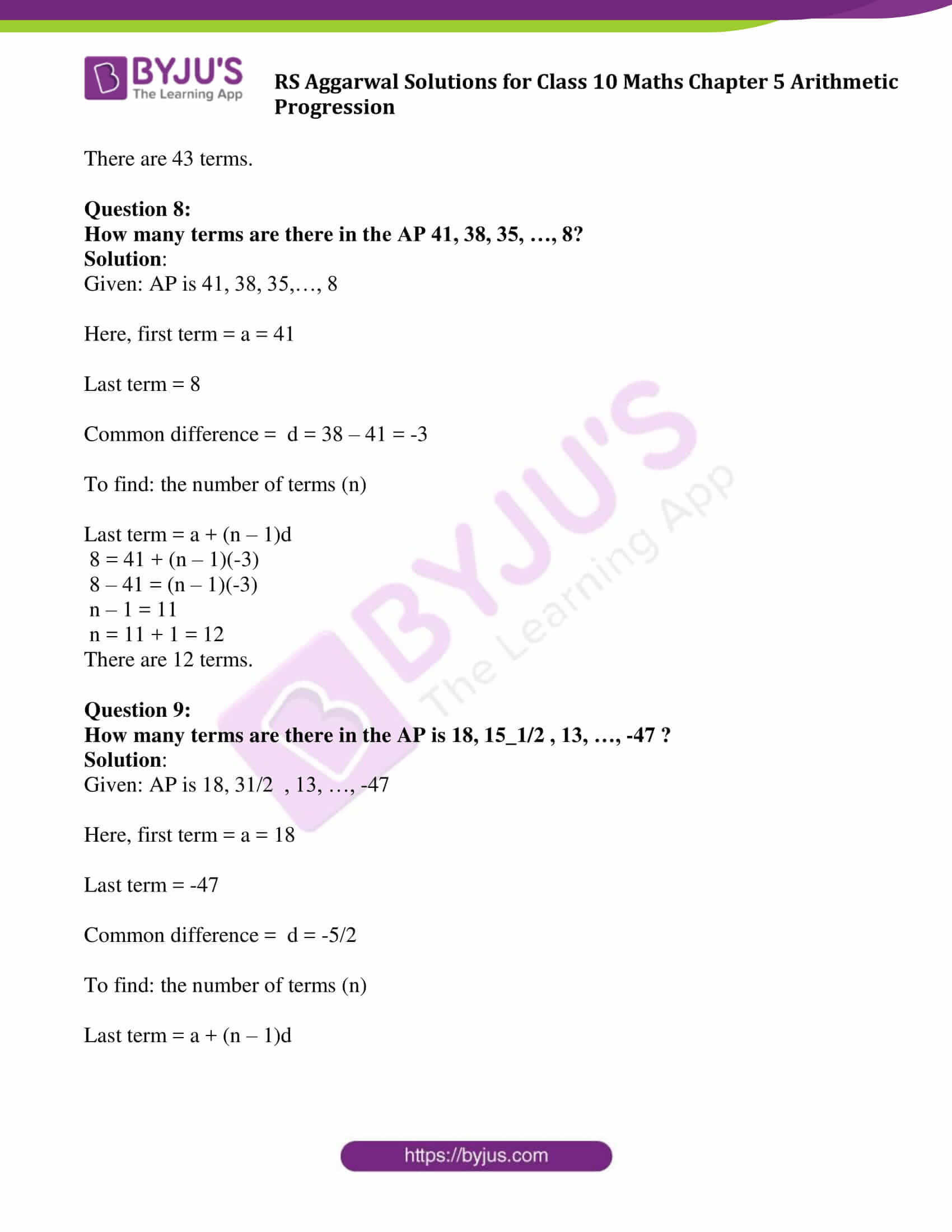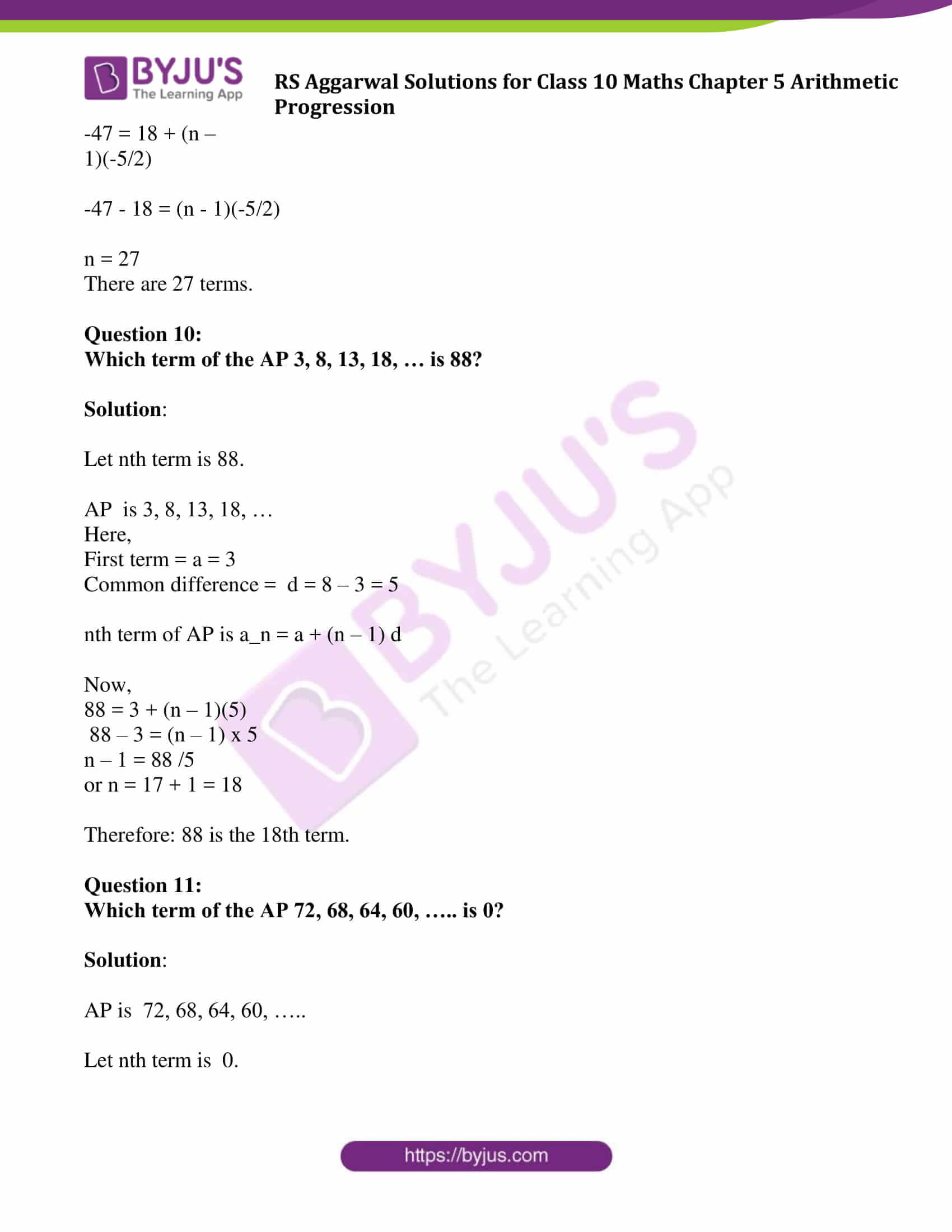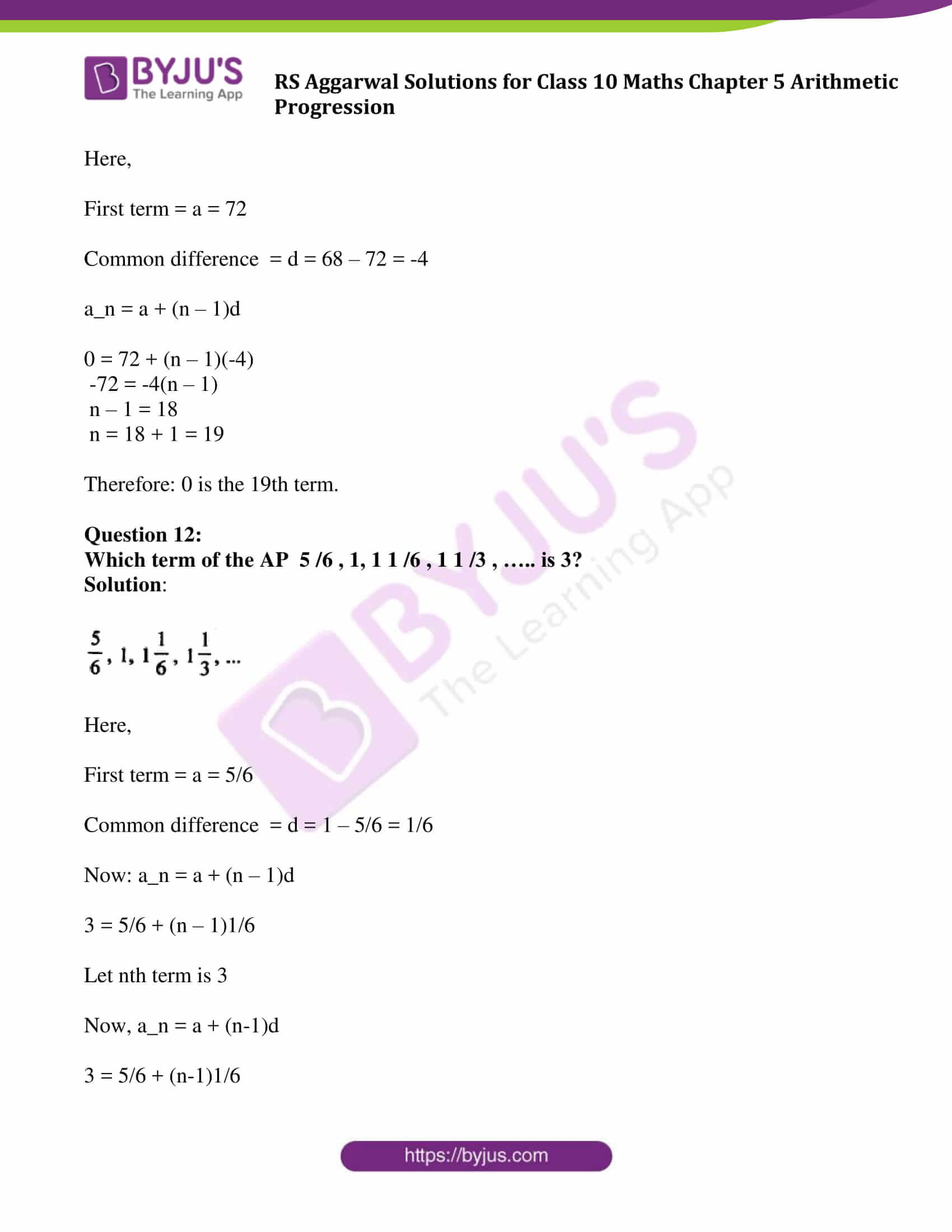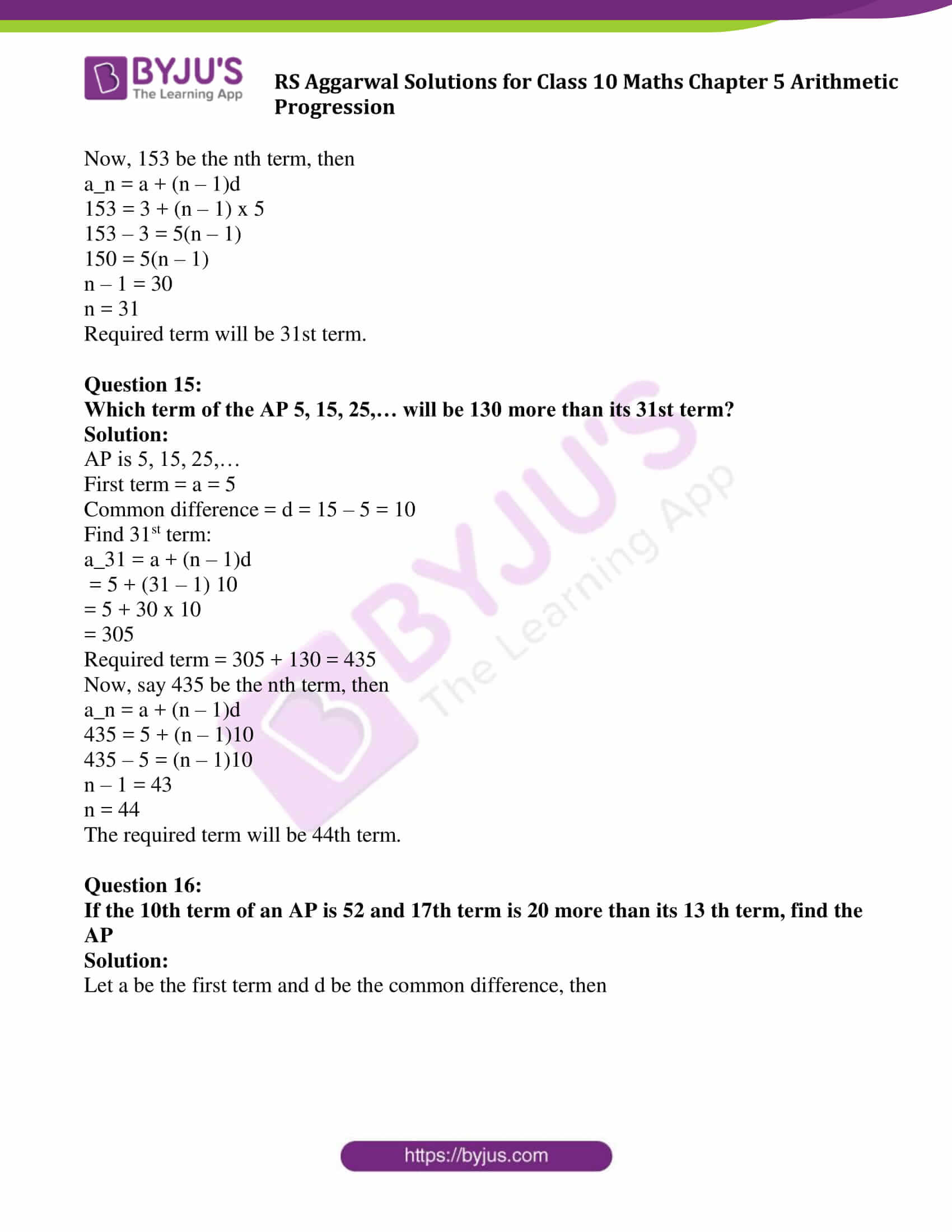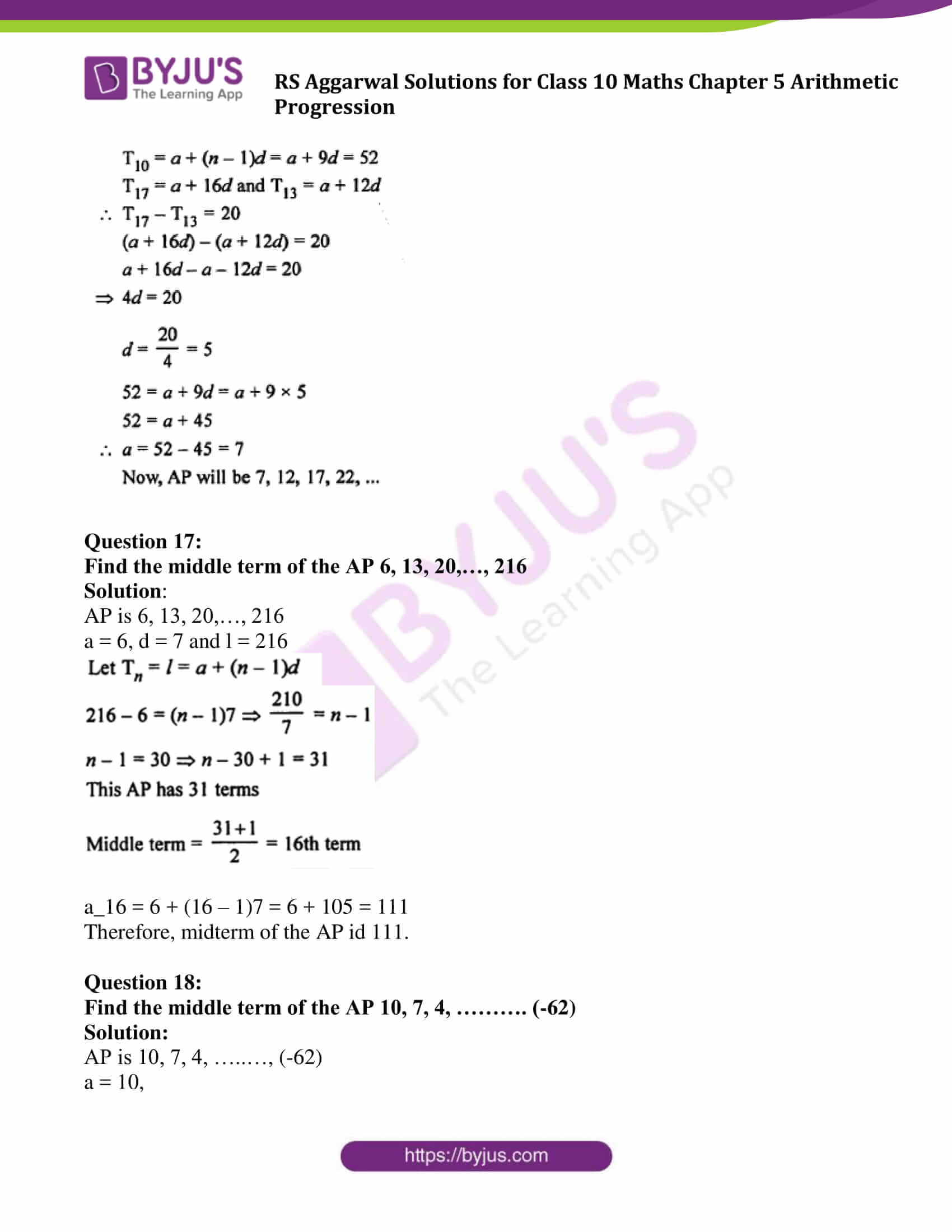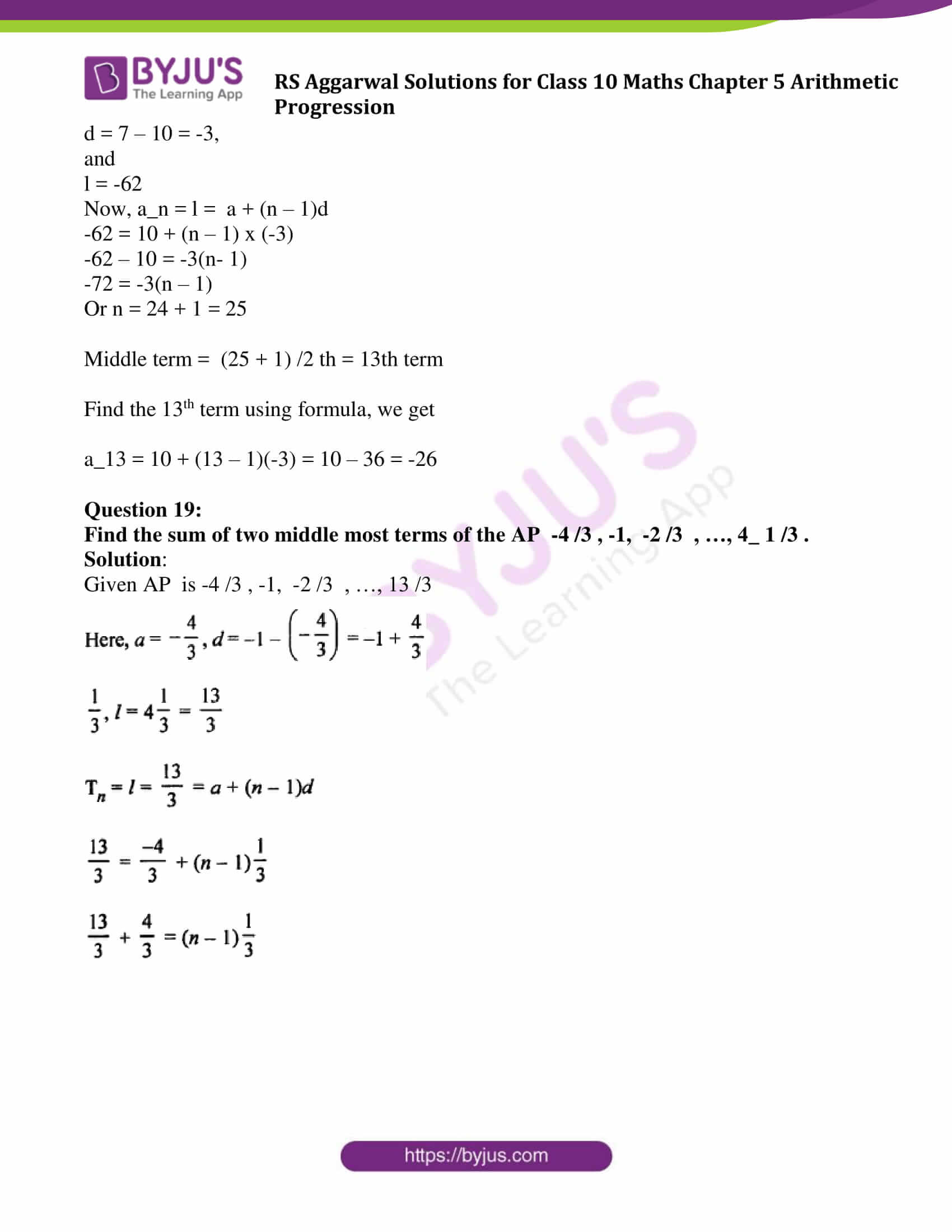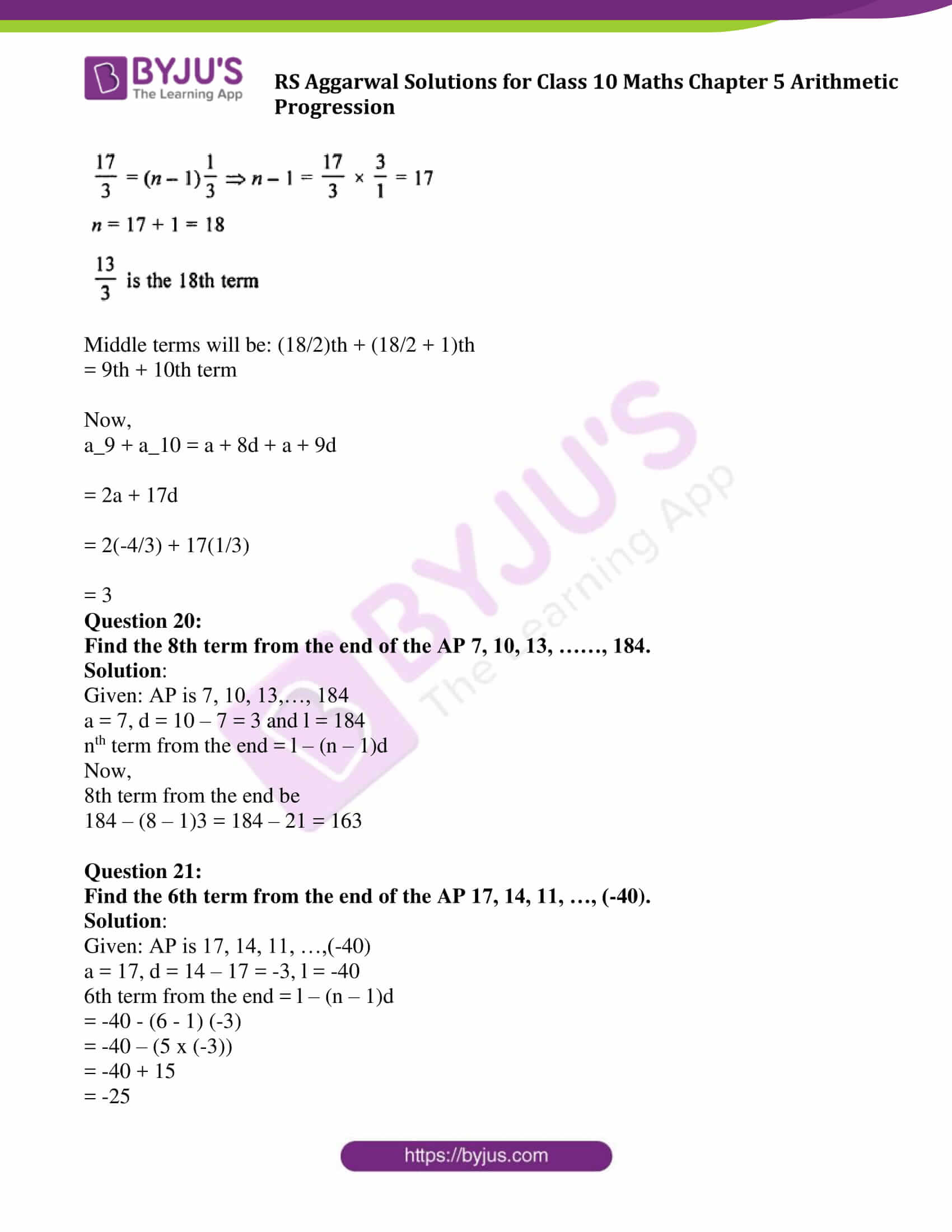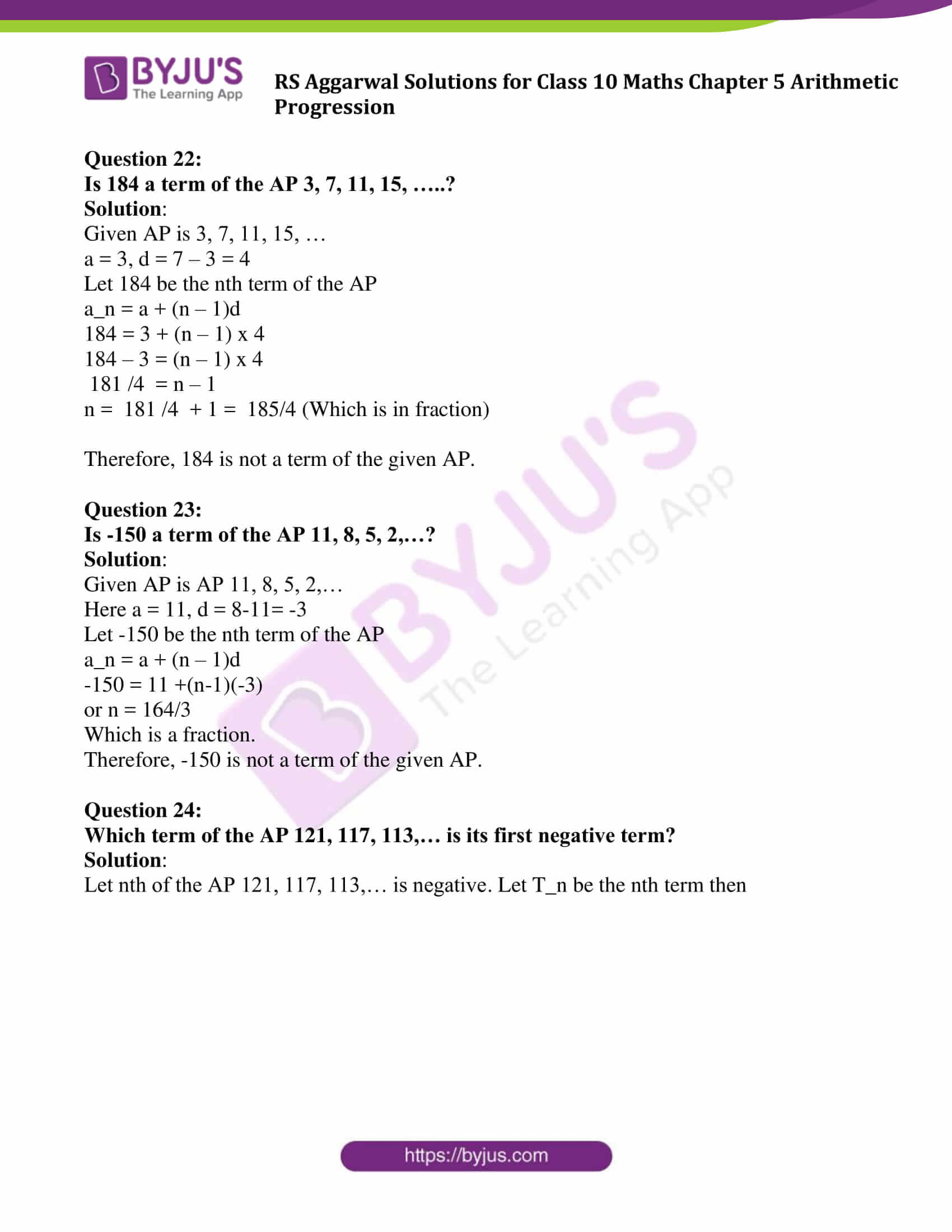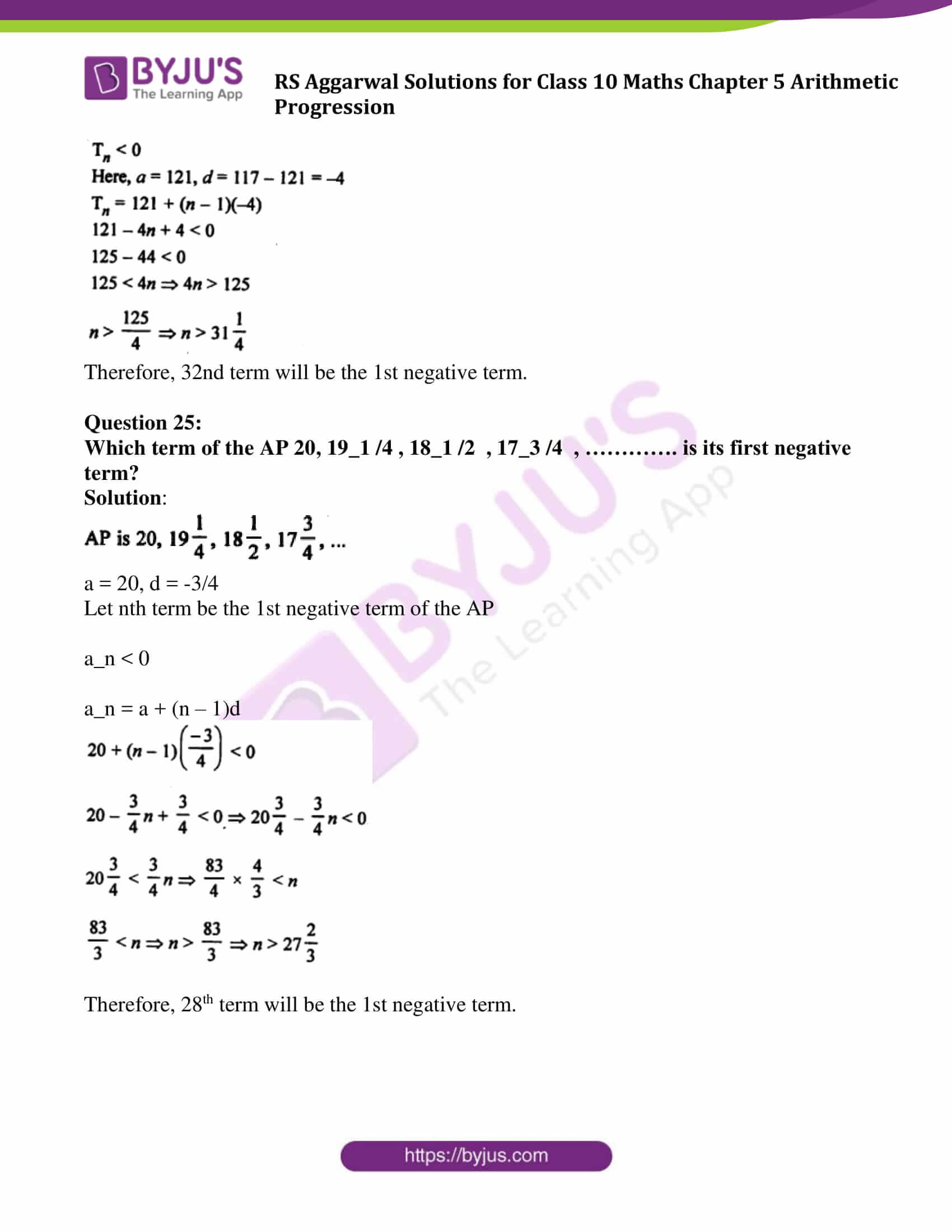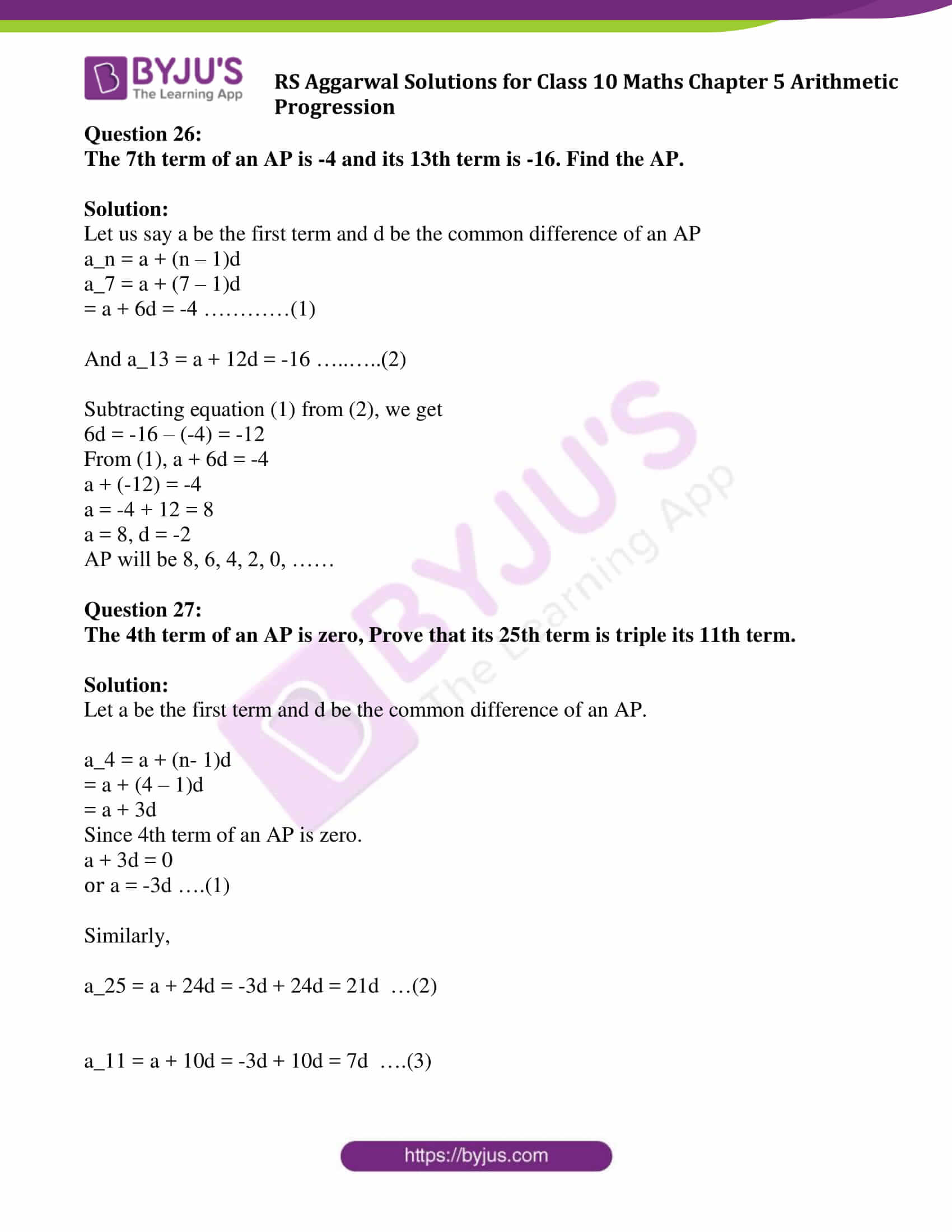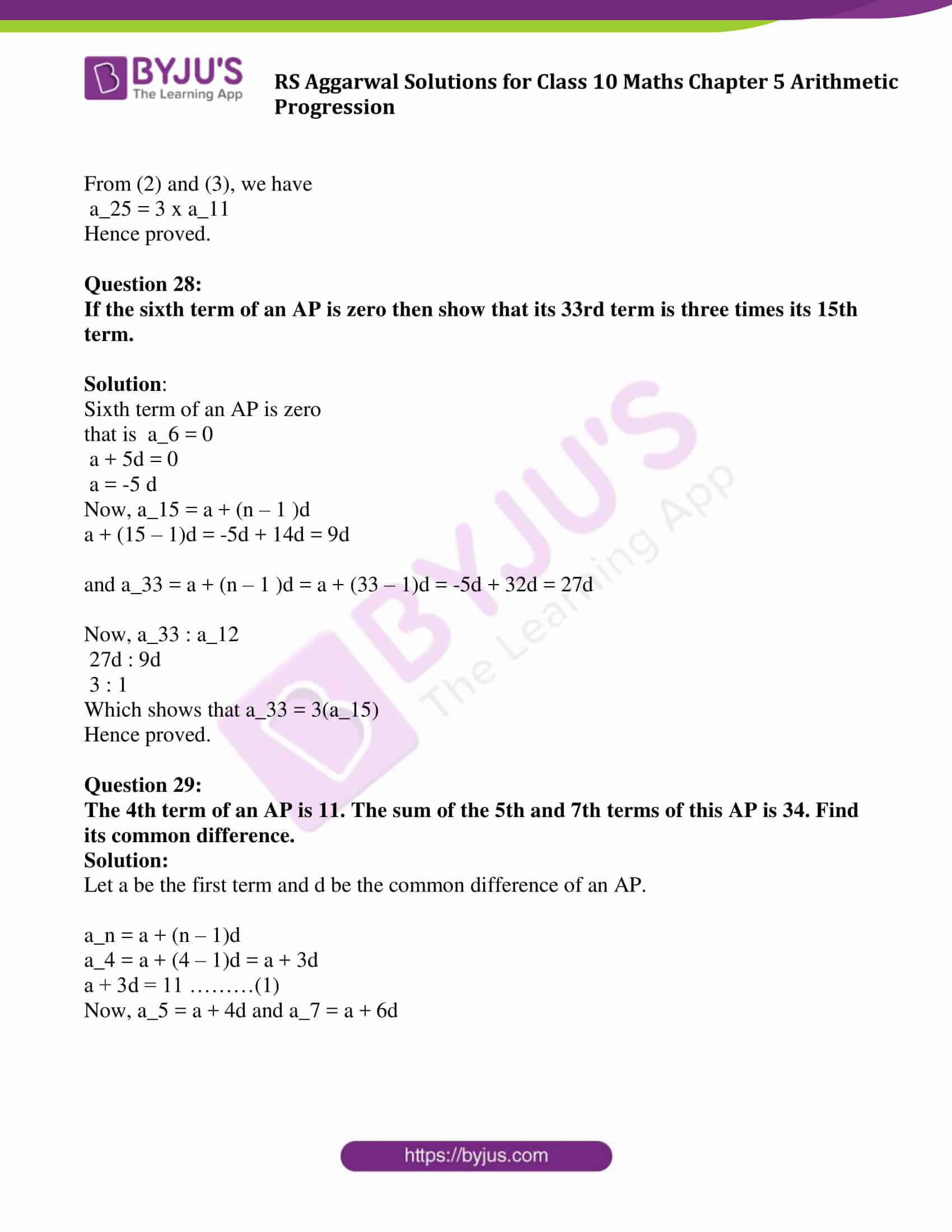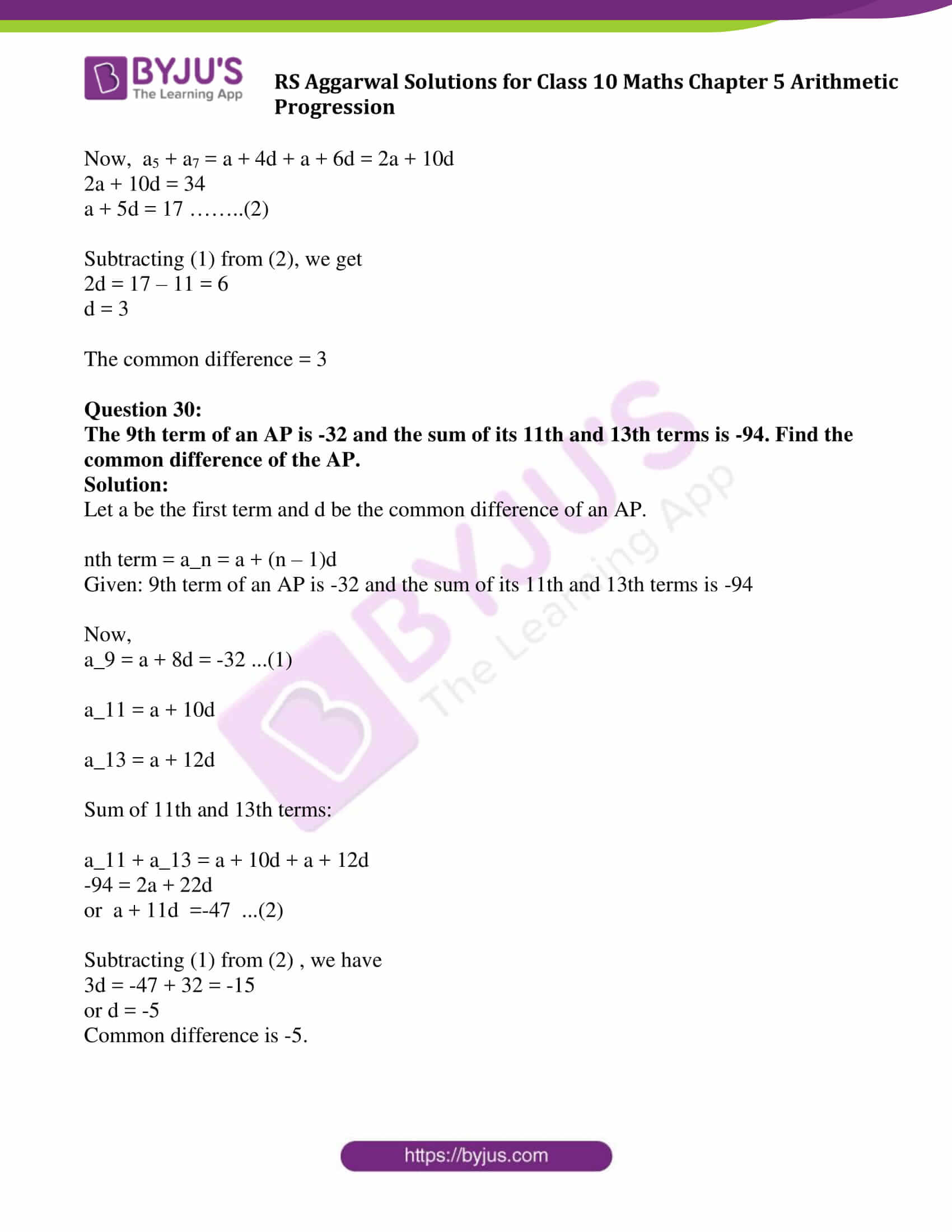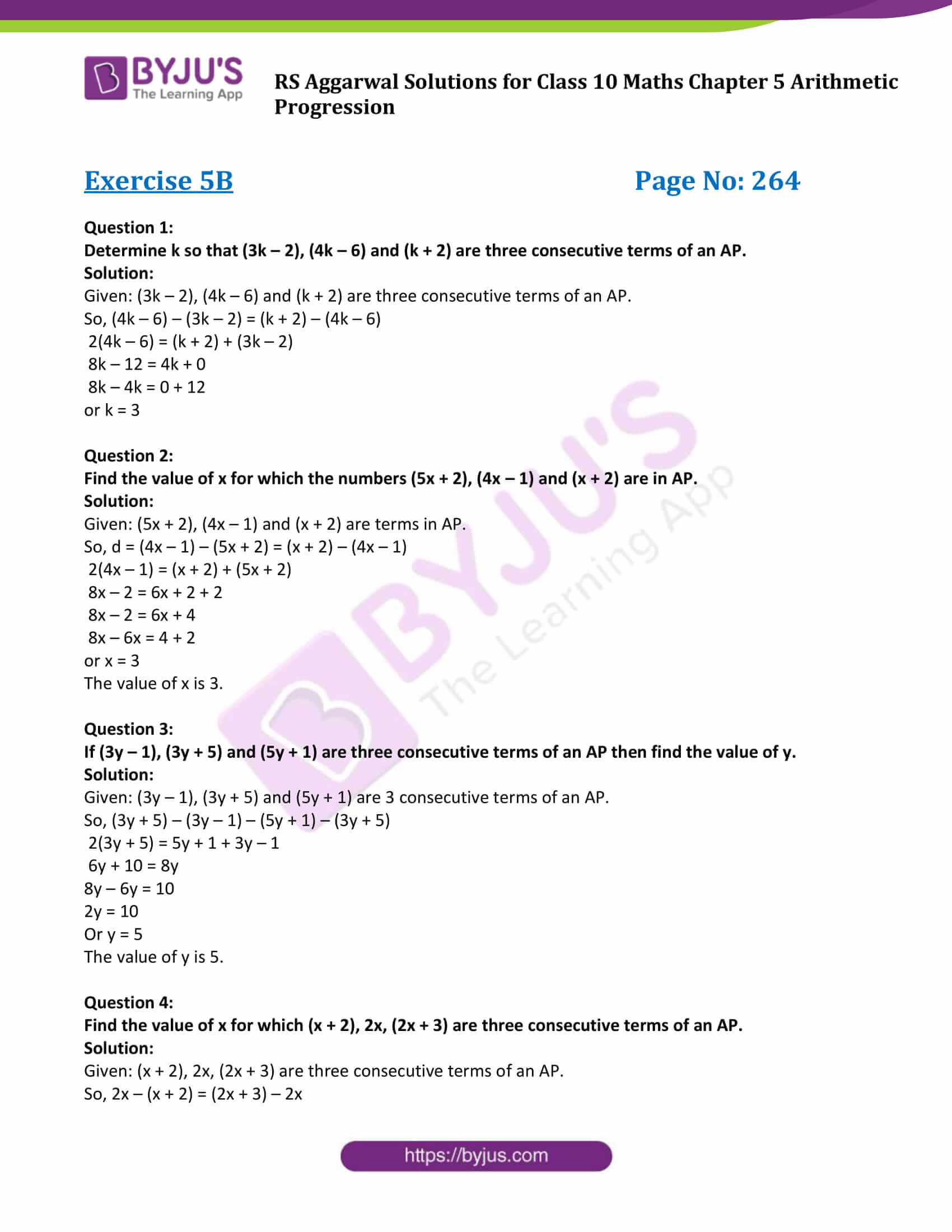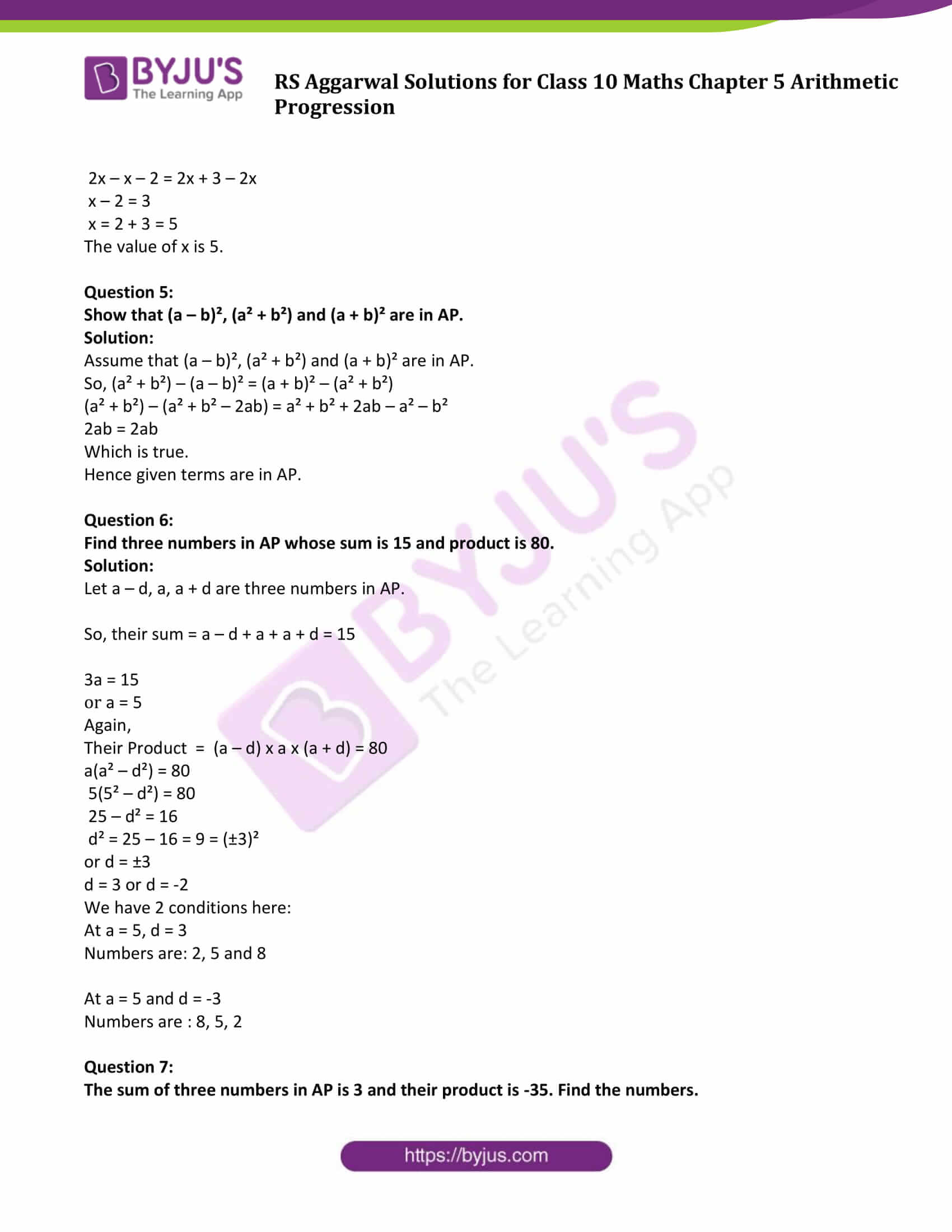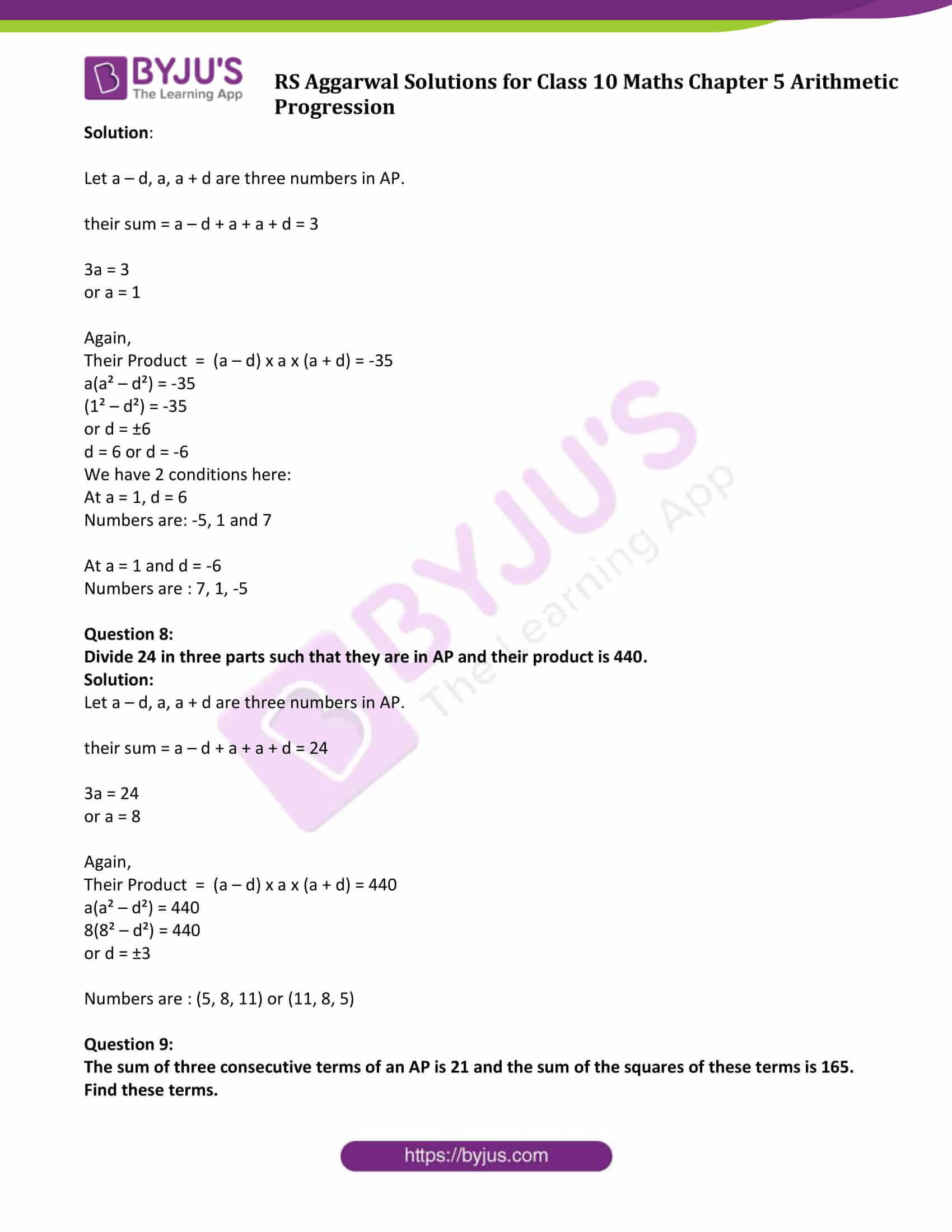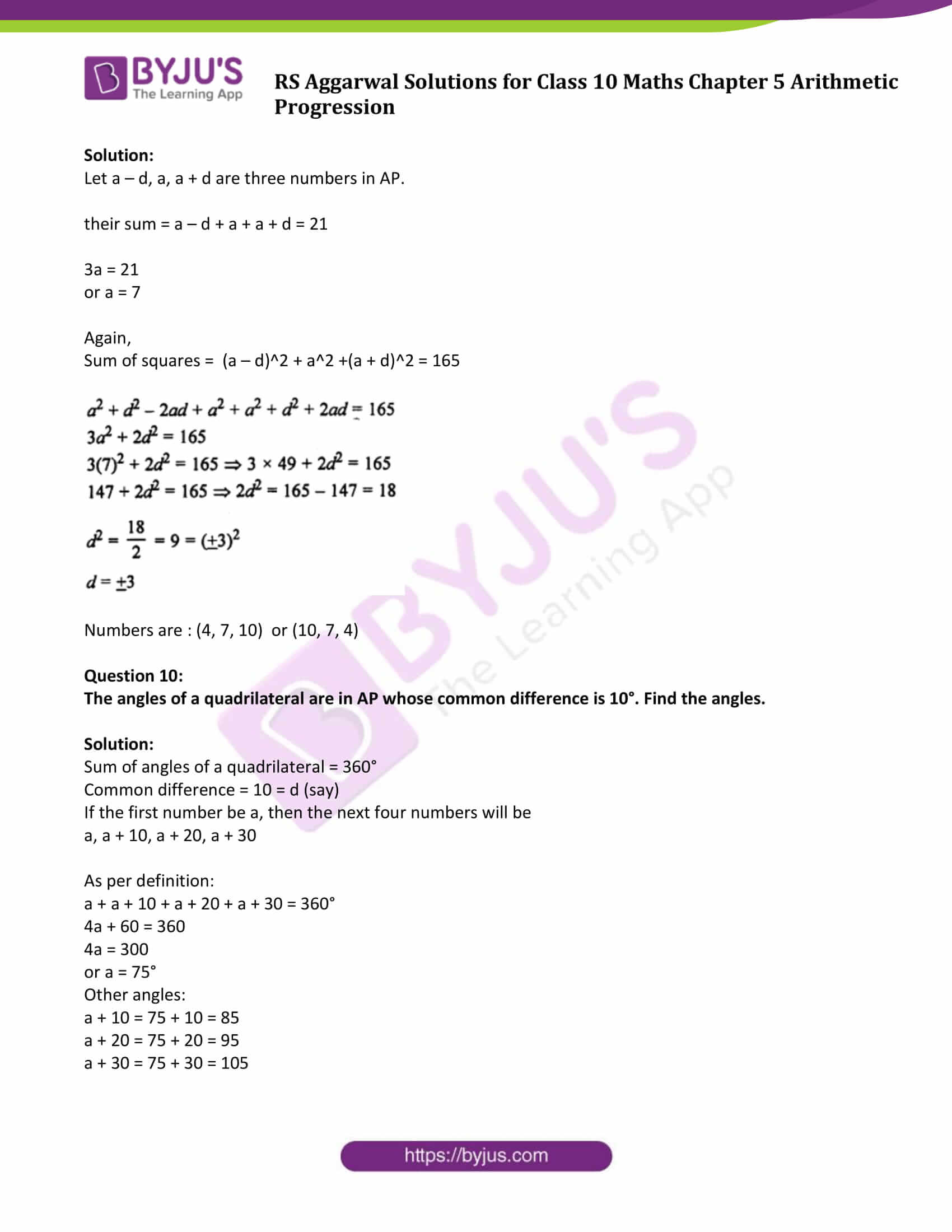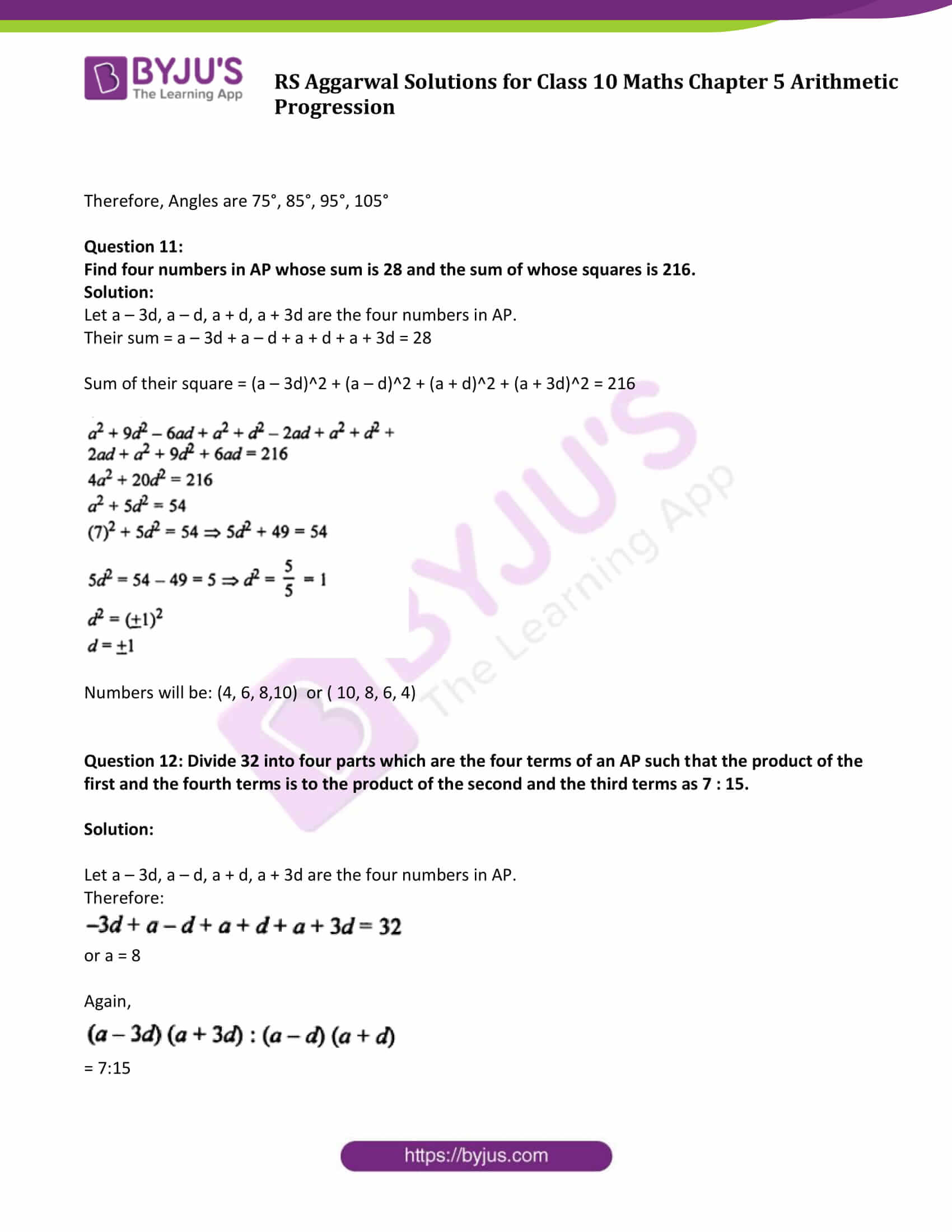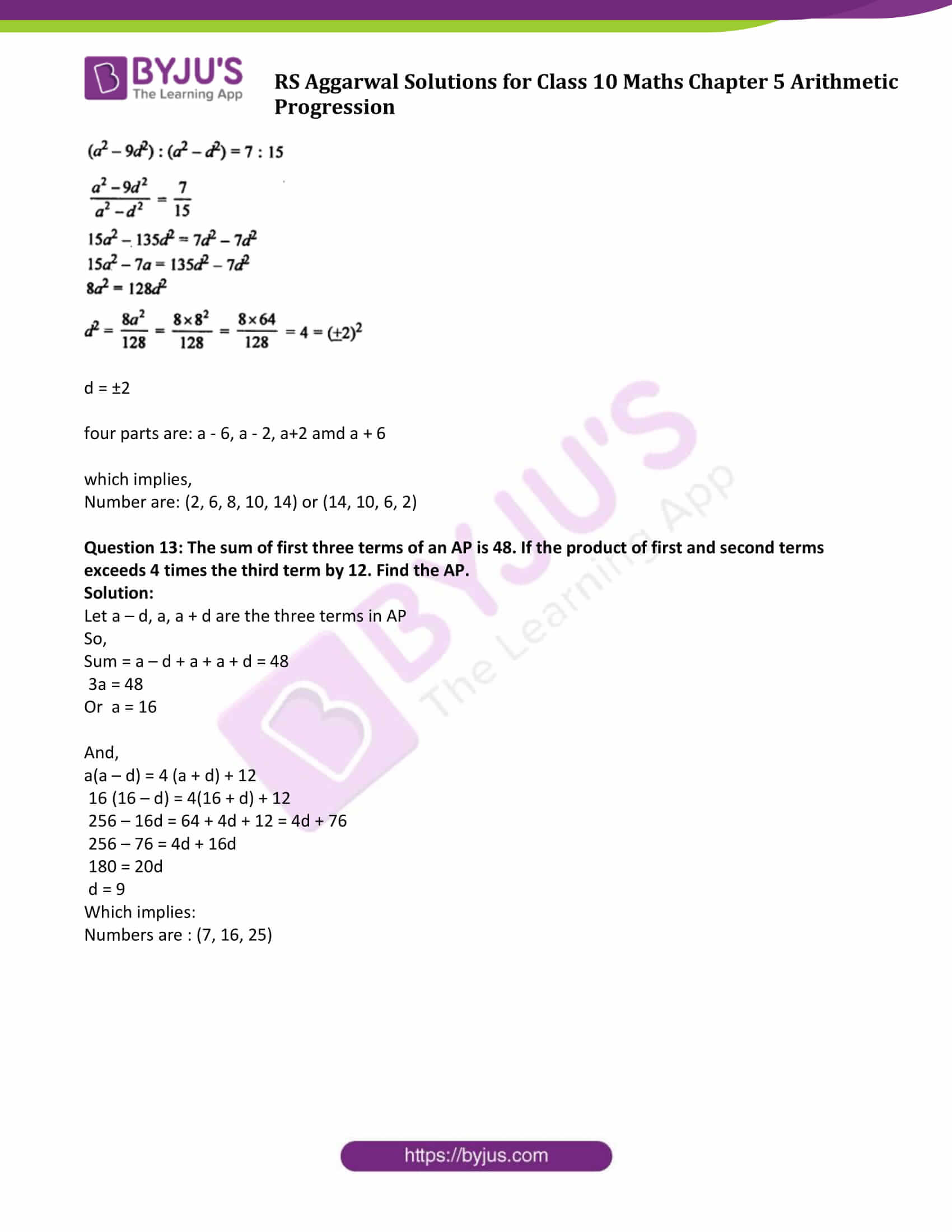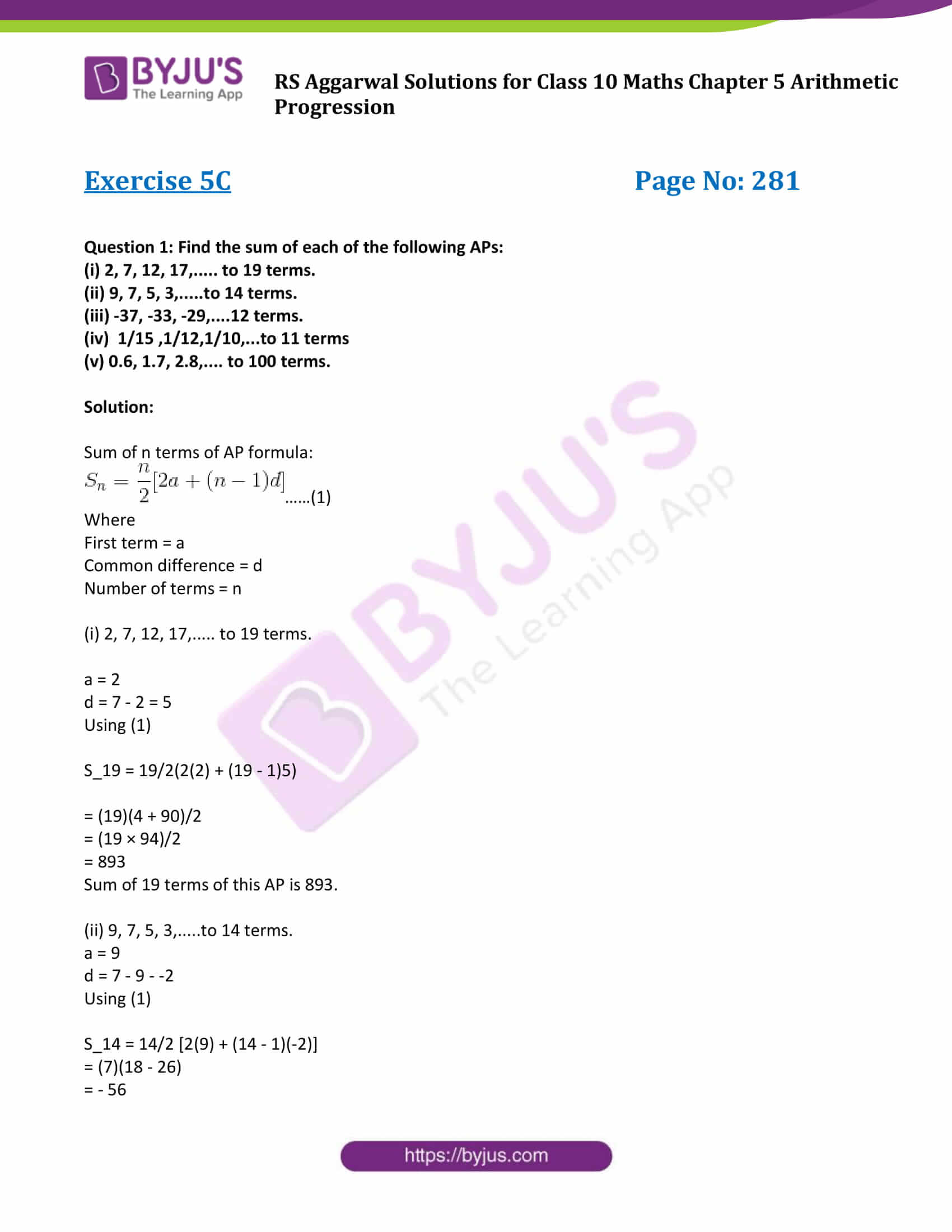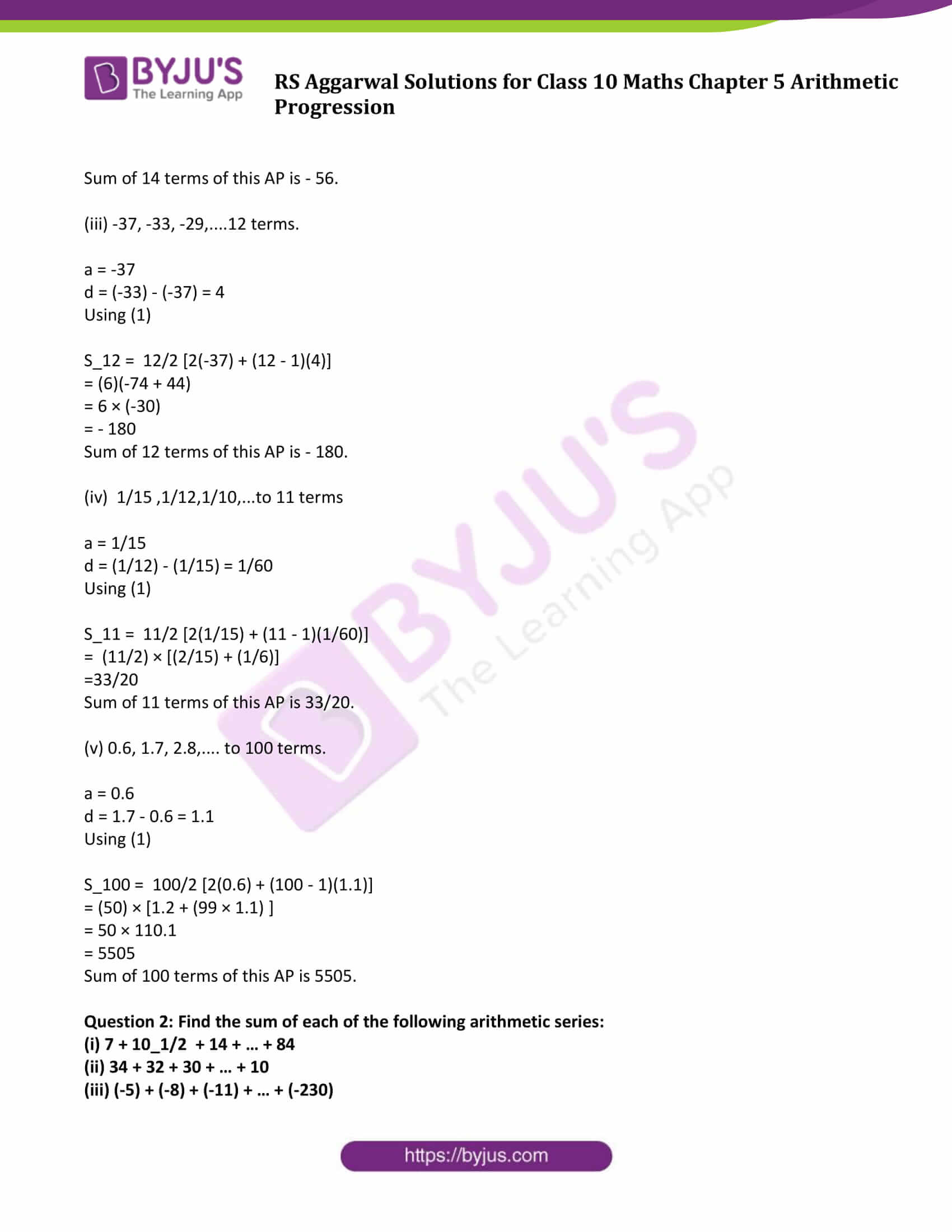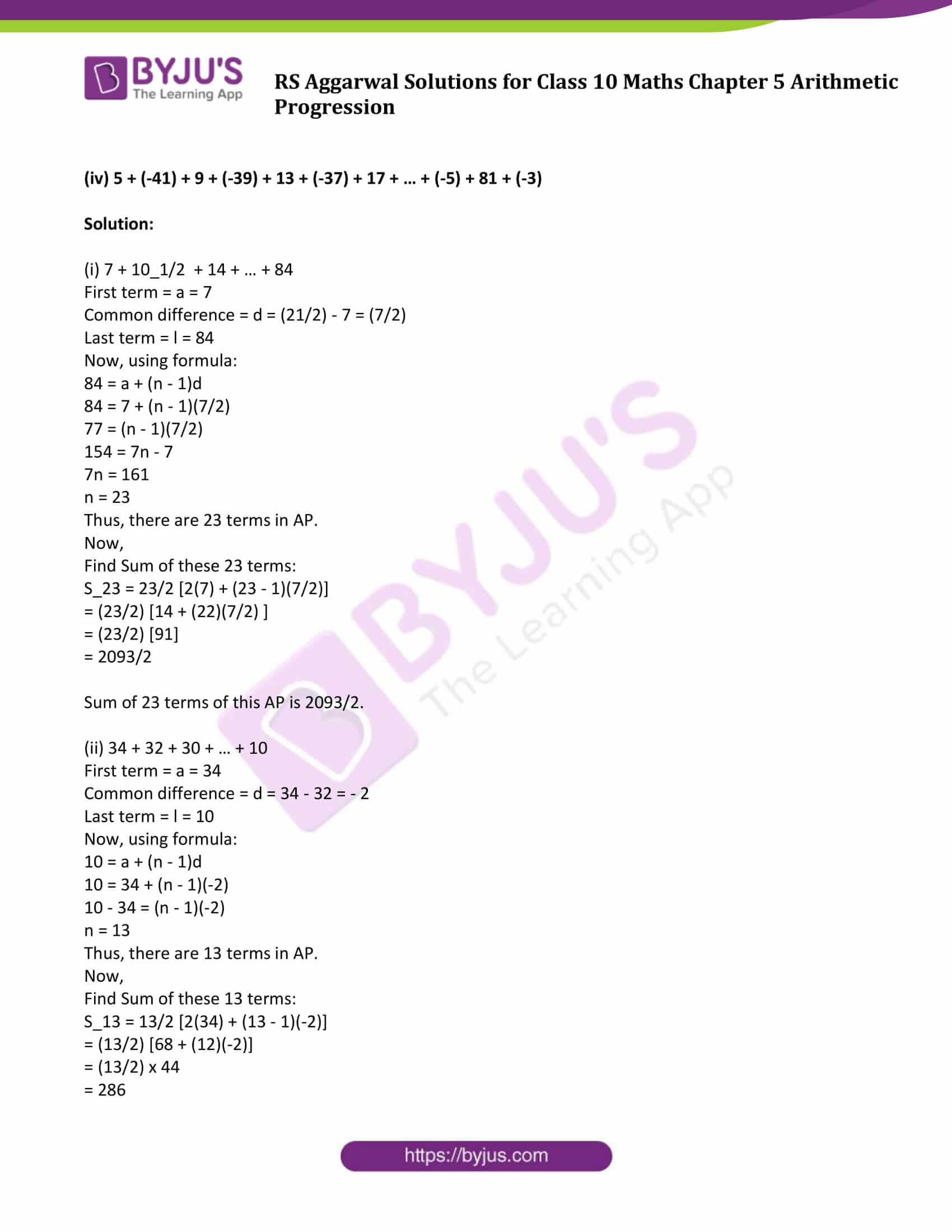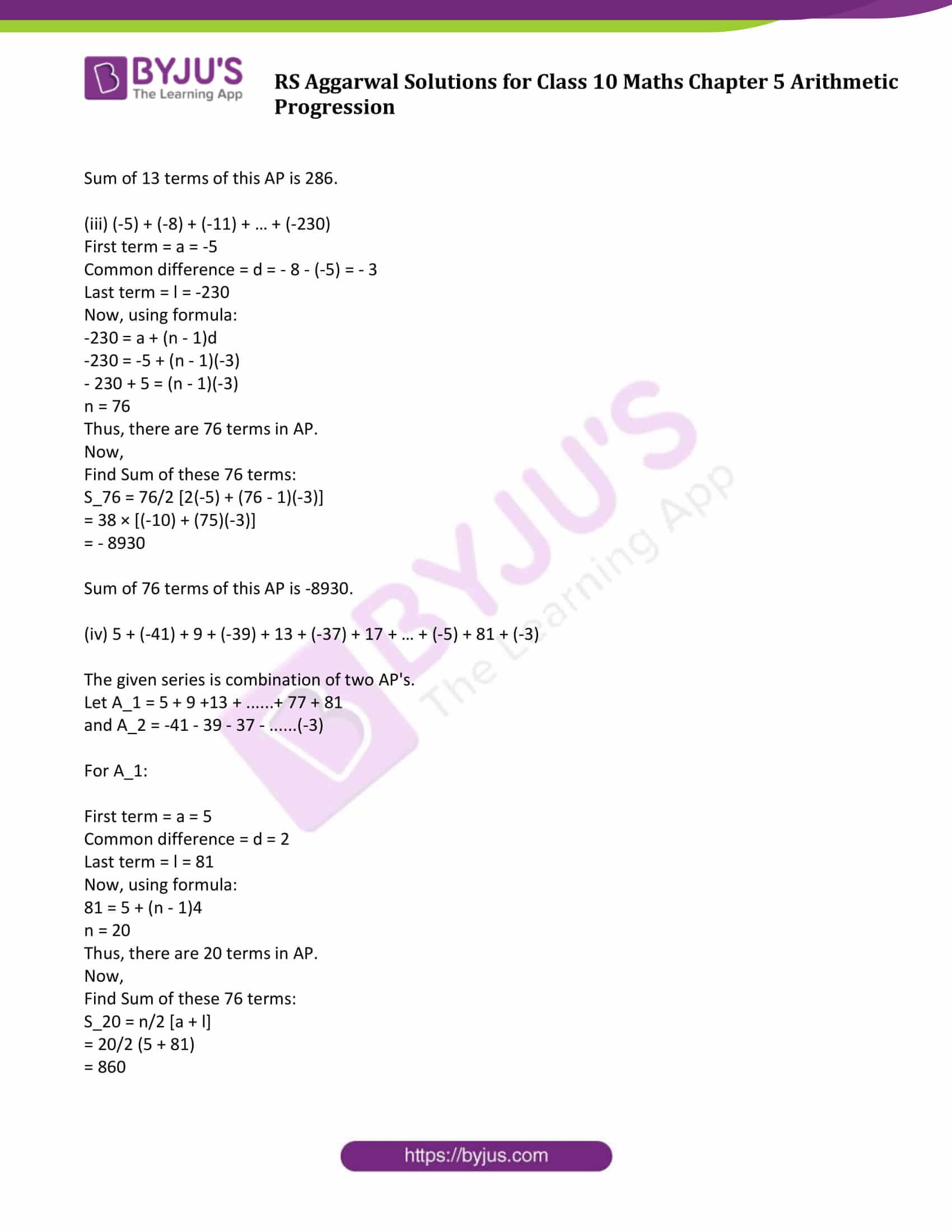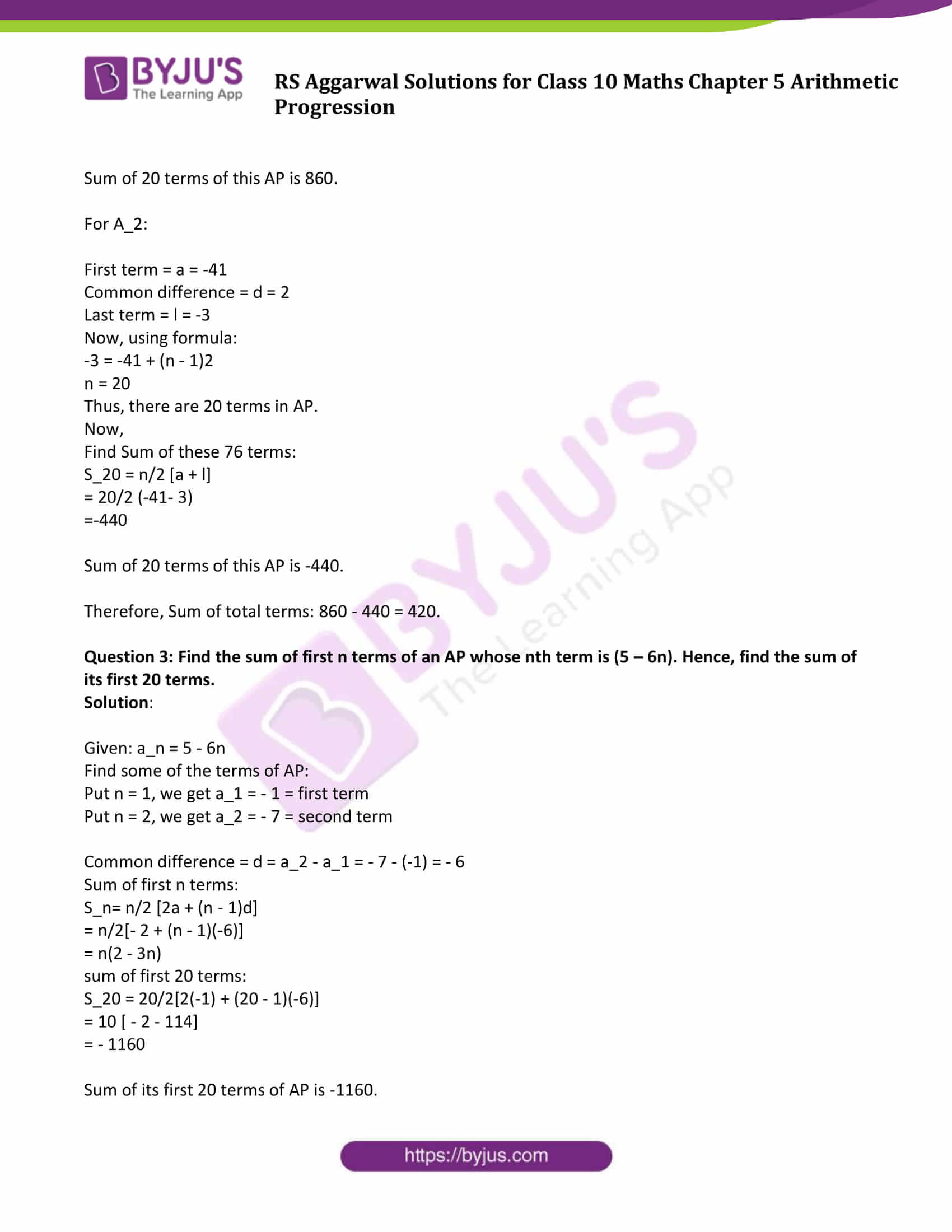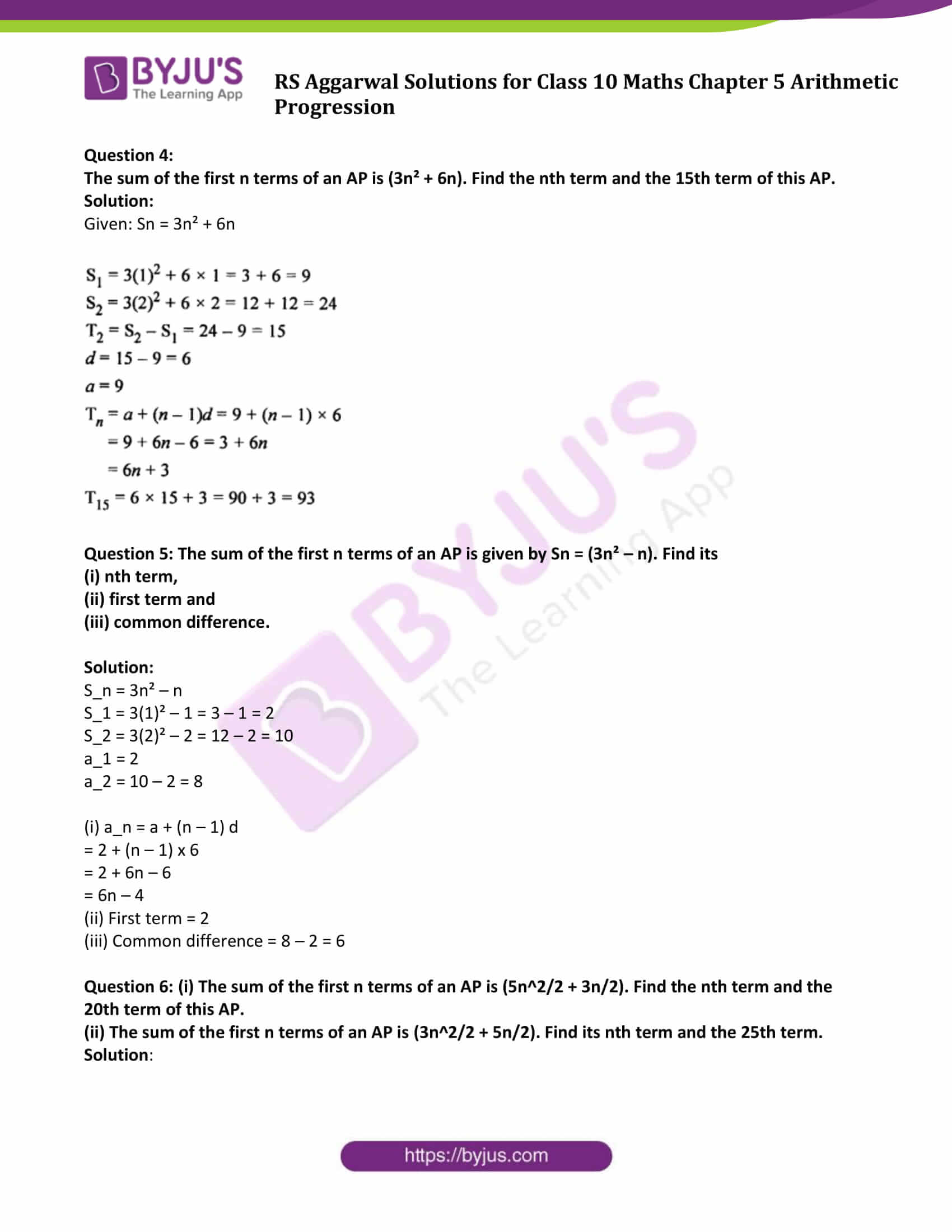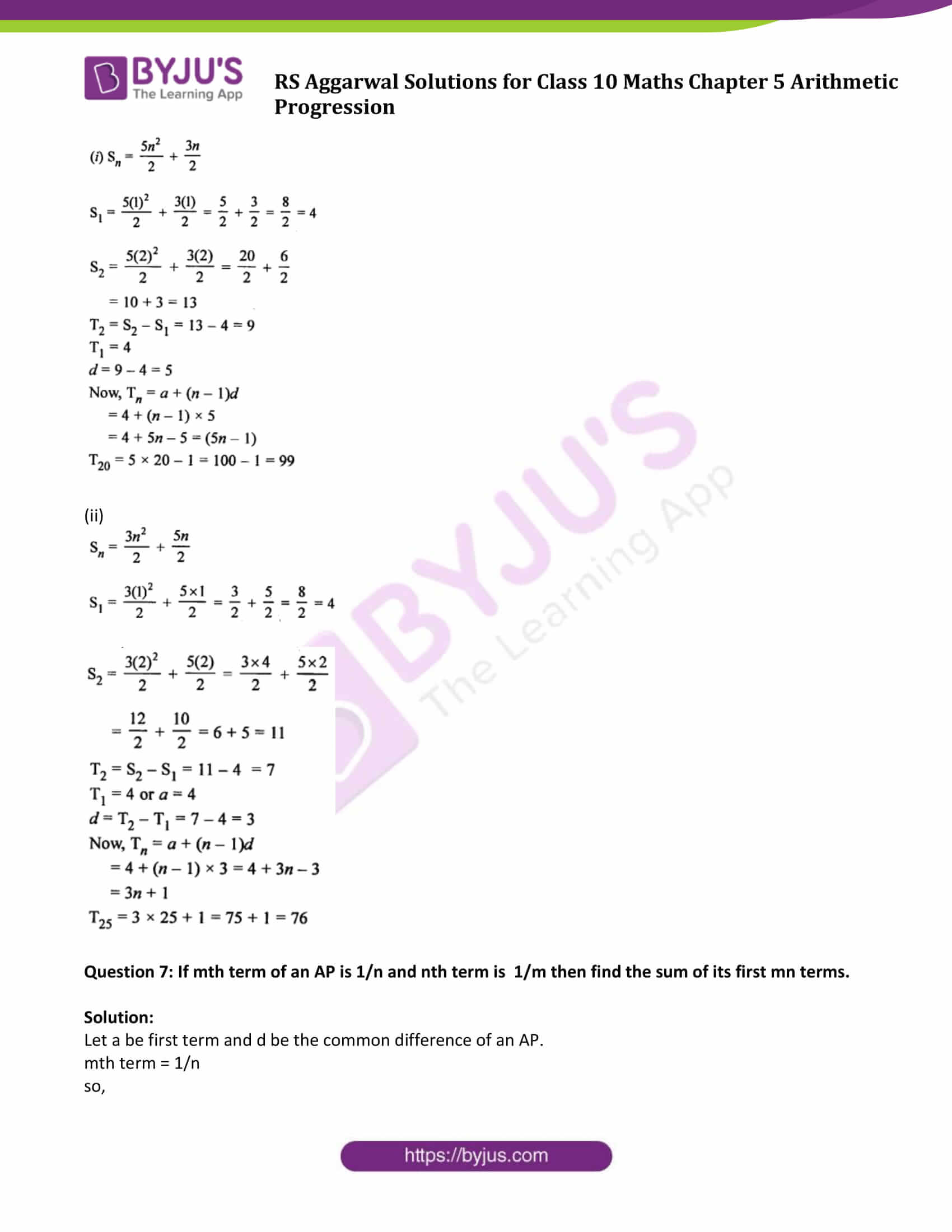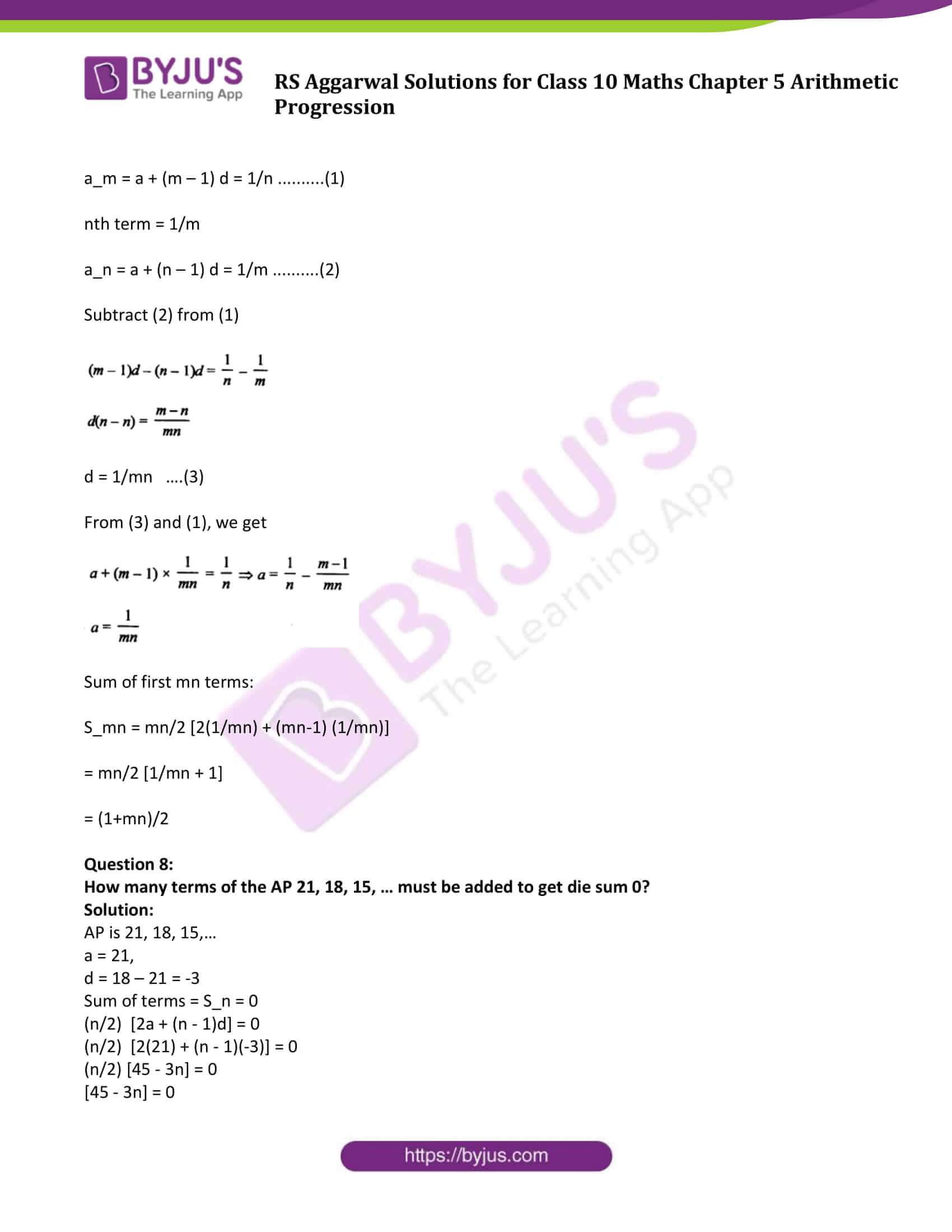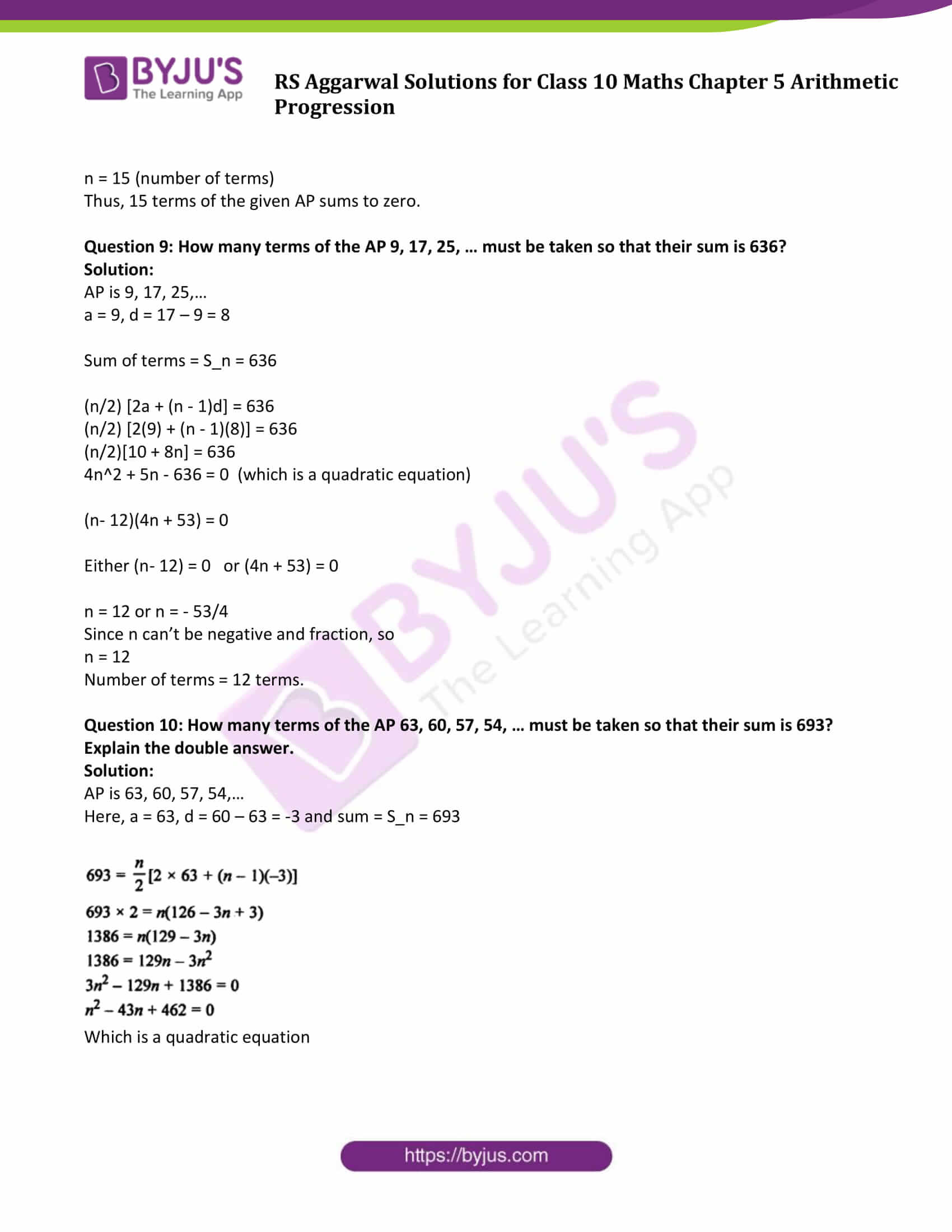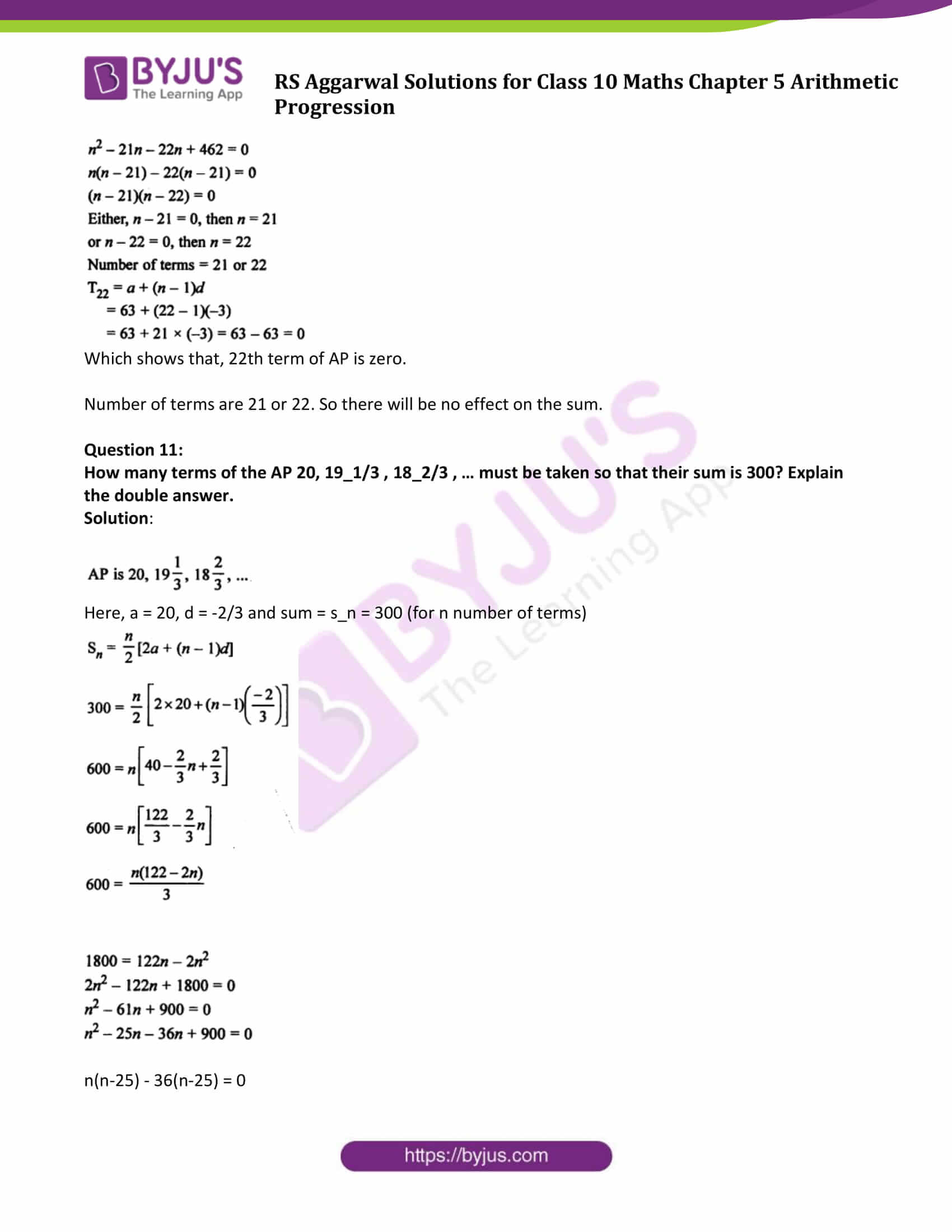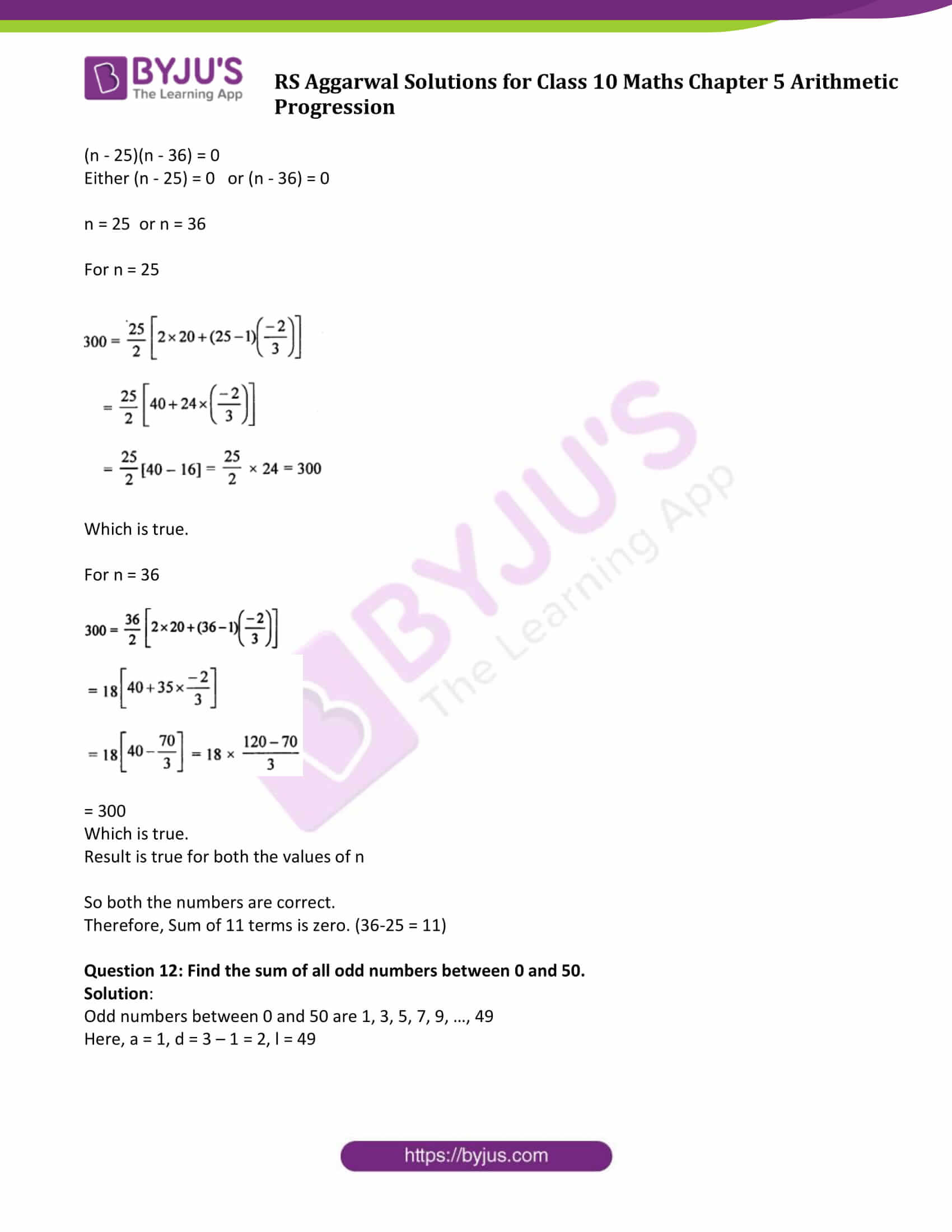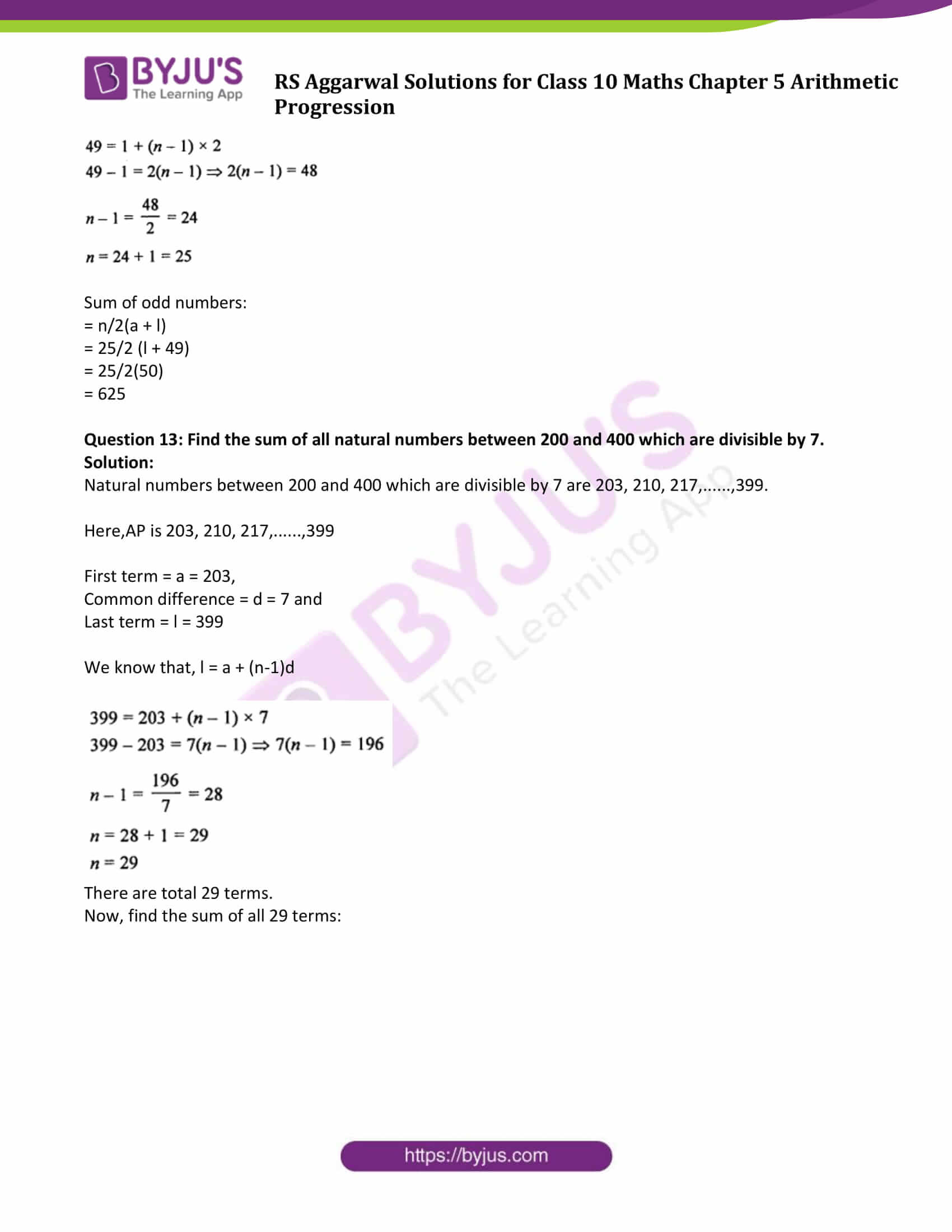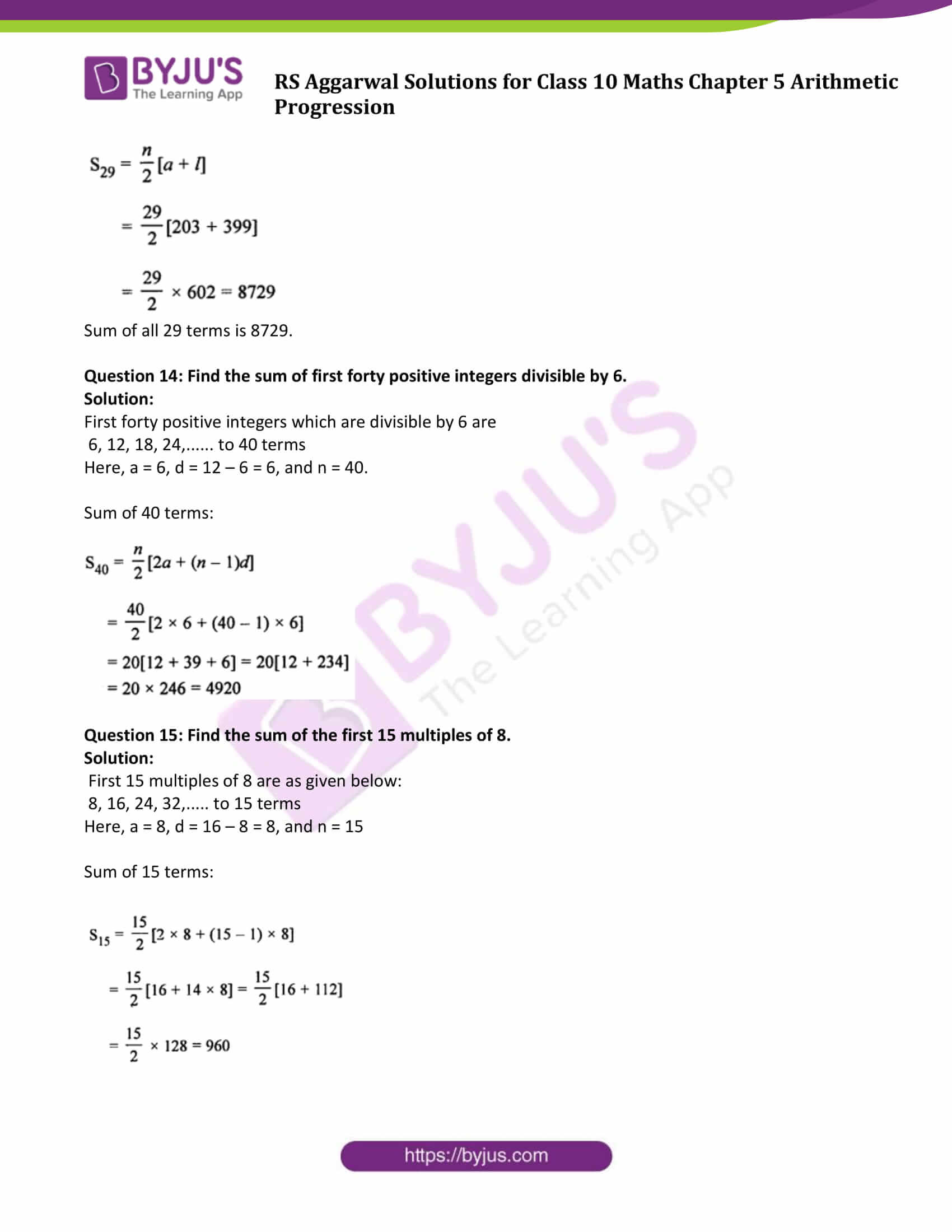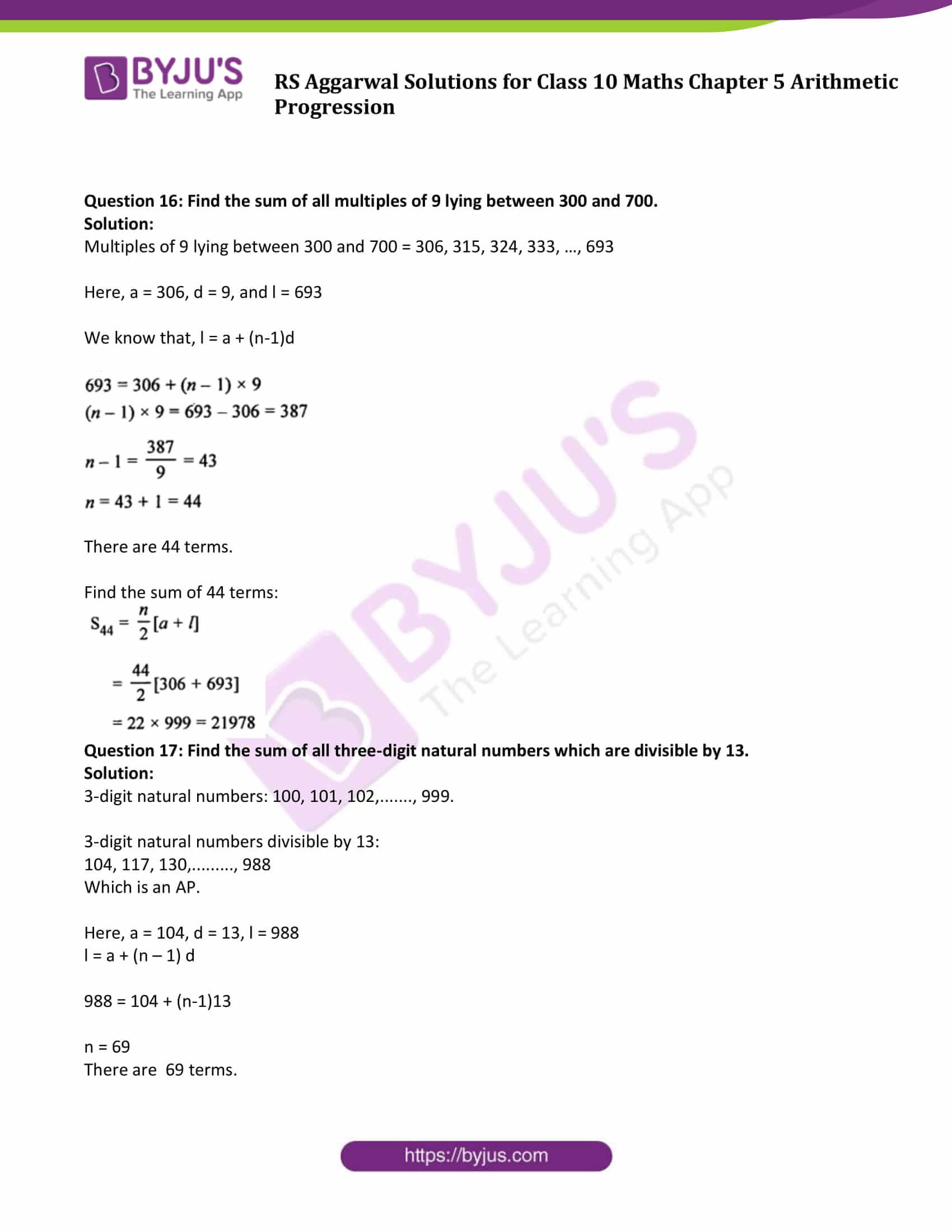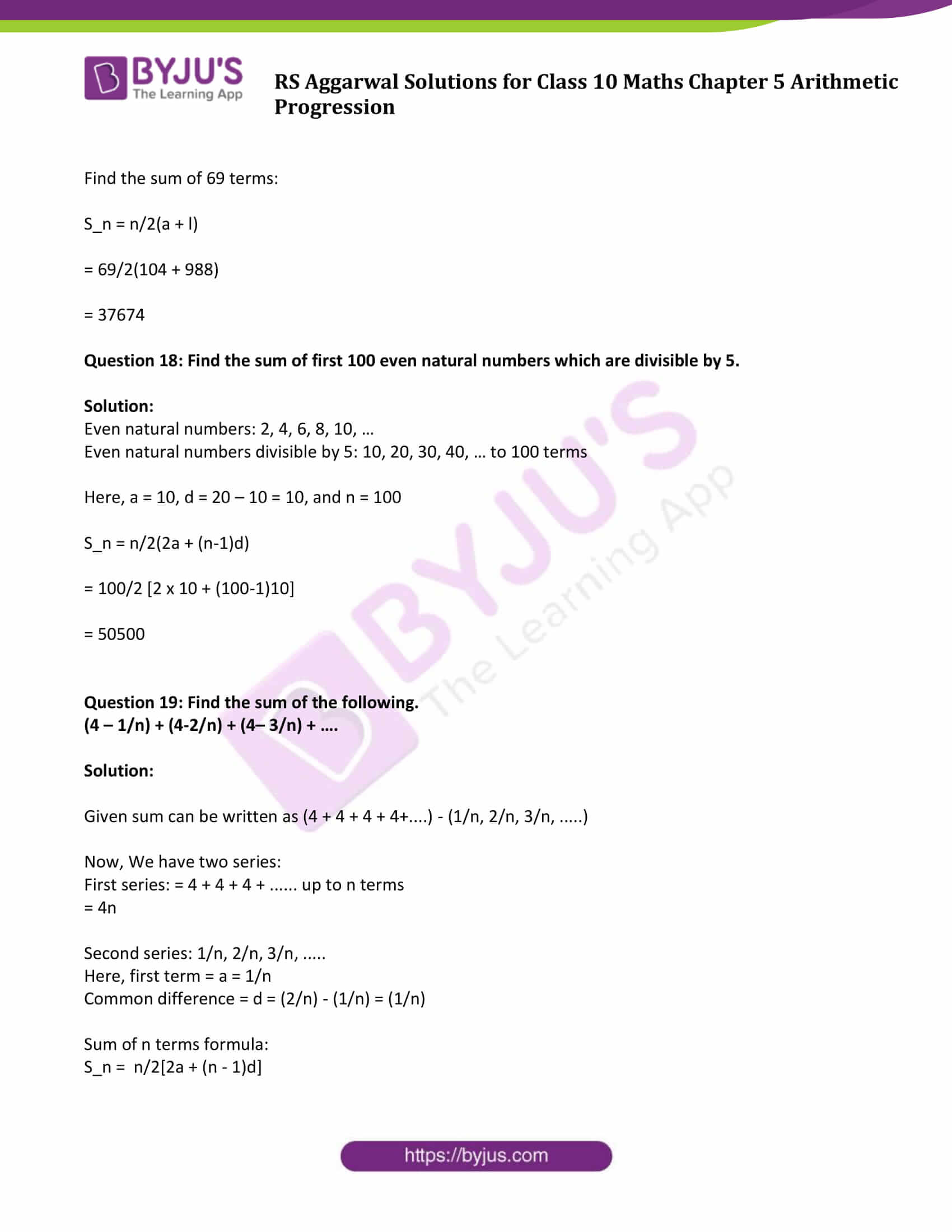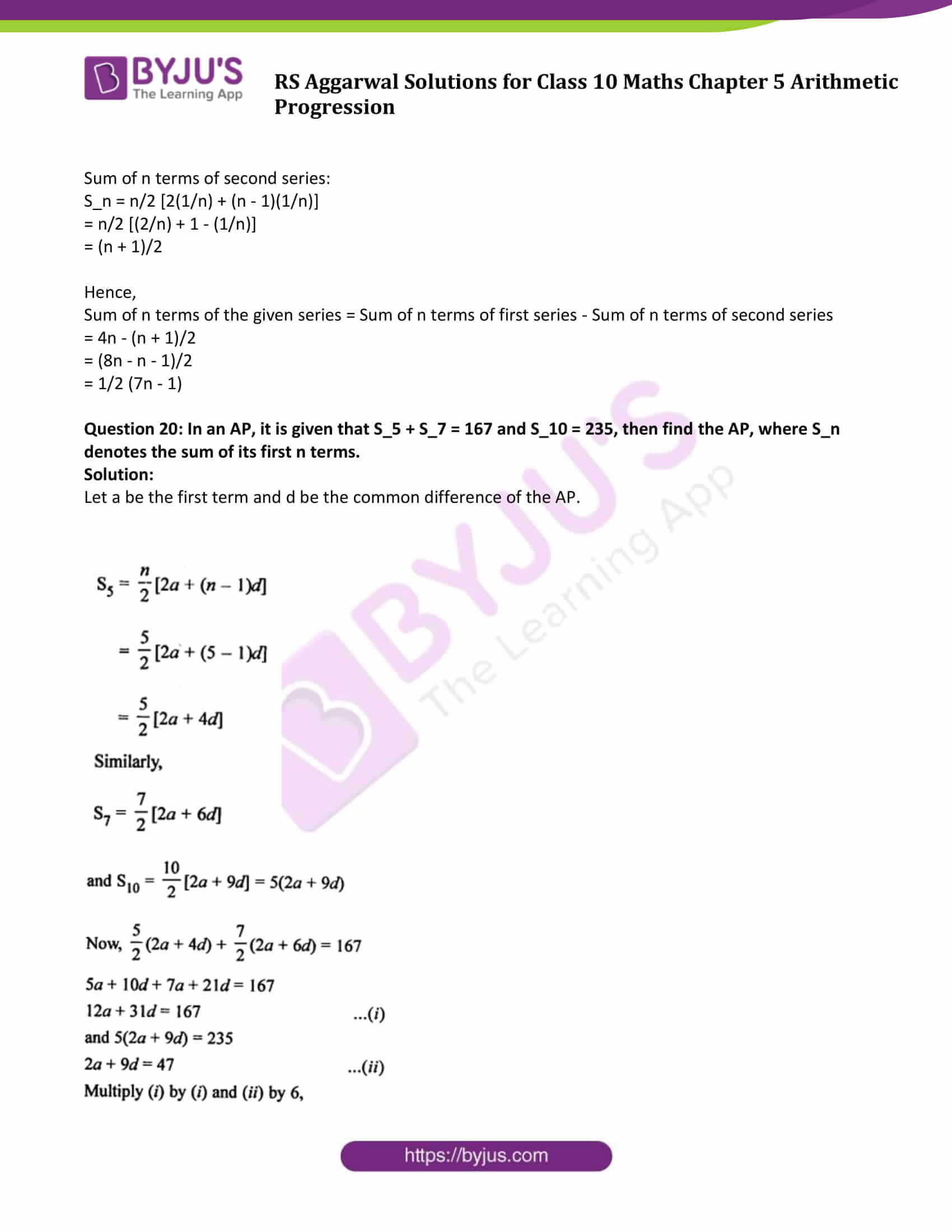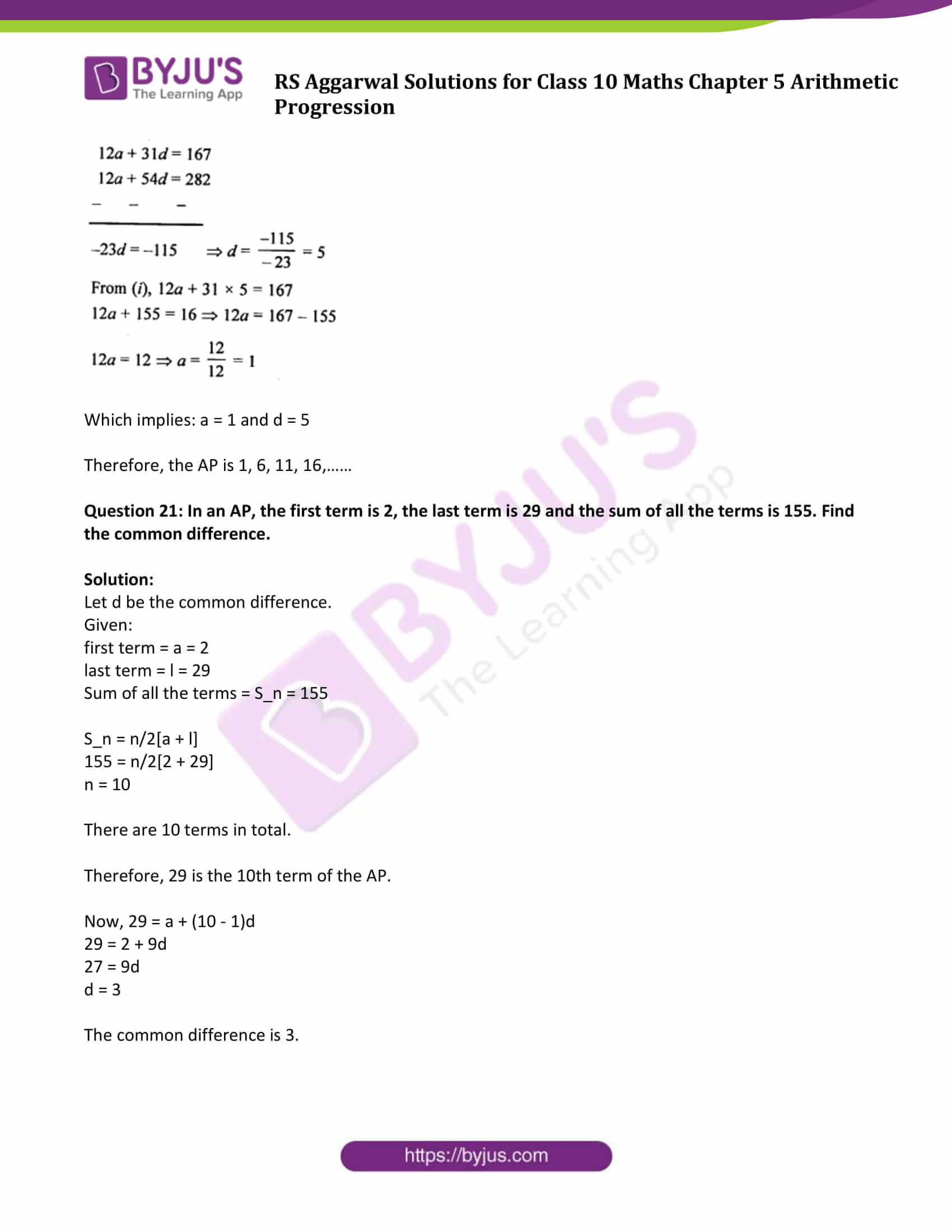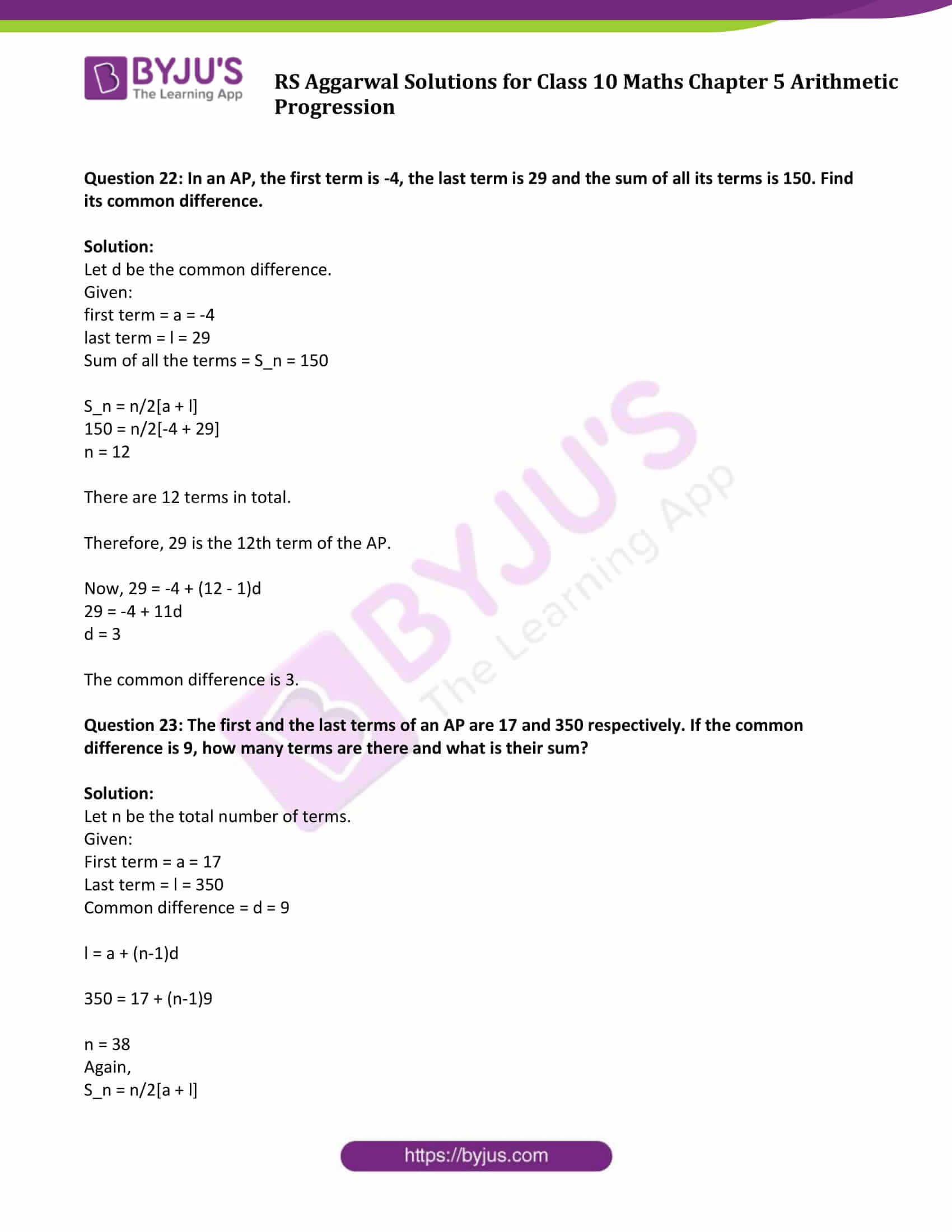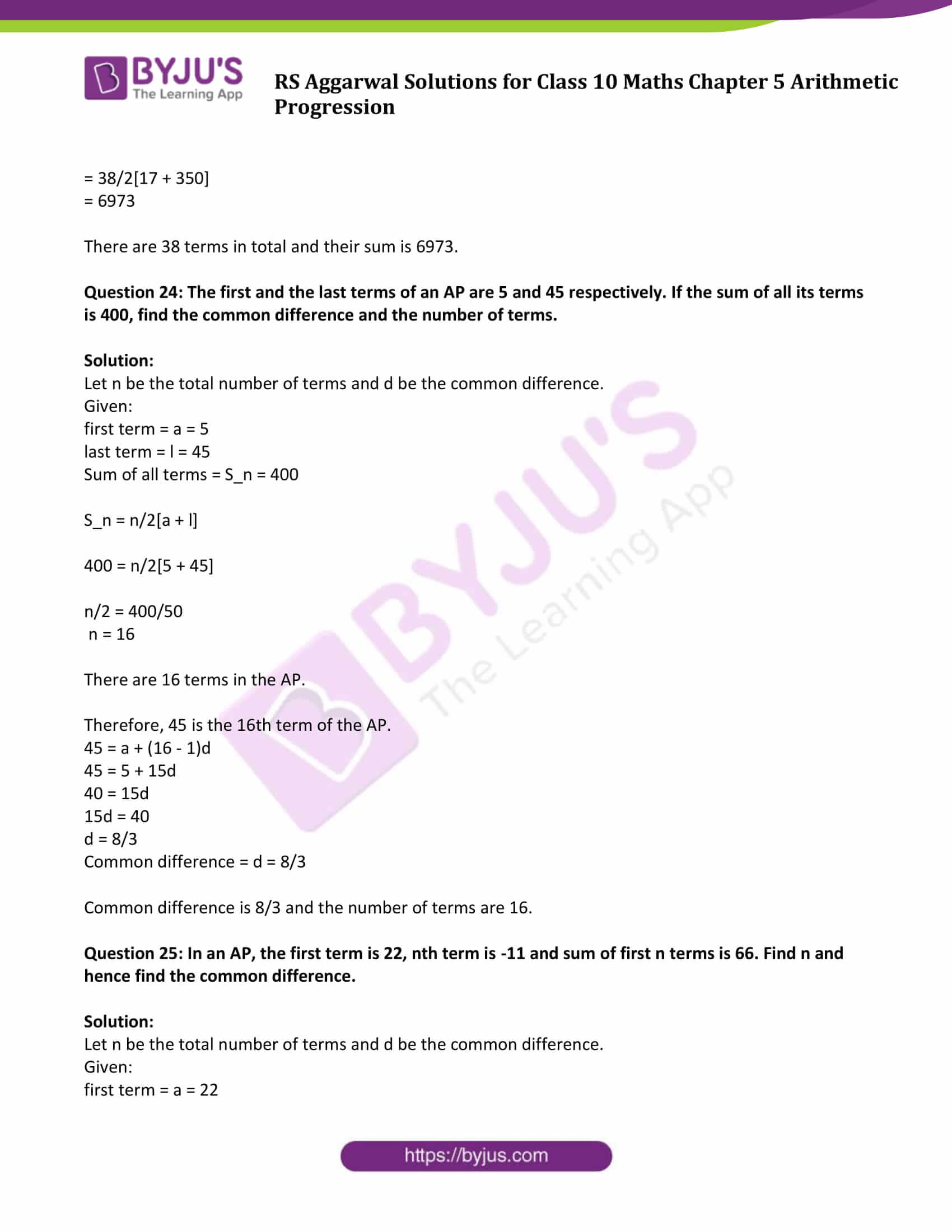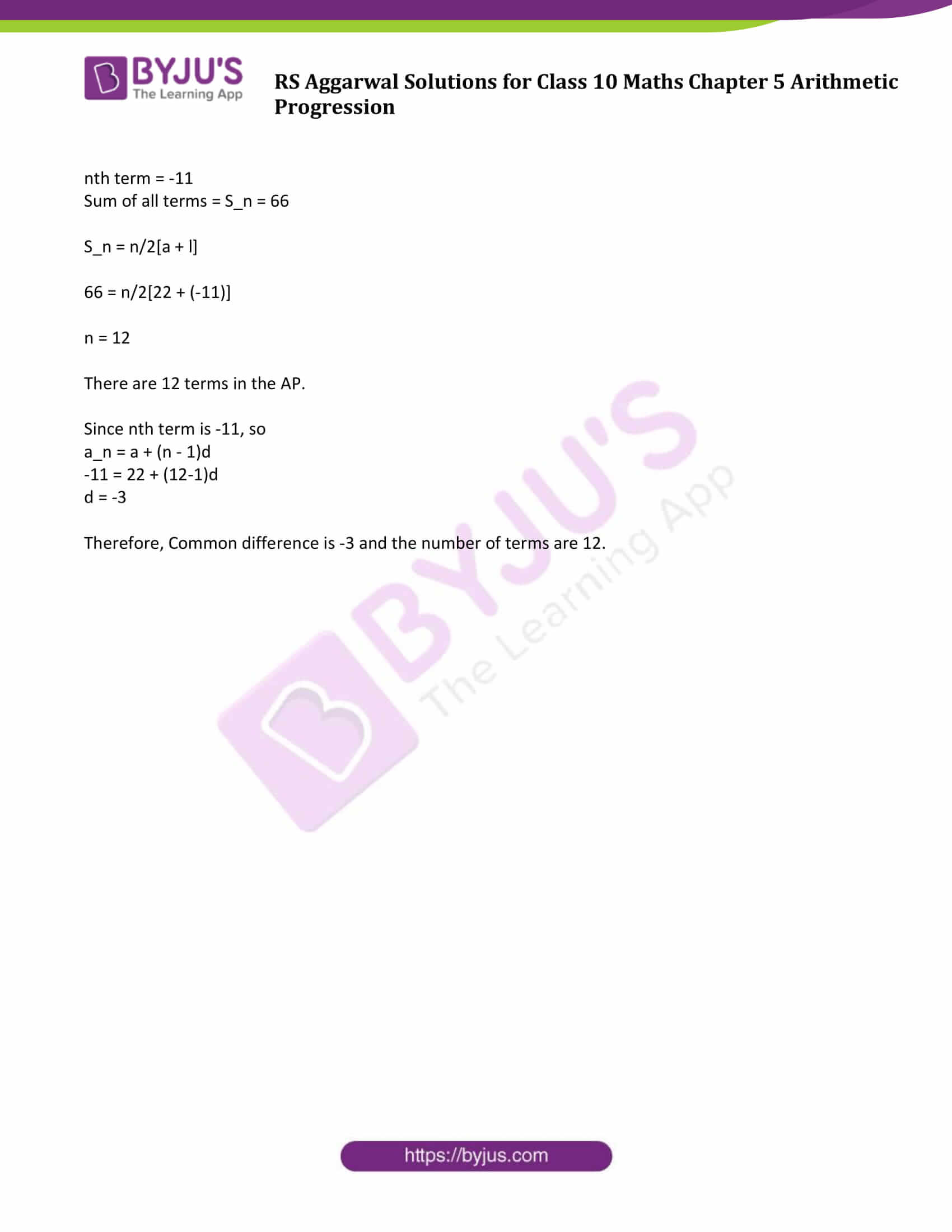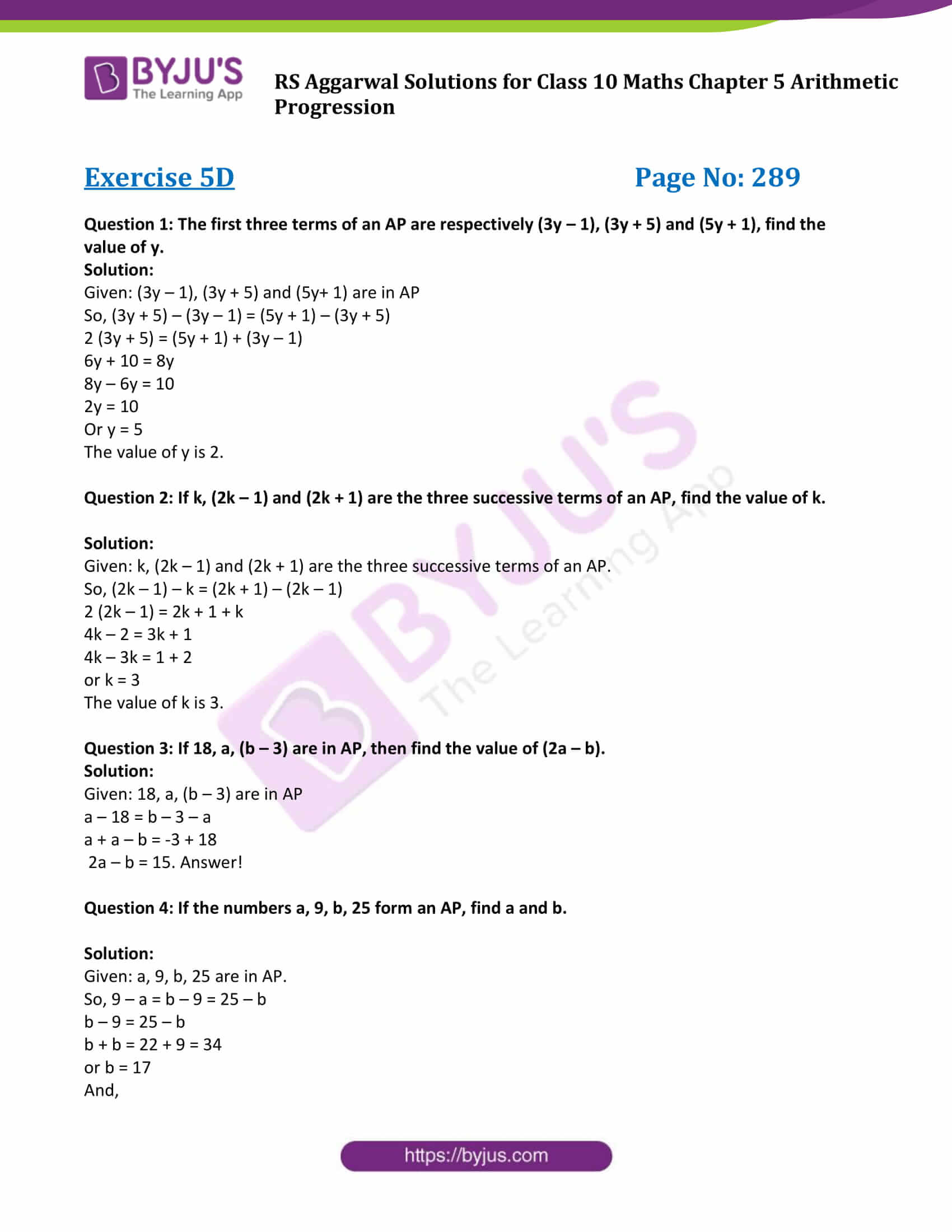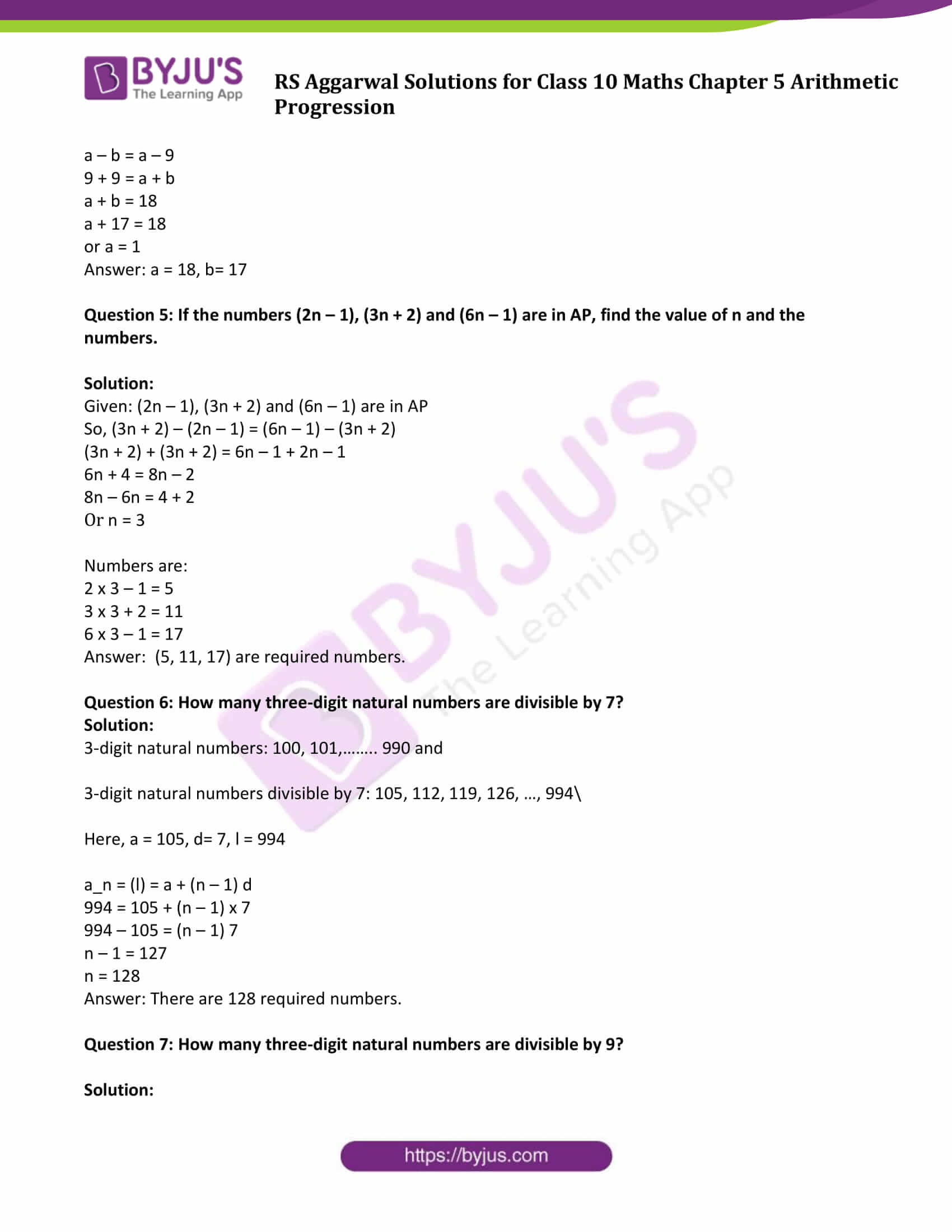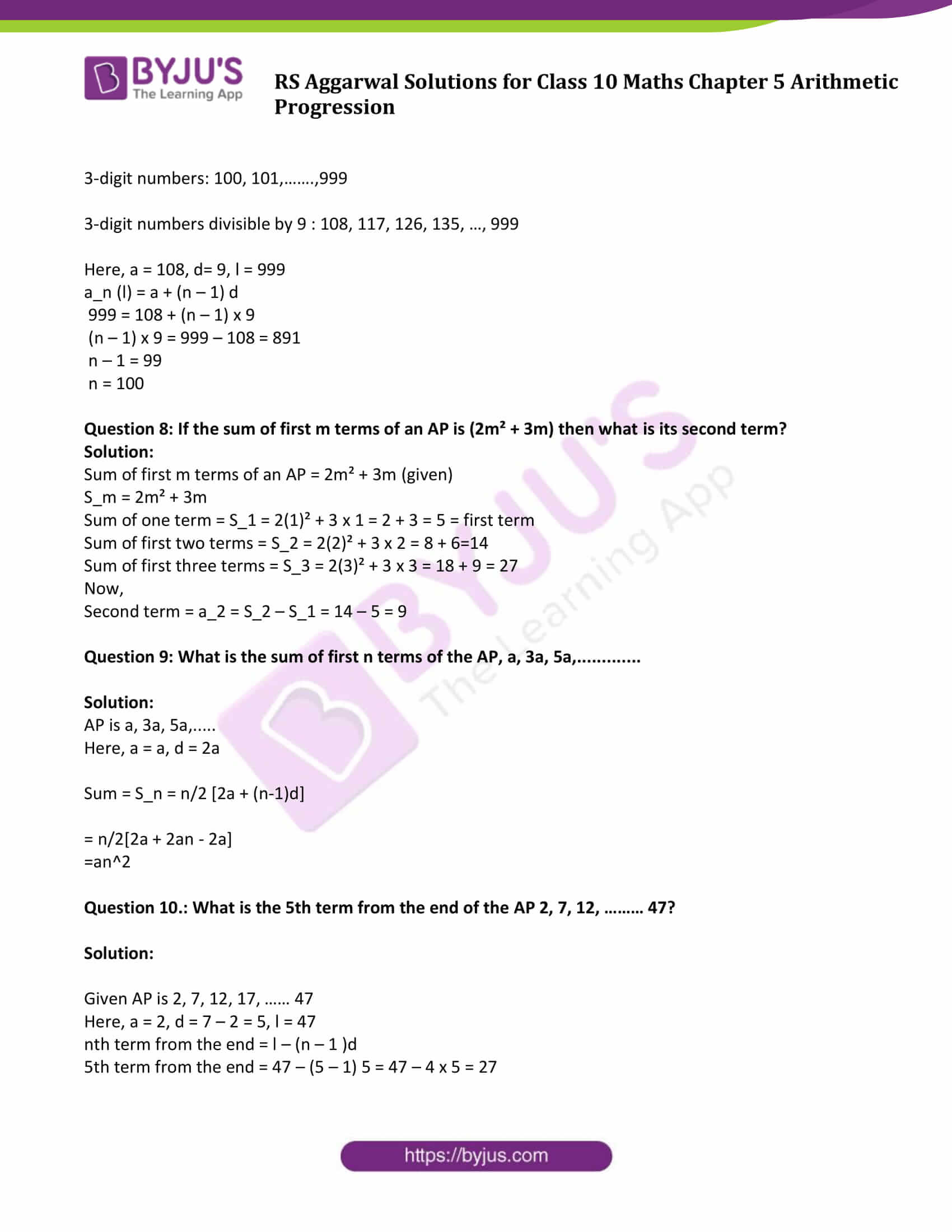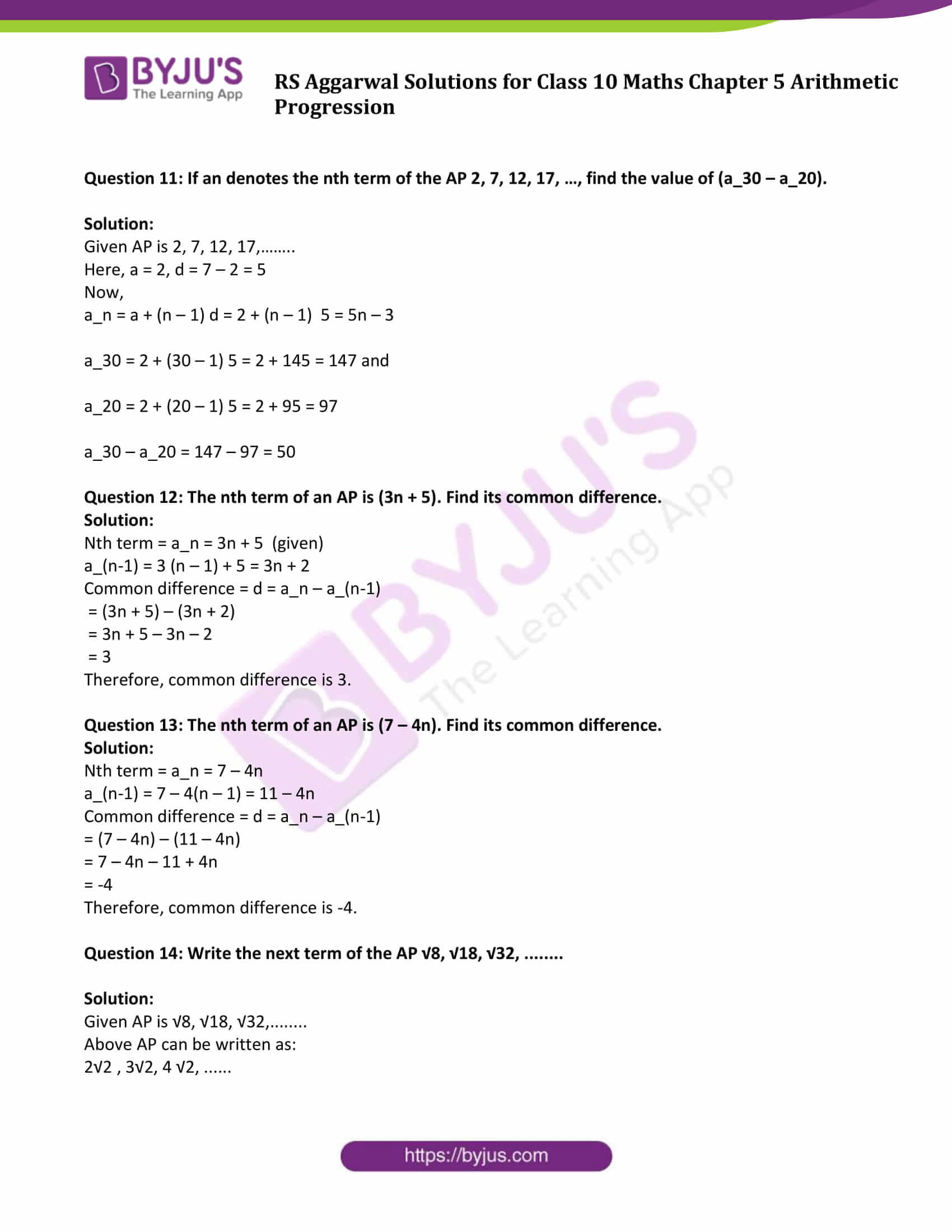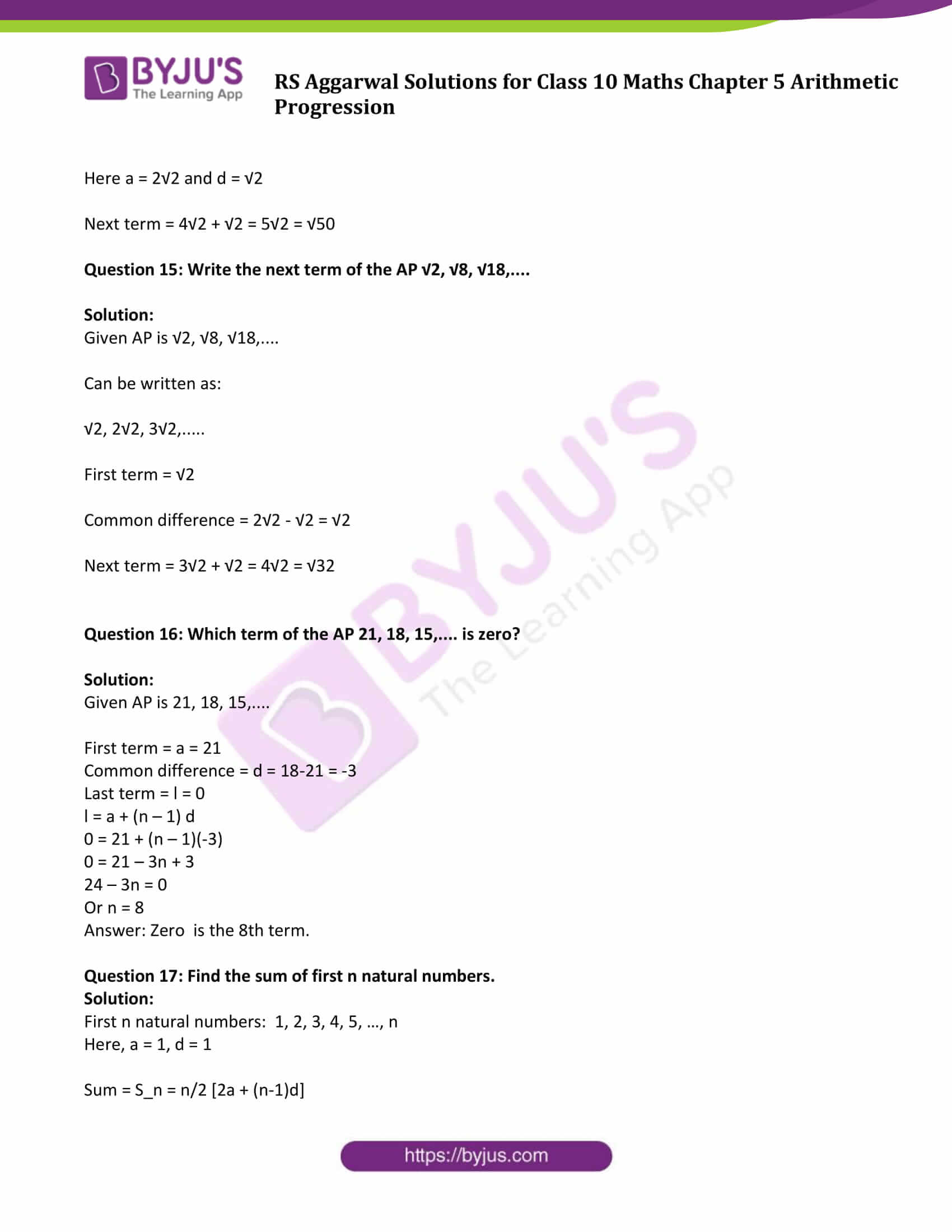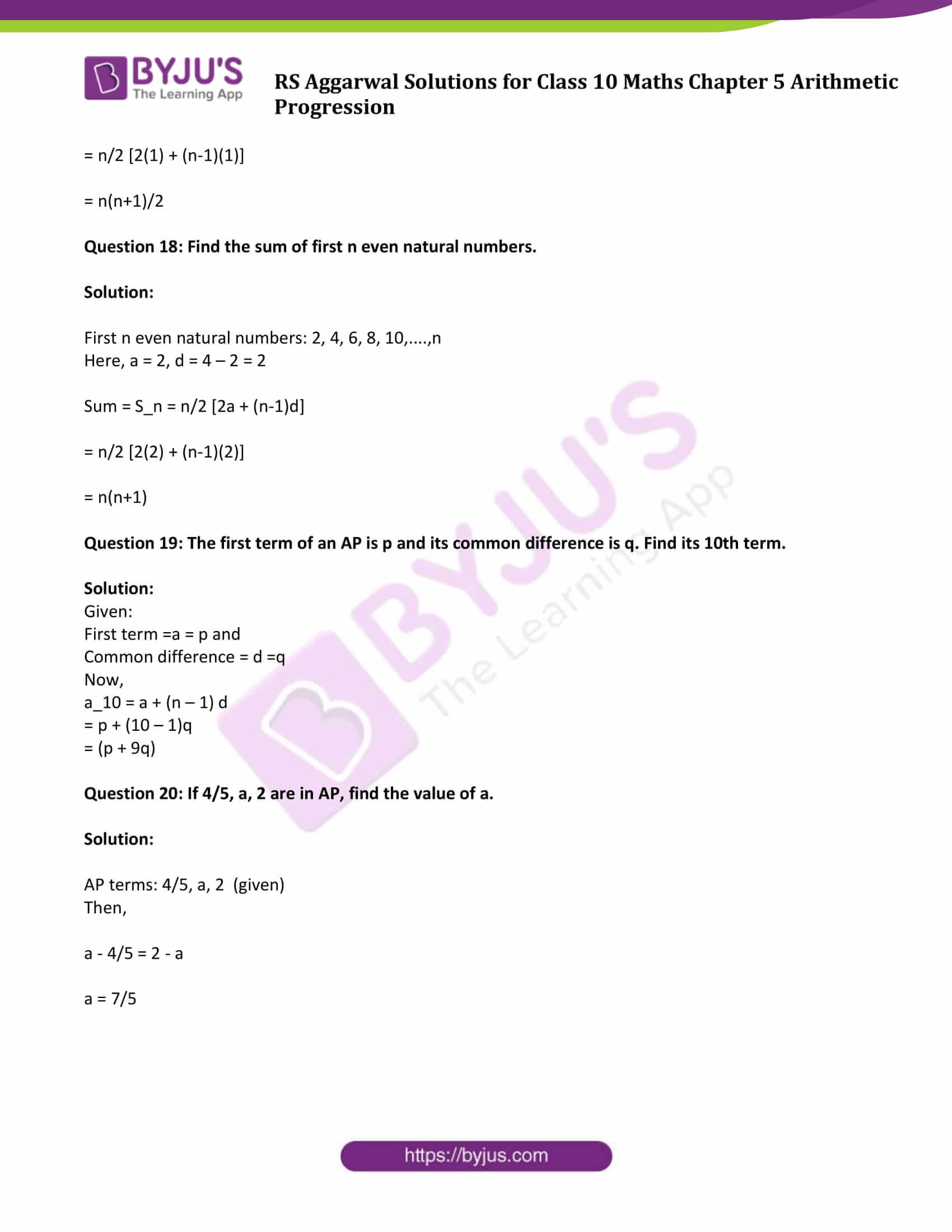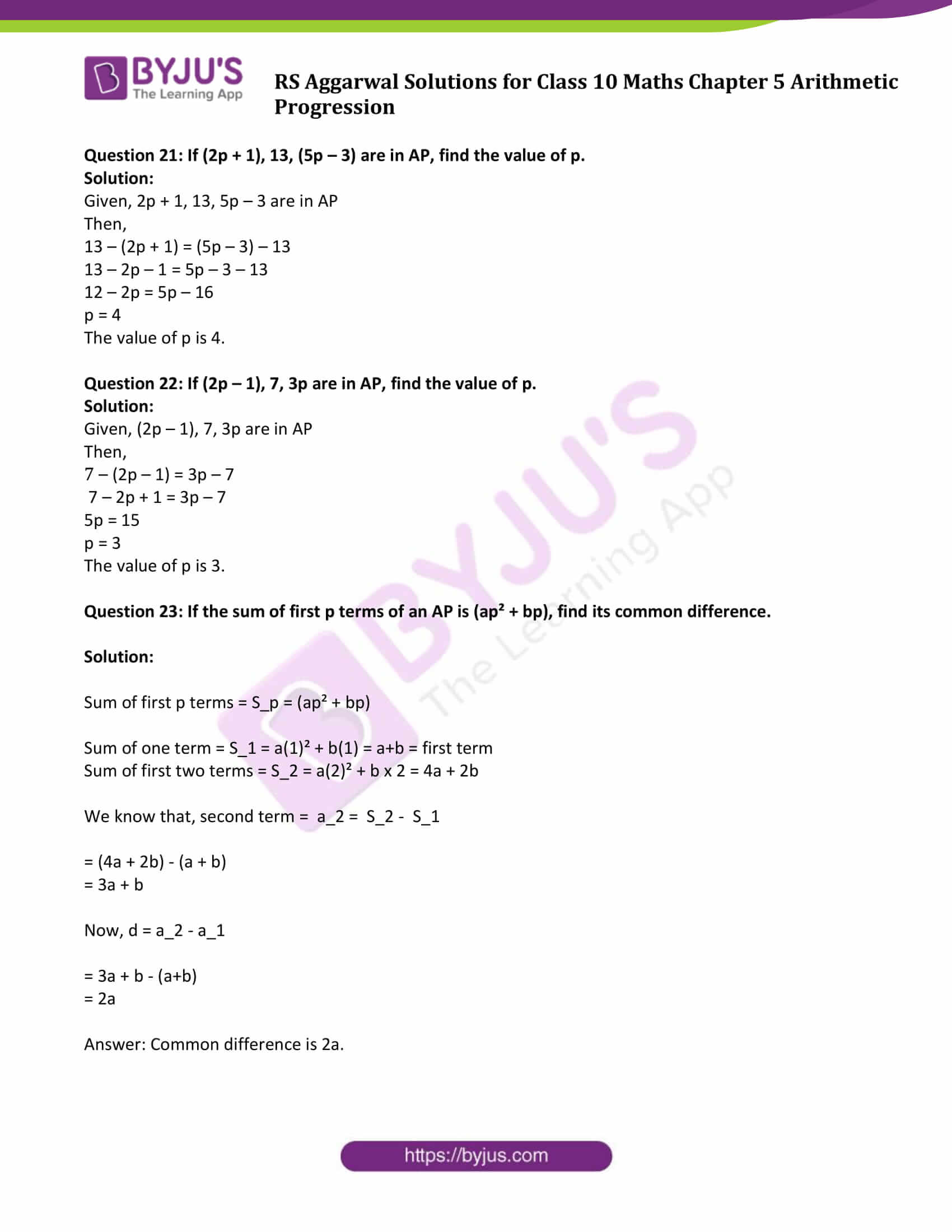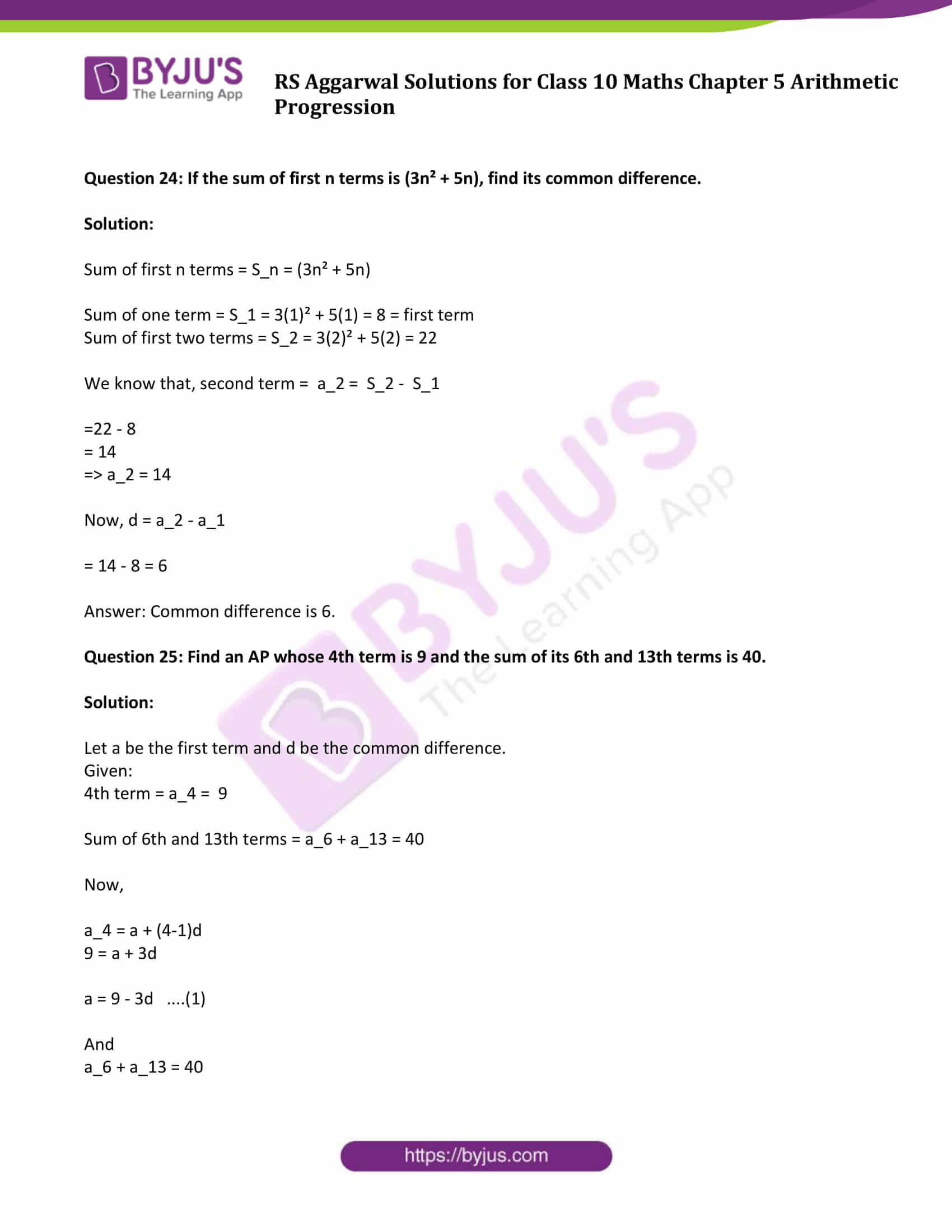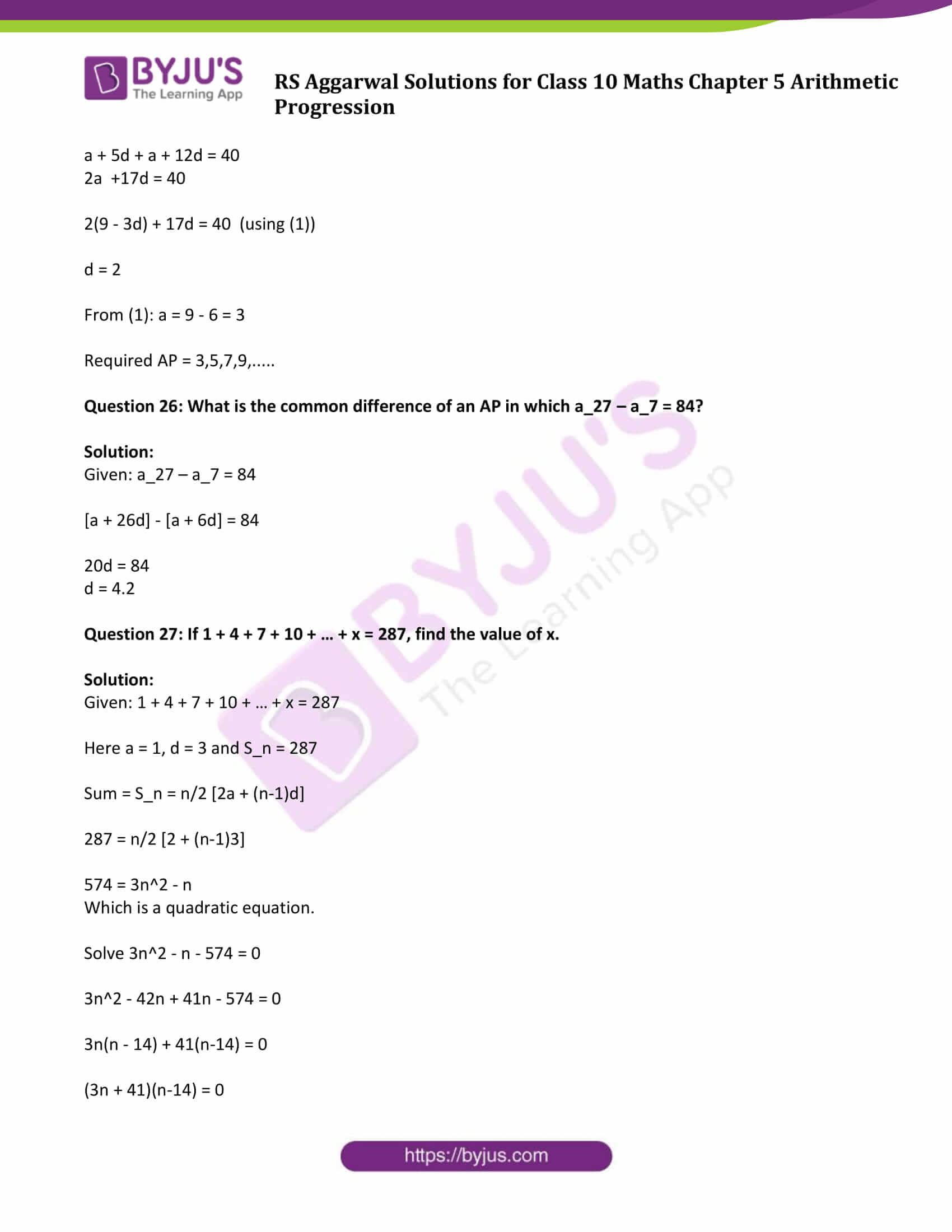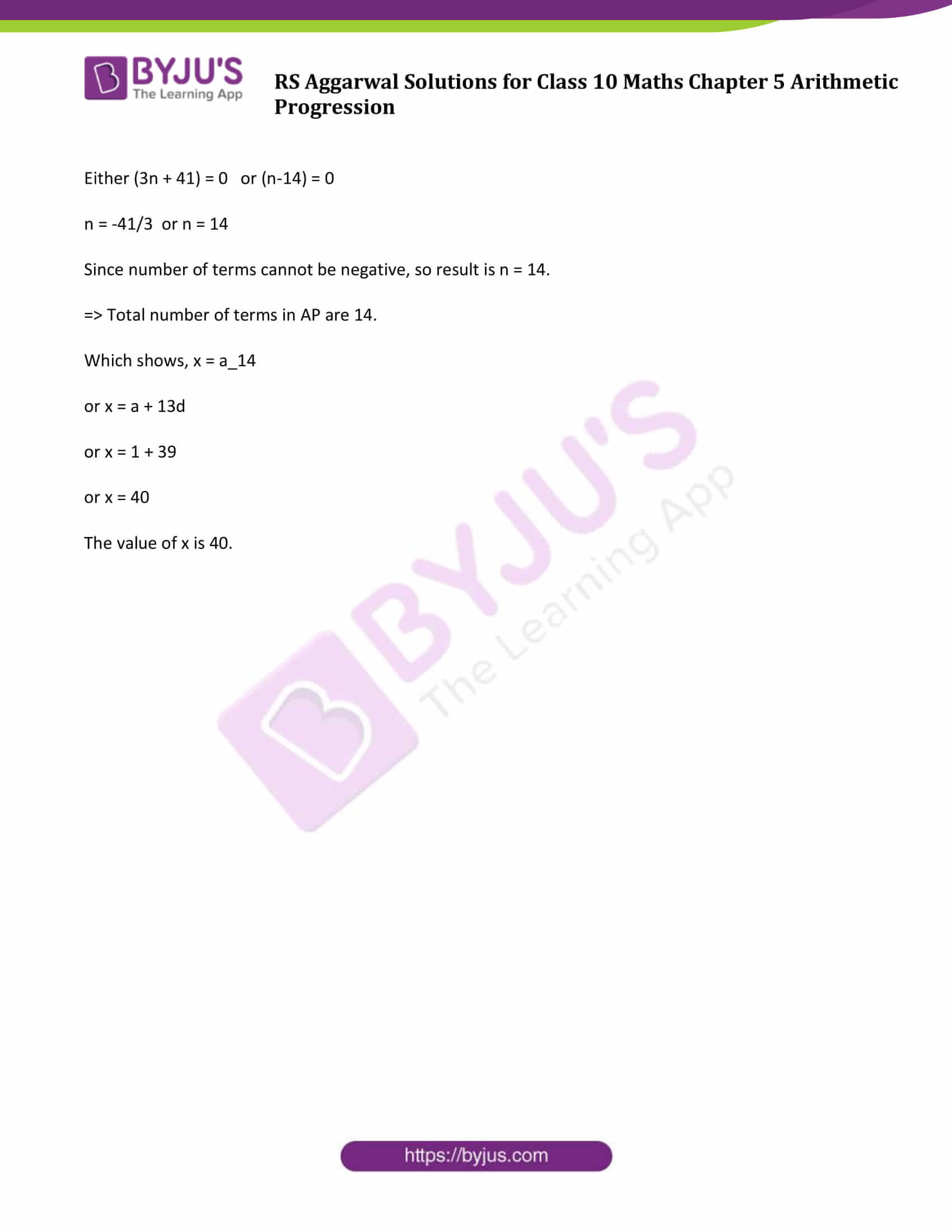### Access Solutions to Maths R S Aggarwal Chapter 5 Arithmetic Progression

Get detailed solutions for all the questions listed under the below exercises:

Exercise 5A Solutions: 30 Questions (Short Answers)

Exercise 5B Solutions: 16 Questions (Short Answers)

Exercise 5C Solutions : 15 Questions (Short Answers)

Exercise 5D Solutions: 20 Questions (Long Answers)

## Exercise 5A Page No: 257

Question 1:

Show that each of the progressions given below is an AP. Find the first term, common difference and next term of each.

(i) 9, 15, 21, 27, …..

(ii) 11, 6, 1, -4, ……

(iii) -1, -5 /6 , -2 /3 , -1 /2 , …………

(iv) √2, √8, √18, √32, ……..

(v) √20, √45, √80, √125, ……

Solution:

(i) 9, 15, 21, 27, …

Here, 15 – 9 = 21 – 15 = 27 – 21 = 6 (which is constant)

Common difference is 6

Or d = 6

Next term = 27 + d = 27 + 6 = 33

(ii) 11, 6, 1, -4, …

Here, 6 – 11 = 1 – 6 = -5 -4 – 1 = -5 (which is constant)

d (common difference) = -5

Next term = -4 – 5 = -9

(iii) -1, -5 /6 , -2 /3 , -1 /2 , …………

-5/6 – (-1) = 1/6 and

-2/3 – (-5/6) = 1/6

d (common difference) = 1/6

Next term = -1/2 + 1/6 = -1/3

(iv) √2, √8, √18, √32, ……..

√8 – √2 = 2√2 – √2 = √2

√32 – √18 = 4√2 – 3√2 = √2

d (common difference) = √2

Next term = √32 + √2 = 4√2 + √2 = 5√2 = √50

(v) √20, √45, √80, √125, ……

√20, √45, √80, √125, ……

√45 – √20 = 3√5 – 2√5 = √5

√125 – √80 = 5√5 – 4√5 = √5

d (common difference) = √5

Next term = √125 + √5 = 5√5 + √5 = 6√5 or √180

Question 2:

Find:

(i) the 20th term of the AP 9, 13, 17, 21, ……

(ii) the 35th term of the AP 20, 17, 14, 11, ……

(iii) the 18th term of the AP √2, √18 , √50, √98, ………

(iv) the 9th term of the AP 3 /4 , 5 /4 , 7 /4 , 9 /4 , …….

(v) the 15th term of the AP -40, -15, 10, 35, ……

Solution:

(i)Given: AP is 9, 13, 17, 21, ……

Here, first term = a = 9

Common difference = d = 13 – 9 = 4

a_n = a + (n-1)d

a20= 9 + (20-1)4

= 85

(ii) the 35th term of the AP 20, 17, 14, 11, ……

Given: AP is 20, 17, 14, 11, ……

Here, first term = a = 20

Common difference = d = 17 – 20 = -3

n = 35

a_n = a + (n-1)d

a_35 = 20 + (35-1)(-3)

= -82

(iii) the 18th term of the AP √2, √18 , √50, √98, ………

Given: AP is √2, √18 , √50, √98, ………

or √2, 3√2 , 5√2, 7√2,….

Here, first term = a = √2

Common difference = d = 3√2 – √2 = 2√2

n = 18

a_n = a + (n-1)d

a_18 = √2 + 34√2

= 35√2

(iv) the 9th term of the AP 3 /4 , 5 /4 , 7 /4 , 9 /4 , …….

Given: AP is 3 /4 , 5 /4 , 7 /4 , 9 /4 , …….

Here, first term = a = 3/4

Common difference = d = 5/4 – 3/4 = 1/2

n = 9

a_n = a + (n-1)d

a_9 = 3/4 + (9 -1)1/2

= 19/4

(v) the 15th term of the AP -40, -15, 10, 35, ……

Given: AP is -40, -15, 10, 35, ……

Here, first term = a = -40

Common difference = d = -15 – (-40) = -15 + 40 = 25

n = 15

a_n = a + (n-1)d

a_15 = -40 + (15 – 1)25

= 310

Question 3:

(i) Find the 37th term of the AP 6, 7_3 /4 , 9_1 /2 , 11_1 /4 , ………..

(ii) Find the 25th term of the AP 5, 4_1 /2 , 4, 3_1 /2 , 3, ……….

Solution: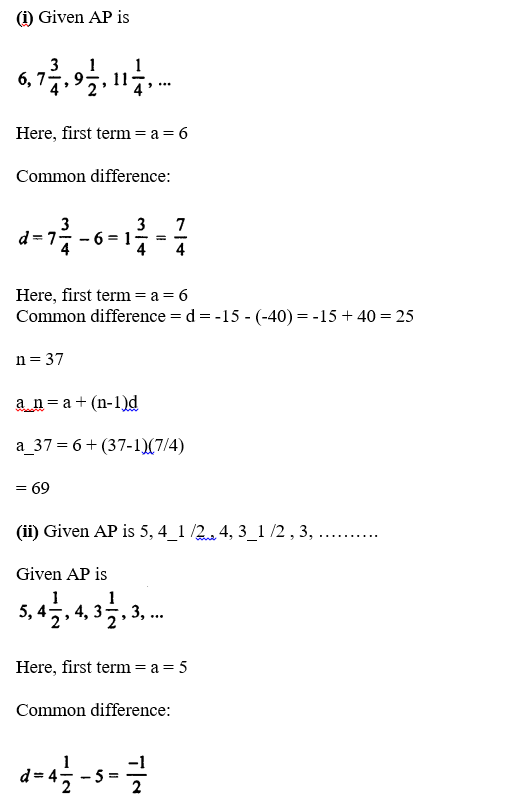n = 25

a_n = a + (n-1)d

a_25 = 5 + (25-1)(-1/2)

= -7

Question 4:

Find the value of p for which the numbers 2p – 1, 3p + 1, 11 are in AP. Hence, find the numbers.

Solution:

If 2p – 1, 3p + 1, 11 are terms in AP, then

a2 – a1 = a3 – a2 …..(1)

From given:

a1 = 2p – 1

a2 = 3p + 1

a3 = 11

From (1), we get

(3p + 1) – (2p – 1) = 11 – (3p + 1)

3p + 1 – 2p + 1 = 11 – 3p – 1

p + 2 = 10 – 3p

4p = 8

p = 2

For p = 2, these terms are in AP.

Question 5:

Find the nth term of each of the following APs:

(i) 5, 11, 17, 23, ……

(ii) 16, 9, 2, -5, ……

Solution:

(i)AP is 5, 11, 17, 23, ……

Here, first term = a = 5

Common difference = d = 11 – 5 = 6

Now,

a_n = a (n – 1)d

= 5 + (n – 1) 6

= 5 + 6n – 6

= (6n – 1)

(ii) AP is 16, 9, 2, -5, ……

Here, first term = a = 16

Common difference = d = 9 – 16 = -7

a_n = a + (n – 1)d

= 16 + (n – 1) (-7)

= 16 – 7n + 7

= (23 – 7n)

Question 6:

If the nth term of a progression is (4n – 10) show that it is an AP. Find its

(i) first term,

(ii) common difference, and

(iii) 16th term.

Solution:

nth term of AP is 4n – 10 (Given)

Putting n = 1, 2, 3, 4, …, we get

At n = 1: 4n – 10 = 4 x 1 – 10 = 4 – 10 = -6

At n = 2: 4n – 10 = 4 x 2 – 10 = 8 – 10 = -2

At n = 3: 4n – 10 = 4 x 3 – 10 = 12 – 10 = 2

At n = 1: 4n – 10 = 4 x 4 – 10 = 16 – 10 = 6

We see that -6, -2, 2, 6,… are in AP

(i) first term = -6

(ii) Common difference = -2 – (-6) = 4

(iii) 16th term:

Using formula: a_n = a + (n – 1)d

Here n = 16

a_16 = -6 + (16 – 1)4 = 54

Question 7:

How many terms are there in the AP 6, 10, 14, 18, …. 174?

Solution:

Given: AP is 6, 10, 14, 18,…, 174

Here, first term = a = 6

Common difference = d= 10 – 6 = 4

To find: the number of terms (n)

Last term = a + (n – 1)d

174 = 6 + (n – 1) 4

174 – 6 = (n – 1) 4

n – 1 = 168 /4 = 42

n = 42 + 1 = 43

There are 43 terms.

Question 8:

How many terms are there in the AP 41, 38, 35, …, 8?

Solution:

Given: AP is 41, 38, 35,…, 8

Here, first term = a = 41

Last term = 8

Common difference = d = 38 – 41 = -3

To find: the number of terms (n)

Last term = a + (n – 1)d

8 = 41 + (n – 1)(-3)

8 – 41 = (n – 1)(-3)

n – 1 = 11

n = 11 + 1 = 12

There are 12 terms.

Question 9:

How many terms are there in the AP is 18, 15_1/2 , 13, …, -47 ?

Solution:

Given: AP is 18, 31/2 , 13, …, -47

Here, first term = a = 18

Last term = -47

Common difference = d = -5/2

To find: the number of terms (n)

Last term = a + (n – 1)d

-47 = 18 + (n – 1)(-5/2)

-47 – 18 = (n – 1)(-5/2)

n = 27

There are 27 terms.

Question 10:

Which term of the AP 3, 8, 13, 18, … is 88?

Solution:

Let nth term is 88.

AP is 3, 8, 13, 18, …

Here,

First term = a = 3

Common difference = d = 8 – 3 = 5

nth term of AP is a_n = a + (n – 1) d

Now,

88 = 3 + (n – 1)(5)

88 – 3 = (n – 1) x 5

n – 1 = 88 /5

or n = 17 + 1 = 18

Therefore: 88 is the 18th term.

Question 11:

Which term of the AP 72, 68, 64, 60, ….. is 0?

Solution:

AP is 72, 68, 64, 60, …..

Let nth term is 0.

Here,

First term = a = 72

Common difference = d = 68 – 72 = -4

a_n = a + (n – 1)d

0 = 72 + (n – 1)(-4)

-72 = -4(n – 1)

n – 1 = 18

n = 18 + 1 = 19

Therefore: 0 is the 19th term.

Question 12:

Which term of the AP 5 /6 , 1, 1 1 /6 , 1 1 /3 , ….. is 3?

Solution: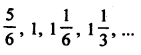Here,

First term = a = 5/6

Common difference = d = 1 – 5/6 = 1/6

Now: a_n = a + (n – 1)d

3 = 5/6 + (n – 1)1/6

Let nth term is 3

Now, a_n = a + (n-1)d

3 = 5/6 + (n-1)1/6

n -1 = 13

n = 13 + 1 = 14

Therefore, 3 is the 14th term.

Question 13:

Which term of the AP 21, 18, 15,… is -81?

Solution:

AP is 21, 18, 15, ……

Let nth term -81

Here, a = 21, d = 18 – 21 = -3

a_n = a + (n – 1)d

-81 = 21 + (n – 1)(-3)

-81 – 21 = (n – 1)(-3)

-102 = (n – 1)(-3)

n = 34 + 1 = 35

Therefore, -81 is the 35th term

Question 14:

Which term of the AP 3, 8, 13, 18, …will be 55 more than its 20th term?

Solution:

Given AP is 3, 8, 13, 18,…

First term = a = 3

Common difference = d = 8 – 3 = 5

And n = 20 and a_20 be the 20th term, then

a_20 = a + (n – 1)d

= 3 + (20 – 1) 5

= 3 + 95

= 98

The required term = 98 + 55 = 153

Now, 153 be the nth term, then

a_n = a + (n – 1)d

153 = 3 + (n – 1) x 5

153 – 3 = 5(n – 1)

150 = 5(n – 1)

n – 1 = 30

n = 31

Required term will be 31st term.

Question 15:

Which term of the AP 5, 15, 25,… will be 130 more than its 31st term?

Solution:

AP is 5, 15, 25,…

First term = a = 5

Common difference = d = 15 – 5 = 10

Find 31st term:

a_31 = a + (n – 1)d

= 5 + (31 – 1) 10

= 5 + 30 x 10

= 305

Required term = 305 + 130 = 435

Now, say 435 be the nth term, then

a_n = a + (n – 1)d

435 = 5 + (n – 1)10

435 – 5 = (n – 1)10

n – 1 = 43

n = 44

The required term will be 44th term.

Question 16:

If the 10th term of an AP is 52 and 17th term is 20 more than its 13 th term, find the AP

Solution:

Let a be the first term and d be the common difference, then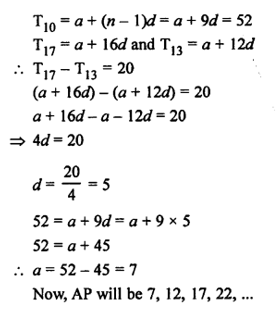Question 17:

Find the middle term of the AP 6, 13, 20,…, 216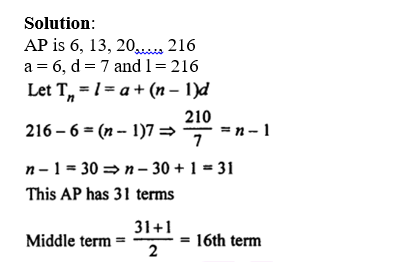a_16 = 6 + (16 – 1)7 = 6 + 105 = 111

Therefore, midterm of the AP id 111.

Question 18:

Find the middle term of the AP 10, 7, 4, ………. (-62)

Solution:

AP is 10, 7, 4, …..…, (-62)

a = 10,

d = 7 – 10 = -3, and

l = -62

Now, a_n = l = a + (n – 1)d

-62 = 10 + (n – 1) x (-3)

-62 – 10 = -3(n- 1)

-72 = -3(n – 1)

Or n = 24 + 1 = 25

Middle term = (25 + 1) /2 th = 13th term

Find the 13th term using formula, we get

a_13 = 10 + (13 – 1)(-3) = 10 – 36 = -26

Question 19:

Find the sum of two middle most terms of the AP -4 /3 , -1, -2 /3 , …, 4_ 1 /3 .

Solution:

Given AP is -4 /3 , -1, -2 /3 , …, 13 /3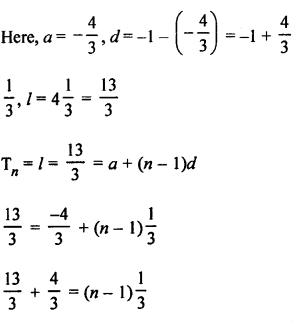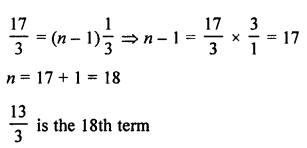Middle terms will be: (18/2)th + (18/2 + 1)th

= 9th + 10th term

Now,

a_9 + a_10 = a + 8d + a + 9d

= 2a + 17d

= 2(-4/3) + 17(1/3)

= 3

Question 20:

Find the 8th term from the end of the AP 7, 10, 13, ……, 184.

Solution:

Given: AP is 7, 10, 13,…, 184

a = 7, d = 10 – 7 = 3 and l = 184

nth term from the end = l – (n – 1)d

Now,

8th term from the end be

184 – (8 – 1)3 = 184 – 21 = 163

Question 21:

Find the 6th term from the end of the AP 17, 14, 11, …, (-40).

Solution:

Given: AP is 17, 14, 11, …,(-40)

a = 17, d = 14 – 17 = -3, l = -40

6th term from the end = l – (n – 1)d

= -40 – (6 – 1) (-3)

= -40 – (5 x (-3))

= -40 + 15

= -25

Question 22:

Is 184 a term of the AP 3, 7, 11, 15, …..?

Solution:

Given AP is 3, 7, 11, 15, …

a = 3, d = 7 – 3 = 4

Let 184 be the nth term of the AP

a_n = a + (n – 1)d

184 = 3 + (n – 1) x 4

184 – 3 = (n – 1) x 4

181 /4 = n – 1

n = 181 /4 + 1 = 185/4 (Which is in fraction)

Therefore, 184 is not a term of the given AP.

Question 23:

Is -150 a term of the AP 11, 8, 5, 2,…?

Solution:

Given AP is AP 11, 8, 5, 2,…

Here a = 11, d = 8-11= -3

Let -150 be the nth term of the AP

a_n = a + (n – 1)d

-150 = 11 +(n-1)(-3)

or n = 164/3

Which is a fraction.

Therefore, -150 is not a term of the given AP.

Question 24:

Which term of the AP 121, 117, 113,… is its first negative term?

Solution:

Let nth of the AP 121, 117, 113,… is negative. Let T_n be the nth term then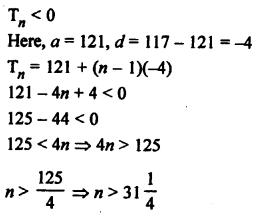Therefore, 32nd term will be the 1st negative term.

Question 25:

Which term of the AP 20, 19_1 /4 , 18_1 /2 , 17_3 /4 , …………. is its first negative term?

Solution: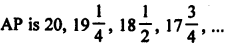a = 20, d = -3/4

Let nth term be the 1st negative term of the AP

a_n < 0

a_n = a + (n – 1)d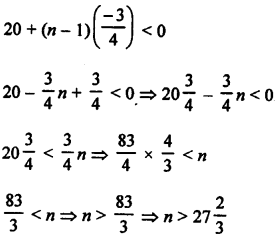Therefore, 28th term will be the 1st negative term.

Question 26:

The 7th term of an AP is -4 and its 13th term is -16. Find the AP.

Solution:

Let us say a be the first term and d be the common difference of an AP

a_n = a + (n – 1)d

a_7 = a + (7 – 1)d

= a + 6d = -4 …………(1)

And a_13 = a + 12d = -16 …..…..(2)

Subtracting equation (1) from (2), we get

6d = -16 – (-4) = -12

From (1), a + 6d = -4

a + (-12) = -4

a = -4 + 12 = 8

a = 8, d = -2

AP will be 8, 6, 4, 2, 0, ……

Question 27:

The 4th term of an AP is zero, Prove that its 25th term is triple its 11th term.

Solution:

Let a be the first term and d be the common difference of an AP.

a_4 = a + (n- 1)d

= a + (4 – 1)d

= a + 3d

Since 4th term of an AP is zero.

a + 3d = 0

or a = -3d ….(1)

Similarly,

a_25 = a + 24d = -3d + 24d = 21d …(2)

a_11 = a + 10d = -3d + 10d = 7d ….(3)

From (2) and (3), we have

a_25 = 3 x a_11

Hence proved.

Question 28:

If the sixth term of an AP is zero then show that its 33rd term is three times its 15th term.

Solution:

Sixth term of an AP is zero

that is a_6 = 0

a + 5d = 0

a = -5 d

Now, a_15 = a + (n – 1 )d

a + (15 – 1)d = -5d + 14d = 9d

and a_33 = a + (n – 1 )d = a + (33 – 1)d = -5d + 32d = 27d

Now, a_33 : a_12

27d : 9d

3 : 1

Which shows that a_33 = 3(a_15)

Hence proved.

Question 29:

The 4th term of an AP is 11. The sum of the 5th and 7th terms of this AP is 34. Find its common difference.

Solution:

Let a be the first term and d be the common difference of an AP.

a_n = a + (n – 1)d

a_4 = a + (4 – 1)d = a + 3d

a + 3d = 11 ………(1)

Now, a_5 = a + 4d and a_7 = a + 6d

Now, a5 + a7 = a + 4d + a + 6d = 2a + 10d

2a + 10d = 34

a + 5d = 17 ……..(2)

Subtracting (1) from (2), we get

2d = 17 – 11 = 6

d = 3

The common difference = 3

Question 30:

The 9th term of an AP is -32 and the sum of its 11th and 13th terms is -94. Find the common difference of the AP.

Solution:

Let a be the first term and d be the common difference of an AP.

nth term = a_n = a + (n – 1)d

Given: 9th term of an AP is -32 and the sum of its 11th and 13th terms is -94

Now,

a_9 = a + 8d = -32 …(1)

a_11 = a + 10d

a_13 = a + 12d

Sum of 11th and 13th terms:

a_11 + a_13 = a + 10d + a + 12d

-94 = 2a + 22d

or a + 11d =-47 …(2)

Subtracting (1) from (2) , we have

3d = -47 + 32 = -15

or d = -5

Common difference is -5.

## Exercise 5B Page No: 264

Question 1:

Determine k so that (3k – 2), (4k – 6) and (k + 2) are three consecutive terms of an AP.

Solution:

Given: (3k – 2), (4k – 6) and (k + 2) are three consecutive terms of an AP.

So, (4k – 6) – (3k – 2) = (k + 2) – (4k – 6)

2(4k – 6) = (k + 2) + (3k – 2)

8k – 12 = 4k + 0

8k – 4k = 0 + 12

or k = 3

Question 2:

Find the value of x for which the numbers (5x + 2), (4x – 1) and (x + 2) are in AP.

Solution:

Given: (5x + 2), (4x – 1) and (x + 2) are terms in AP.

So, d = (4x – 1) – (5x + 2) = (x + 2) – (4x – 1)

2(4x – 1) = (x + 2) + (5x + 2)

8x – 2 = 6x + 2 + 2

8x – 2 = 6x + 4

8x – 6x = 4 + 2

or x = 3

The value of x is 3.

Question 3:

If (3y – 1), (3y + 5) and (5y + 1) are three consecutive terms of an AP then find the value of y.

Solution:

Given: (3y – 1), (3y + 5) and (5y + 1) are 3 consecutive terms of an AP.

So, (3y + 5) – (3y – 1) – (5y + 1) – (3y + 5)

2(3y + 5) = 5y + 1 + 3y – 1

6y + 10 = 8y

8y – 6y = 10

2y = 10

Or y = 5

The value of y is 5.

Question 4:

Find the value of x for which (x + 2), 2x, (2x + 3) are three consecutive terms of an AP.

Solution:

Given: (x + 2), 2x, (2x + 3) are three consecutive terms of an AP.

So, 2x – (x + 2) = (2x + 3) – 2x

2x – x – 2 = 2x + 3 – 2x

x – 2 = 3

x = 2 + 3 = 5

The value of x is 5.

Question 5:

Show that (a – b)², (a² + b²) and (a + b)² are in AP.

Solution:

Assume that (a – b)², (a² + b²) and (a + b)² are in AP.

So, (a² + b²) – (a – b)² = (a + b)² – (a² + b²)

(a² + b²) – (a² + b² – 2ab) = a² + b² + 2ab – a² – b²

2ab = 2ab

Which is true.

Hence given terms are in AP.

Question 6:

Find three numbers in AP whose sum is 15 and product is 80.

Solution:

Let a – d, a, a + d are three numbers in AP.

So, their sum = a – d + a + a + d = 15

3a = 15

or a = 5

Again,

Their Product = (a – d) x a x (a + d) = 80

a(a² – d²) = 80

5(5² – d²) = 80

25 – d² = 16

d² = 25 – 16 = 9 = (±3)²

or d = ±3

d = 3 or d = -2

We have 2 conditions here:

At a = 5, d = 3

Numbers are: 2, 5 and 8

At a = 5 and d = -3

Numbers are : 8, 5, 2

Question 7:

The sum of three numbers in AP is 3 and their product is -35. Find the numbers.

Solution:

Let a – d, a, a + d are three numbers in AP.

their sum = a – d + a + a + d = 3

3a = 3

or a = 1

Again,

Their Product = (a – d) x a x (a + d) = -35

a(a² – d²) = -35

(1² – d²) = -35

or d = ±6

d = 6 or d = -6

We have 2 conditions here:

At a = 1, d = 6

Numbers are: -5, 1 and 7

At a = 1 and d = -6

Numbers are : 7, 1, -5

Question 8:

Divide 24 in three parts such that they are in AP and their product is 440.

Solution:

Let a – d, a, a + d are three numbers in AP.

their sum = a – d + a + a + d = 24

3a = 24

or a = 8

Again,

Their Product = (a – d) x a x (a + d) = 440

a(a² – d²) = 440

8(8² – d²) = 440

or d = ±3

Numbers are : (5, 8, 11) or (11, 8, 5)

Question 9:

The sum of three consecutive terms of an AP is 21 and the sum of the squares of these terms is 165. Find these terms.

Solution:

Let a – d, a, a + d are three numbers in AP.

their sum = a – d + a + a + d = 21

3a = 21

or a = 7

Again,

Sum of squares = (a – d)^2 + a^2 +(a + d)^2 = 165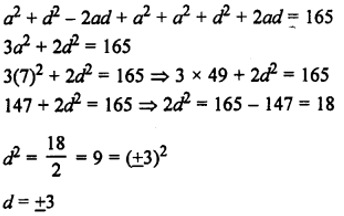Numbers are : (4, 7, 10) or (10, 7, 4)

Question 10:

The angles of a quadrilateral are in AP whose common difference is 10°. Find the angles.

Solution:

Sum of angles of a quadrilateral = 360°

Common difference = 10 = d (say)

If the first number be a, then the next four numbers will be

a, a + 10, a + 20, a + 30

As per definition:

a + a + 10 + a + 20 + a + 30 = 360°

4a + 60 = 360

4a = 300

or a = 75°

Other angles:

a + 10 = 75 + 10 = 85

a + 20 = 75 + 20 = 95

a + 30 = 75 + 30 = 105

Therefore, Angles are 75°, 85°, 95°, 105°

Question 11:

Find four numbers in AP whose sum is 28 and the sum of whose squares is 216.

Solution:

Let a – 3d, a – d, a + d, a + 3d are the four numbers in AP.

Their sum = a – 3d + a – d + a + d + a + 3d = 28

Sum of their square = (a – 3d)^2 + (a – d)^2 + (a + d)^2 + (a + 3d)^2 = 216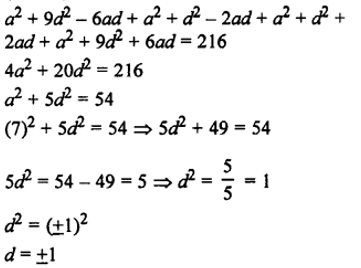Numbers will be: (4, 6, 8,10) or ( 10, 8, 6, 4)

Question 12: Divide 32 into four parts which are the four terms of an AP such that the product of the first and the fourth terms is to the product of the second and the third terms as 7 : 15.

Solution:

Let a – 3d, a – d, a + d, a + 3d are the four numbers in AP.

Therefore: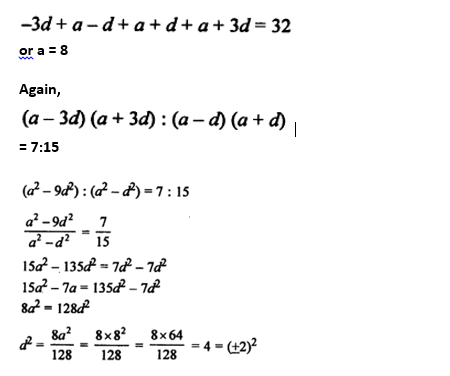d = ±2

four parts are: a – 6, a – 2, a+2 amd a + 6

which implies,

Number are: (2, 6, 8, 10, 14) or (14, 10, 6, 2)

Question 13: The sum of first three terms of an AP is 48. If the product of first and second terms exceeds 4 times the third term by 12. Find the AP.

Solution:

Let a – d, a, a + d are the three terms in AP

So,

Sum = a – d + a + a + d = 48

3a = 48

Or a = 16

And,

a(a – d) = 4 (a + d) + 12

16 (16 – d) = 4(16 + d) + 12

256 – 16d = 64 + 4d + 12 = 4d + 76

256 – 76 = 4d + 16d

180 = 20d

d = 9

Which implies:

Numbers are : (7, 16, 25)

## Exercise 5C Page No: 281

Question 1: Find the sum of each of the following APs:

(i) 2, 7, 12, 17,….. to 19 terms.

(ii) 9, 7, 5, 3,…..to 14 terms.

(iii) -37, -33, -29,….12 terms.

(iv) 1/15 ,1/12,1/10,…to 11 terms

(v) 0.6, 1.7, 2.8,…. to 100 terms.

Solution:

Sum of n terms of AP formula: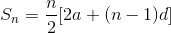……(1)

Where

First term = a

Common difference = d

Number of terms = n

(i) 2, 7, 12, 17,….. to 19 terms.

a = 2

d = 7 – 2 = 5

Using (1)

S_19 = 19/2(2(2) + (19 – 1)5)

= (19)(4 + 90)/2

= (19 × 94)/2

= 893

Sum of 19 terms of this AP is 893.

(ii) 9, 7, 5, 3,…..to 14 terms.

a = 9

d = 7 – 9 – -2

Using (1)

S_14 = 14/2 [2(9) + (14 – 1)(-2)]

= (7)(18 – 26)

= – 56

Sum of 14 terms of this AP is – 56.

(iii) -37, -33, -29,….12 terms.

a = -37

d = (-33) – (-37) = 4

Using (1)

S_12 = 12/2 [2(-37) + (12 – 1)(4)]

= (6)(-74 + 44)

= 6 × (-30)

= – 180

Sum of 12 terms of this AP is – 180.

(iv) 1/15 ,1/12,1/10,…to 11 terms

a = 1/15

d = (1/12) – (1/15) = 1/60

Using (1)

S_11 = 11/2 [2(1/15) + (11 – 1)(1/60)]

= (11/2) × [(2/15) + (1/6)]

=33/20

Sum of 11 terms of this AP is 33/20.

(v) 0.6, 1.7, 2.8,…. to 100 terms.

a = 0.6

d = 1.7 – 0.6 = 1.1

Using (1)

S_100 = 100/2 [2(0.6) + (100 – 1)(1.1)]

= (50) × [1.2 + (99 × 1.1) ]

= 50 × 110.1

= 5505

Sum of 100 terms of this AP is 5505.

Question 2: Find the sum of each of the following arithmetic series:

(i) 7 + 10_1/2 + 14 + … + 84

(ii) 34 + 32 + 30 + … + 10

(iii) (-5) + (-8) + (-11) + … + (-230)

(iv) 5 + (-41) + 9 + (-39) + 13 + (-37) + 17 + … + (-5) + 81 + (-3)

Solution:

(i) 7 + 10_1/2 + 14 + … + 84

First term = a = 7

Common difference = d = (21/2) – 7 = (7/2)

Last term = l = 84

Now, using formula:

84 = a + (n – 1)d

84 = 7 + (n – 1)(7/2)

77 = (n – 1)(7/2)

154 = 7n – 7

7n = 161

n = 23

Thus, there are 23 terms in AP.

Now,

Find Sum of these 23 terms:

S_23 = 23/2 [2(7) + (23 – 1)(7/2)]

= (23/2) [14 + (22)(7/2) ]

= (23/2) 

= 2093/2

Sum of 23 terms of this AP is 2093/2.

(ii) 34 + 32 + 30 + … + 10

First term = a = 34

Common difference = d = 34 – 32 = – 2

Last term = l = 10

Now, using formula:

10 = a + (n – 1)d

10 = 34 + (n – 1)(-2)

10 – 34 = (n – 1)(-2)

n = 13

Thus, there are 13 terms in AP.

Now,

Find Sum of these 13 terms:

S_13 = 13/2 [2(34) + (13 – 1)(-2)]

= (13/2) [68 + (12)(-2)]

= (13/2) x 44

= 286

Sum of 13 terms of this AP is 286.

(iii) (-5) + (-8) + (-11) + … + (-230)

First term = a = -5

Common difference = d = – 8 – (-5) = – 3

Last term = l = -230

Now, using formula:

-230 = a + (n – 1)d

-230 = -5 + (n – 1)(-3)

– 230 + 5 = (n – 1)(-3)

n = 76

Thus, there are 76 terms in AP.

Now,

Find Sum of these 76 terms:

S_76 = 76/2 [2(-5) + (76 – 1)(-3)]

= 38 × [(-10) + (75)(-3)]

= – 8930

Sum of 76 terms of this AP is -8930.

(iv) 5 + (-41) + 9 + (-39) + 13 + (-37) + 17 + … + (-5) + 81 + (-3)

The given series is combination of two AP’s.

Let A_1 = 5 + 9 +13 + ……+ 77 + 81

and A_2 = -41 – 39 – 37 – ……(-3)

For A_1:

First term = a = 5

Common difference = d = 2

Last term = l = 81

Now, using formula:

81 = 5 + (n – 1)4

n = 20

Thus, there are 20 terms in AP.

Now,

Find Sum of these 76 terms:

S_20 = n/2 [a + l]

= 20/2 (5 + 81)

= 860

Sum of 20 terms of this AP is 860.

For A_2:

First term = a = -41

Common difference = d = 2

Last term = l = -3

Now, using formula:

-3 = -41 + (n – 1)2

n = 20

Thus, there are 20 terms in AP.

Now,

Find Sum of these 76 terms:

S_20 = n/2 [a + l]

= 20/2 (-41- 3)

=-440

Sum of 20 terms of this AP is -440.

Therefore, Sum of total terms: 860 – 440 = 420.

Question 3: Find the sum of first n terms of an AP whose nth term is (5 – 6n). Hence, find the sum of its first 20 terms.

Solution:

Given: a_n = 5 – 6n

Find some of the terms of AP:

Put n = 1, we get a_1 = – 1 = first term

Put n = 2, we get a_2 = – 7 = second term

Common difference = d = a_2 – a_1 = – 7 – (-1) = – 6

Sum of first n terms:

S_n= n/2 [2a + (n – 1)d]

= n/2[- 2 + (n – 1)(-6)]

= n(2 – 3n)

sum of first 20 terms:

S_20 = 20/2[2(-1) + (20 – 1)(-6)]

= 10 [ – 2 – 114]

= – 1160

Sum of its first 20 terms of AP is -1160.

Question 4:

The sum of the first n terms of an AP is (3n² + 6n). Find the nth term and the 15th term of this AP.

Solution:

Given: Sn = 3n² + 6n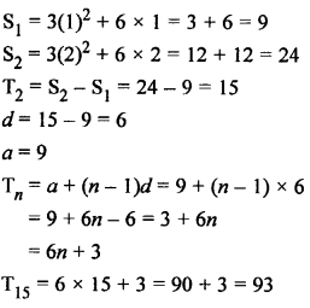Question 5: The sum of the first n terms of an AP is given by Sn = (3n² – n). Find its

(i) nth term,

(ii) first term and

(iii) common difference.

Solution:

S_n = 3n² – n

S_1 = 3(1)² – 1 = 3 – 1 = 2

S_2 = 3(2)² – 2 = 12 – 2 = 10

a_1 = 2

a_2 = 10 – 2 = 8

(i) a_n = a + (n – 1) d

= 2 + (n – 1) x 6

= 2 + 6n – 6

= 6n – 4

(ii) First term = 2

(iii) Common difference = 8 – 2 = 6

Question 6: (i) The sum of the first n terms of an AP is (5n^2/2 + 3n/2). Find the nth term and the 20th term of this AP.

(ii) The sum of the first n terms of an AP is (3n^2/2 + 5n/2). Find its nth term and the 25th term.

Solution: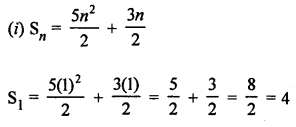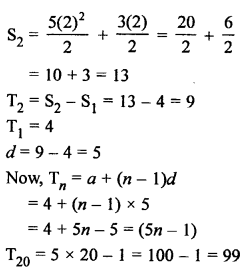(ii)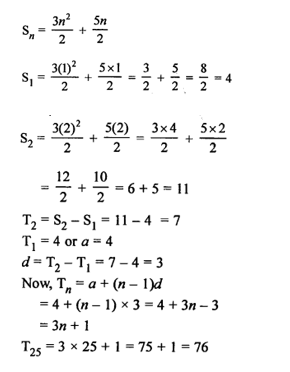Question 7: If mth term of an AP is 1/n and nth term is 1/m then find the sum of its first mn terms.

Solution:

Let a be first term and d be the common difference of an AP.

mth term = 1/n

so,

a_m = a + (m – 1) d = 1/n ……….(1)

nth term = 1/m

a_n = a + (n – 1) d = 1/m ……….(2)

Subtract (2) from (1)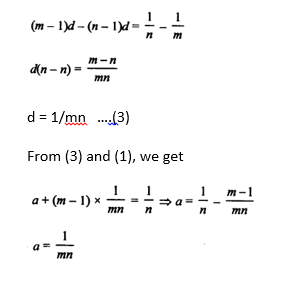Sum of first mn terms:

S_mn = mn/2 [2(1/mn) + (mn-1) (1/mn)]

= mn/2 [1/mn + 1]

= (1+mn)/2

Question 8:

How many terms of the AP 21, 18, 15, … must be added to get die sum 0?

Solution:

AP is 21, 18, 15,…

a = 21,

d = 18 – 21 = -3

Sum of terms = S_n = 0

(n/2) [2a + (n – 1)d] = 0

(n/2) [2(21) + (n – 1)(-3)] = 0

(n/2) [45 – 3n] = 0

[45 – 3n] = 0

n = 15 (number of terms)

Thus, 15 terms of the given AP sums to zero.

Question 9: How many terms of the AP 9, 17, 25, … must be taken so that their sum is 636?

Solution:

AP is 9, 17, 25,…

a = 9, d = 17 – 9 = 8

Sum of terms = S_n = 636

(n/2) [2a + (n – 1)d] = 636

(n/2) [2(9) + (n – 1)(8)] = 636

(n/2)[10 + 8n] = 636

4n^2 + 5n – 636 = 0 (which is a quadratic equation)

(n- 12)(4n + 53) = 0

Either (n- 12) = 0 or (4n + 53) = 0

n = 12 or n = – 53/4

Since n can’t be negative and fraction, so

n = 12

Number of terms = 12 terms.

Question 10: How many terms of the AP 63, 60, 57, 54, … must be taken so that their sum is 693? Explain the double answer.

Solution:

AP is 63, 60, 57, 54,…

Here, a = 63, d = 60 – 63 = -3 and sum = S_n = 693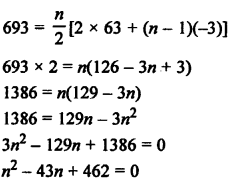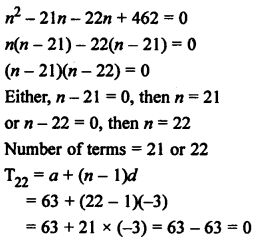Which shows that, 22th term of AP is zero.

Number of terms are 21 or 22. So there will be no effect on the sum.

Question 11:

How many terms of the AP 20, 19_1/3 , 18_2/3 , … must be taken so that their sum is 300? Explain the double answer.

Solution:Here, a = 20, d = -2/3 and sum = s_n = 300 (for n number of terms)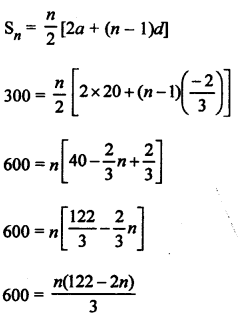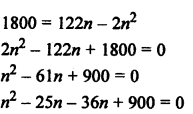n(n-25) – 36(n-25) = 0

(n – 25)(n – 36) = 0

Either (n – 25) = 0 or (n – 36) = 0

n = 25 or n = 36

For n = 25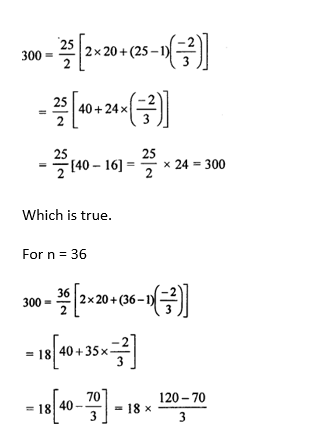= 300

Which is true.

Result is true for both the values of n

So both the numbers are correct.

Therefore, Sum of 11 terms is zero. (36-25 = 11)

Question 12: Find the sum of all odd numbers between 0 and 50.

Solution:

Odd numbers between 0 and 50 are 1, 3, 5, 7, 9, …, 49

Here, a = 1, d = 3 – 1 = 2, l = 49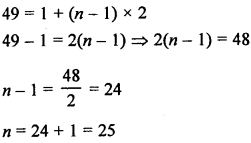Sum of odd numbers:

= n/2(a + l)

= 25/2 (l + 49)

= 25/2(50)

= 625

Question 13: Find the sum of all natural numbers between 200 and 400 which are divisible by 7.

Solution:

Natural numbers between 200 and 400 which are divisible by 7 are 203, 210, 217,……,399.

Here,AP is 203, 210, 217,……,399

First term = a = 203,

Common difference = d = 7 and

Last term = l = 399

We know that, l = a + (n-1)d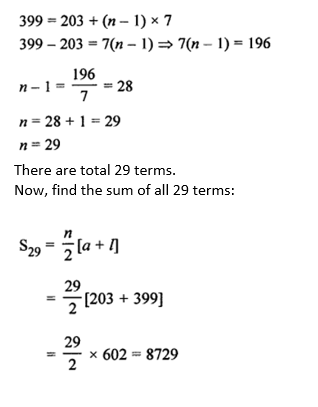Sum of all 29 terms is 8729.

Question 14: Find the sum of first forty positive integers divisible by 6.

Solution:

First forty positive integers which are divisible by 6 are

6, 12, 18, 24,…… to 40 terms

Here, a = 6, d = 12 – 6 = 6, and n = 40.

Sum of 40 terms: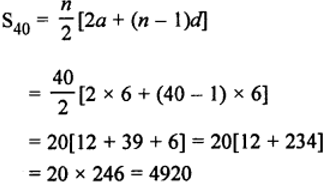Question 15: Find the sum of the first 15 multiples of 8.

Solution:

First 15 multiples of 8 are as given below:

8, 16, 24, 32,….. to 15 terms

Here, a = 8, d = 16 – 8 = 8, and n = 15

Sum of 15 terms: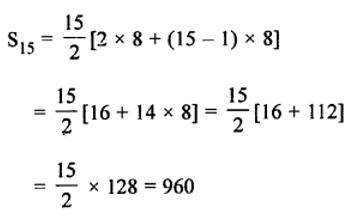Question 16: Find the sum of all multiples of 9 lying between 300 and 700.

Solution:

Multiples of 9 lying between 300 and 700 = 306, 315, 324, 333, …, 693

Here, a = 306, d = 9, and l = 693

We know that, l = a + (n-1)d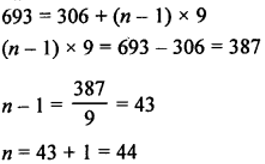There are 44 terms.

Find the sum of 44 terms: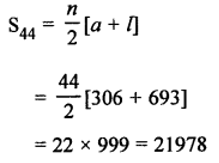Question 17: Find the sum of all three-digit natural numbers which are divisible by 13.

Solution:

3-digit natural numbers: 100, 101, 102,……., 999.

3-digit natural numbers divisible by 13:

104, 117, 130,………, 988

Which is an AP.

Here, a = 104, d = 13, l = 988

l = a + (n – 1) d

988 = 104 + (n-1)13

n = 69

There are 69 terms.

Find the sum of 69 terms:

S_n = n/2(a + l)

= 69/2(104 + 988)

= 37674

Question 18: Find the sum of first 100 even natural numbers which are divisible by 5.

Solution:

Even natural numbers: 2, 4, 6, 8, 10, …

Even natural numbers divisible by 5: 10, 20, 30, 40, … to 100 terms

Here, a = 10, d = 20 – 10 = 10, and n = 100

S_n = n/2(2a + (n-1)d)

= 100/2 [2 x 10 + (100-1)10]

= 50500

Question 19: Find the sum of the following.

(4 – 1/n) + (4-2/n) + (4– 3/n) + ….

Solution:

Given sum can be written as (4 + 4 + 4 + 4+….) – (1/n, 2/n, 3/n, …..)

Now, We have two series:

First series: = 4 + 4 + 4 + …… up to n terms

= 4n

Second series: 1/n, 2/n, 3/n, …..

Here, first term = a = 1/n

Common difference = d = (2/n) – (1/n) = (1/n)

Sum of n terms formula:

S_n = n/2[2a + (n – 1)d]

Sum of n terms of second series:

S_n = n/2 [2(1/n) + (n – 1)(1/n)]

= n/2 [(2/n) + 1 – (1/n)]

= (n + 1)/2

Hence,

Sum of n terms of the given series = Sum of n terms of first series – Sum of n terms of second series

= 4n – (n + 1)/2

= (8n – n – 1)/2

= 1/2 (7n – 1)

Question 20: In an AP, it is given that S_5 + S_7 = 167 and S_10 = 235, then find the AP, where S_n denotes the sum of its first n terms.

Solution:

Let a be the first term and d be the common difference of the AP.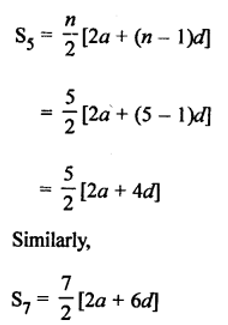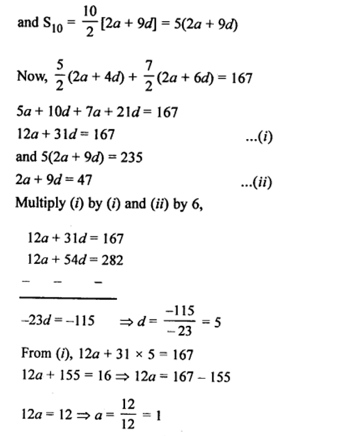Which implies: a = 1 and d = 5

Therefore, the AP is 1, 6, 11, 16,……

Question 21: In an AP, the first term is 2, the last term is 29 and the sum of all the terms is 155. Find the common difference.

Solution:

Let d be the common difference.

Given:

first term = a = 2

last term = l = 29

Sum of all the terms = S_n = 155

S_n = n/2[a + l]

155 = n/2[2 + 29]

n = 10

There are 10 terms in total.

Therefore, 29 is the 10th term of the AP.

Now, 29 = a + (10 – 1)d

29 = 2 + 9d

27 = 9d

d = 3

The common difference is 3.

Question 22: In an AP, the first term is -4, the last term is 29 and the sum of all its terms is 150. Find its common difference.

Solution:

Let d be the common difference.

Given:

first term = a = -4

last term = l = 29

Sum of all the terms = S_n = 150

S_n = n/2[a + l]

150 = n/2[-4 + 29]

n = 12

There are 12 terms in total.

Therefore, 29 is the 12th term of the AP.

Now, 29 = -4 + (12 – 1)d

29 = -4 + 11d

d = 3

The common difference is 3.

Question 23: The first and the last terms of an AP are 17 and 350 respectively. If the common difference is 9, how many terms are there and what is their sum?

Solution:

Let n be the total number of terms.

Given:

First term = a = 17

Last term = l = 350

Common difference = d = 9

l = a + (n-1)d

350 = 17 + (n-1)9

n = 38

Again,

S_n = n/2[a + l]

= 38/2[17 + 350]

= 6973

There are 38 terms in total and their sum is 6973.

Question 24: The first and the last terms of an AP are 5 and 45 respectively. If the sum of all its terms is 400, find the common difference and the number of terms.

Solution:

Let n be the total number of terms and d be the common difference.

Given:

first term = a = 5

last term = l = 45

Sum of all terms = S_n = 400

S_n = n/2[a + l]

400 = n/2[5 + 45]

n/2 = 400/50

n = 16

There are 16 terms in the AP.

Therefore, 45 is the 16th term of the AP.

45 = a + (16 – 1)d

45 = 5 + 15d

40 = 15d

15d = 40

d = 8/3

Common difference = d = 8/3

Common difference is 8/3 and the number of terms are 16.

Question 25: In an AP, the first term is 22, nth term is -11 and sum of first n terms is 66. Find n and hence find the common difference.

Solution:

Let n be the total number of terms and d be the common difference.

Given:

first term = a = 22

nth term = -11

Sum of all terms = S_n = 66

S_n = n/2[a + l]

66 = n/2[22 + (-11)]

n = 12

There are 12 terms in the AP.

Since nth term is -11, so

a_n = a + (n – 1)d

-11 = 22 + (12-1)d

d = -3

Therefore, Common difference is -3 and the number of terms are 12.

## Exercise 5D Page No: 289

Question 1: The first three terms of an AP are respectively (3y – 1), (3y + 5) and (5y + 1), find the value of y.

Solution:

Given: (3y – 1), (3y + 5) and (5y+ 1) are in AP

So, (3y + 5) – (3y – 1) = (5y + 1) – (3y + 5)

2 (3y + 5) = (5y + 1) + (3y – 1)

6y + 10 = 8y

8y – 6y = 10

2y = 10

Or y = 5

The value of y is 2.

Question 2: If k, (2k – 1) and (2k + 1) are the three successive terms of an AP, find the value of k.

Solution:

Given: k, (2k – 1) and (2k + 1) are the three successive terms of an AP.

So, (2k – 1) – k = (2k + 1) – (2k – 1)

2 (2k – 1) = 2k + 1 + k

4k – 2 = 3k + 1

4k – 3k = 1 + 2

or k = 3

The value of k is 3.

Question 3: If 18, a, (b – 3) are in AP, then find the value of (2a – b).

Solution:

Given: 18, a, (b – 3) are in AP

a – 18 = b – 3 – a

a + a – b = -3 + 18

2a – b = 15. Answer!

Question 4: If the numbers a, 9, b, 25 form an AP, find a and b.

Solution:

Given: a, 9, b, 25 are in AP.

So, 9 – a = b – 9 = 25 – b

b – 9 = 25 – b

b + b = 22 + 9 = 34

or b = 17

And,

a – b = a – 9

9 + 9 = a + b

a + b = 18

a + 17 = 18

or a = 1

Answer: a = 18, b= 17

Question 5: If the numbers (2n – 1), (3n + 2) and (6n – 1) are in AP, find the value of n and the numbers.

Solution:

Given: (2n – 1), (3n + 2) and (6n – 1) are in AP

So, (3n + 2) – (2n – 1) = (6n – 1) – (3n + 2)

(3n + 2) + (3n + 2) = 6n – 1 + 2n – 1

6n + 4 = 8n – 2

8n – 6n = 4 + 2

Or n = 3

Numbers are:

2 x 3 – 1 = 5

3 x 3 + 2 = 11

6 x 3 – 1 = 17

Answer: (5, 11, 17) are required numbers.

Question 6: How many three-digit natural numbers are divisible by 7?

Solution:

3-digit natural numbers: 100, 101,…….. 990 and

3-digit natural numbers divisible by 7: 105, 112, 119, 126, …, 994\

Here, a = 105, d= 7, l = 994

a_n = (l) = a + (n – 1) d

994 = 105 + (n – 1) x 7

994 – 105 = (n – 1) 7

n – 1 = 127

n = 128

Answer: There are 128 required numbers.

Question 7: How many three-digit natural numbers are divisible by 9?

Solution:

3-digit numbers: 100, 101,…….,999

3-digit numbers divisible by 9 : 108, 117, 126, 135, …, 999

Here, a = 108, d= 9, l = 999

a_n (l) = a + (n – 1) d

999 = 108 + (n – 1) x 9

(n – 1) x 9 = 999 – 108 = 891

n – 1 = 99

n = 100

Question 8: If the sum of first m terms of an AP is (2m² + 3m) then what is its second term?

Solution:

Sum of first m terms of an AP = 2m² + 3m (given)

S_m = 2m² + 3m

Sum of one term = S_1 = 2(1)² + 3 x 1 = 2 + 3 = 5 = first term

Sum of first two terms = S_2 = 2(2)² + 3 x 2 = 8 + 6=14

Sum of first three terms = S_3 = 2(3)² + 3 x 3 = 18 + 9 = 27

Now,

Second term = a_2 = S_2 – S_1 = 14 – 5 = 9

Question 9: What is the sum of first n terms of the AP, a, 3a, 5a,………….

Solution:

AP is a, 3a, 5a,…..

Here, a = a, d = 2a

Sum = S_n = n/2 [2a + (n-1)d]

= n/2[2a + 2an – 2a]

=an^2

Question 10.: What is the 5th term from the end of the AP 2, 7, 12, ……… 47?

Solution:

Given AP is 2, 7, 12, 17, …… 47

Here, a = 2, d = 7 – 2 = 5, l = 47

nth term from the end = l – (n – 1 )d

5th term from the end = 47 – (5 – 1) 5 = 47 – 4 x 5 = 27

Question 11: If an denotes the nth term of the AP 2, 7, 12, 17, …, find the value of (a_30 – a_20).

Solution:

Given AP is 2, 7, 12, 17,……..

Here, a = 2, d = 7 – 2 = 5

Now,

a_n = a + (n – 1) d = 2 + (n – 1) 5 = 5n – 3

a_30 = 2 + (30 – 1) 5 = 2 + 145 = 147 and

a_20 = 2 + (20 – 1) 5 = 2 + 95 = 97

a_30 – a_20 = 147 – 97 = 50

Question 12: The nth term of an AP is (3n + 5). Find its common difference.

Solution:

Nth term = a_n = 3n + 5 (given)

a_(n-1) = 3 (n – 1) + 5 = 3n + 2

Common difference = d = a_n – a_(n-1)

= (3n + 5) – (3n + 2)

= 3n + 5 – 3n – 2

= 3

Therefore, common difference is 3.

Question 13: The nth term of an AP is (7 – 4n). Find its common difference.

Solution:

Nth term = a_n = 7 – 4n

a_(n-1) = 7 – 4(n – 1) = 11 – 4n

Common difference = d = a_n – a_(n-1)

= (7 – 4n) – (11 – 4n)

= 7 – 4n – 11 + 4n

= -4

Therefore, common difference is -4.

Question 14: Write the next term of the AP √8, √18, √32, ……..

Solution:

Given AP is √8, √18, √32,……..

Above AP can be written as:

2√2 , 3√2, 4 √2, ……

Here a = 2√2 and d = √2

Next term = 4√2 + √2 = 5√2 = √50

Question 15: Write the next term of the AP √2, √8, √18,….

Solution:

Given AP is √2, √8, √18,….

Can be written as:

√2, 2√2, 3√2,…..

First term = √2

Common difference = 2√2 – √2 = √2

Next term = 3√2 + √2 = 4√2 = √32

Question 16: Which term of the AP 21, 18, 15,…. is zero?

Solution:

Given AP is 21, 18, 15,….

First term = a = 21

Common difference = d = 18-21 = -3

Last term = l = 0

l = a + (n – 1) d

0 = 21 + (n – 1)(-3)

0 = 21 – 3n + 3

24 – 3n = 0

Or n = 8

Answer: Zero is the 8th term.

Question 17: Find the sum of first n natural numbers.

Solution:

First n natural numbers: 1, 2, 3, 4, 5, …, n

Here, a = 1, d = 1

Sum = S_n = n/2 [2a + (n-1)d]

= n/2 [2(1) + (n-1)(1)]

= n(n+1)/2

Question 18: Find the sum of first n even natural numbers.

Solution:

First n even natural numbers: 2, 4, 6, 8, 10,….,n

Here, a = 2, d = 4 – 2 = 2

Sum = S_n = n/2 [2a + (n-1)d]

= n/2 [2(2) + (n-1)(2)]

= n(n+1)

Question 19: The first term of an AP is p and its common difference is q. Find its 10th term.

Solution:

Given:

First term =a = p and

Common difference = d =q

Now,

a_10 = a + (n – 1) d

= p + (10 – 1)q

= (p + 9q)

Question 20: If 4/5, a, 2 are in AP, find the value of a.

Solution:

AP terms: 4/5, a, 2 (given)

Then,

a – 4/5 = 2 – a

a = 7/5

Question 21: If (2p + 1), 13, (5p – 3) are in AP, find the value of p.

Solution:

Given, 2p + 1, 13, 5p – 3 are in AP

Then,

13 – (2p + 1) = (5p – 3) – 13

13 – 2p – 1 = 5p – 3 – 13

12 – 2p = 5p – 16

p = 4

The value of p is 4.

Question 22: If (2p – 1), 7, 3p are in AP, find the value of p.

Solution:

Given, (2p – 1), 7, 3p are in AP

Then,

7 – (2p – 1) = 3p – 7

7 – 2p + 1 = 3p – 7

5p = 15

p = 3

The value of p is 3.

Question 23: If the sum of first p terms of an AP is (ap² + bp), find its common difference.

Solution:

Sum of first p terms = S_p = (ap² + bp)

Sum of one term = S_1 = a(1)² + b(1) = a+b = first term

Sum of first two terms = S_2 = a(2)² + b x 2 = 4a + 2b

We know that, second term = a_2 = S_2 – S_1

= (4a + 2b) – (a + b)

= 3a + b

Now, d = a_2 – a_1

= 3a + b – (a+b)

= 2a

Question 24: If the sum of first n terms is (3n² + 5n), find its common difference.

Solution:

Sum of first n terms = S_n = (3n² + 5n)

Sum of one term = S_1 = 3(1)² + 5(1) = 8 = first term

Sum of first two terms = S_2 = 3(2)² + 5(2) = 22

We know that, second term = a_2 = S_2 – S_1

=22 – 8

= 14

=> a_2 = 14

Now, d = a_2 – a_1

= 14 – 8 = 6

Question 25: Find an AP whose 4th term is 9 and the sum of its 6th and 13th terms is 40.

Solution:

Let a be the first term and d be the common difference.

Given:

4th term = a_4 = 9

Sum of 6th and 13th terms = a_6 + a_13 = 40

Now,

a_4 = a + (4-1)d

9 = a + 3d

a = 9 – 3d ….(1)

And

a_6 + a_13 = 40

a + 5d + a + 12d = 40

2a +17d = 40

2(9 – 3d) + 17d = 40 (using (1))

d = 2

From (1): a = 9 – 6 = 3

Required AP = 3,5,7,9,…..

Question 26: What is the common difference of an AP in which a_27 – a_7 = 84?

Solution:

Given: a_27 – a_7 = 84

[a + 26d] – [a + 6d] = 84

20d = 84

d = 4.2

Question 27: If 1 + 4 + 7 + 10 + … + x = 287, find the value of x.

Solution:

Given: 1 + 4 + 7 + 10 + … + x = 287

Here a = 1, d = 3 and S_n = 287

Sum = S_n = n/2 [2a + (n-1)d]

287 = n/2 [2 + (n-1)3]

574 = 3n^2 – n

Solve 3n^2 – n – 574 = 0

3n^2 – 42n + 41n – 574 = 0

3n(n – 14) + 41(n-14) = 0

(3n + 41)(n-14) = 0

Either (3n + 41) = 0 or (n-14) = 0

n = -41/3 or n = 14

Since number of terms cannot be negative, so result is n = 14.

=> Total number of terms in AP are 14.

Which shows, x = a_14

or x = a + 13d

or x = 1 + 39

or x = 40

The value of x is 40.

## R S Aggarwal Solutions for Chapter 5 Arithmetic Progression

In this chapter students will study important concepts on arithmetic progression as listed below:

• Arithmetic Progression introduction
• Arithmetic series
• Finding the general term of an AP
• Finding the nth term from the end of an AP
• Arithmetic Mean
• Sum of n terms of an AP

### Key Features of R S Aggarwal Solutions for Class 10 Maths Chapter 5 Arithmetic Progression

1. R S Aggarwal Solutions for Maths is easy to comprehend and covers all the exercise questions.

2. R S Aggarwal textbook helps to get to know more about various concepts on Arithmetic Progression.

3. Step-by-step problem solving approach helps students to understand easily.

4. Easy for quick revision.

5. Prepared based on the latest CBSE syllabus by subject experts.